# Chapter 2 A Single Heatmap

A single heatmap is the most used approach for visualizing the data. Although “the shining point” of the ComplexHeatmap package is it can visualize a list of heatmaps in parallel, as the basic unit of the heatmap list, it is still very important to have the single heatmap nicely configured.

First let’s generate a random matrix where there are three groups by columns and three groups by rows:

set.seed(123)
nr1 = 4; nr2 = 8; nr3 = 6; nr = nr1 + nr2 + nr3
nc1 = 6; nc2 = 8; nc3 = 10; nc = nc1 + nc2 + nc3
mat = cbind(rbind(matrix(rnorm(nr1*nc1, mean = 1,   sd = 0.5), nr = nr1),
matrix(rnorm(nr2*nc1, mean = 0,   sd = 0.5), nr = nr2),
matrix(rnorm(nr3*nc1, mean = 0,   sd = 0.5), nr = nr3)),
rbind(matrix(rnorm(nr1*nc2, mean = 0,   sd = 0.5), nr = nr1),
matrix(rnorm(nr2*nc2, mean = 1,   sd = 0.5), nr = nr2),
matrix(rnorm(nr3*nc2, mean = 0,   sd = 0.5), nr = nr3)),
rbind(matrix(rnorm(nr1*nc3, mean = 0.5, sd = 0.5), nr = nr1),
matrix(rnorm(nr2*nc3, mean = 0.5, sd = 0.5), nr = nr2),
matrix(rnorm(nr3*nc3, mean = 1,   sd = 0.5), nr = nr3))
)
mat = mat[sample(nr, nr), sample(nc, nc)] # random shuffle rows and columns
rownames(mat) = paste0("row", seq_len(nr))
colnames(mat) = paste0("column", seq_len(nc))

Following command contains the minimal argument for the Heatmap() function which just visualizes the matrix as a heatmap with default settings. Very similar as other heatmap tools, it draws the dendrograms, the row/column names and the heatmap legend. The default color schema is “blue-white-red” which is mapped to the minimal-mean-maximal values in the matrix. The title for the legend is assigned with an internal index number.

Heatmap(mat)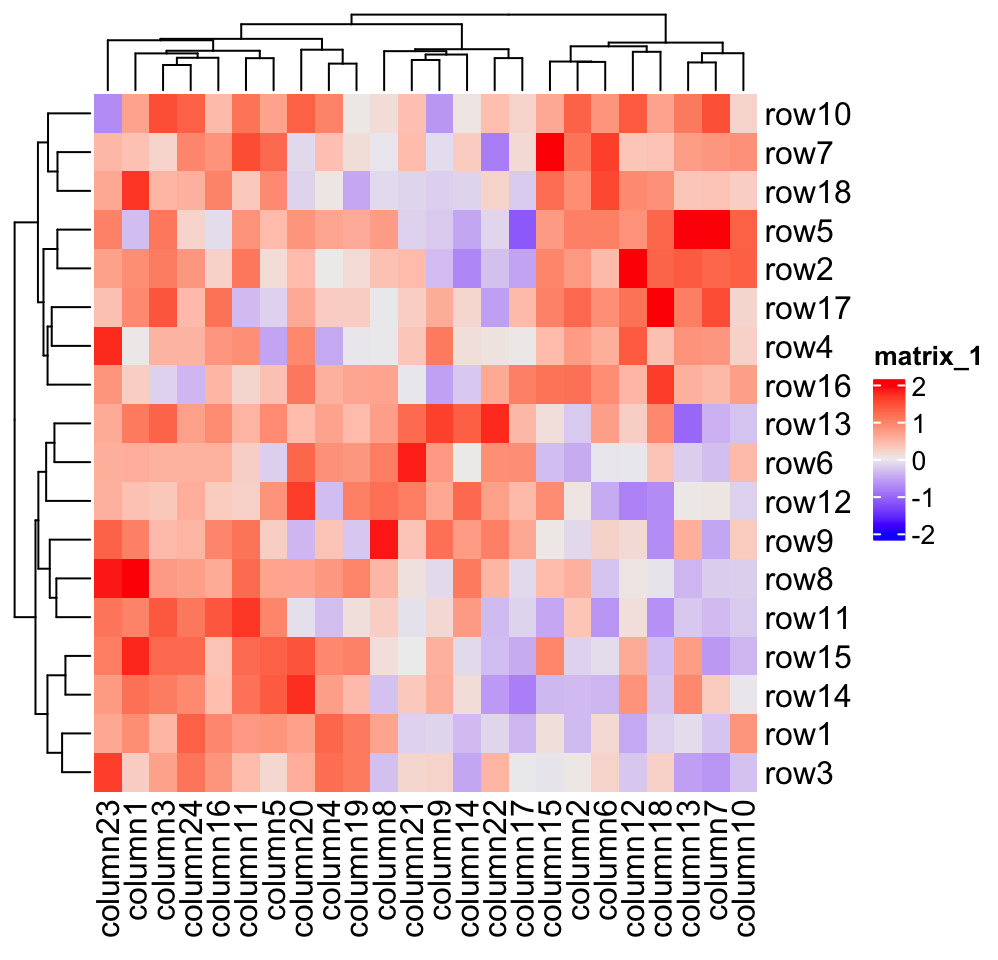The title for the legend is taken from the “name” of the heatmap by default. Each heatmap has a name which is like a unique identifier for the heatmap and it is important when you have a list of heatmaps. In later chapters, you will find the heatmap name is used for setting the “main heatmap” and is used for decoration of heatmaps. If the name is not assigned, an internal name is assigned to the heatmap in a form of matrix_%d. In following examples in this chapter, we give the name mat to the heatmap (for which you will see the change of the legend in the next plot).

If you put Heatmap() inside a function or a for/if/while chunk, you won’t see the heatmap after executing Heatmap(). In this case, you need to use draw() function explicitly as follows. We will explain this point in more detail in Section 2.11.

ht = Heatmap(mat)
draw(ht)

## 2.1 Colors

For heatmap visualization, colors are the major representation of the data matrix. In most cases, the heatmap visualizes a matrix with continuous numeric values. In this case, users should provide a color mapping function. A color mapping function should accept a vector of values and return a vector of corresponding colors. Users should always use circlize::colorRamp2() function to generate the color mapping function with using Heatmap(). The two arguments for colorRamp2() is a vector of break values and a vector of corresponding colors. colorRamp2() linearly interpolates colors in every interval through LAB color space. Also using colorRamp2() helps to generate a legend with proper tick marks.

In following example, values between -2 and 2 are linearly interpolated to get corresponding colors, values larger than 2 are all mapped to red and values less than -2 are all mapped to green.

library(circlize)
col_fun = colorRamp2(c(-2, 0, 2), c("green", "white", "red"))
col_fun(seq(-3, 3))
##  "#00FF00FF" "#00FF00FF" "#B1FF9AFF" "#FFFFFFFF" "#FF9E81FF" "#FF0000FF"
##  "#FF0000FF"
Heatmap(mat, name = "mat", col = col_fun)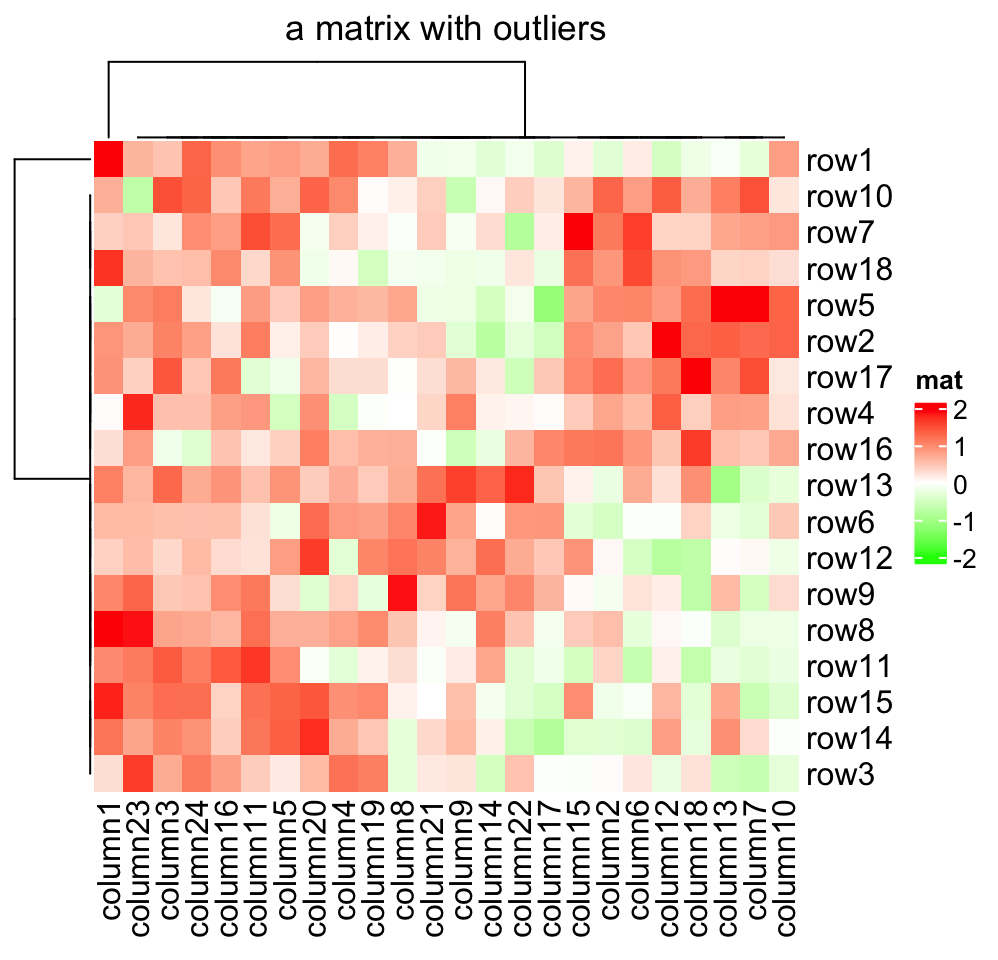As you can see, the color mapping function exactly maps negative values to green and positive values to red, even when the distribution of negative values and positive values are not centric to zero. Also this color mapping function is not affected by outliers. In following plot, the clustering is heavily affected by the outlier but not the color mapping.

mat2 = mat
mat2[1, 1] = 100000
Heatmap(mat2, name = "mat", col = col_fun)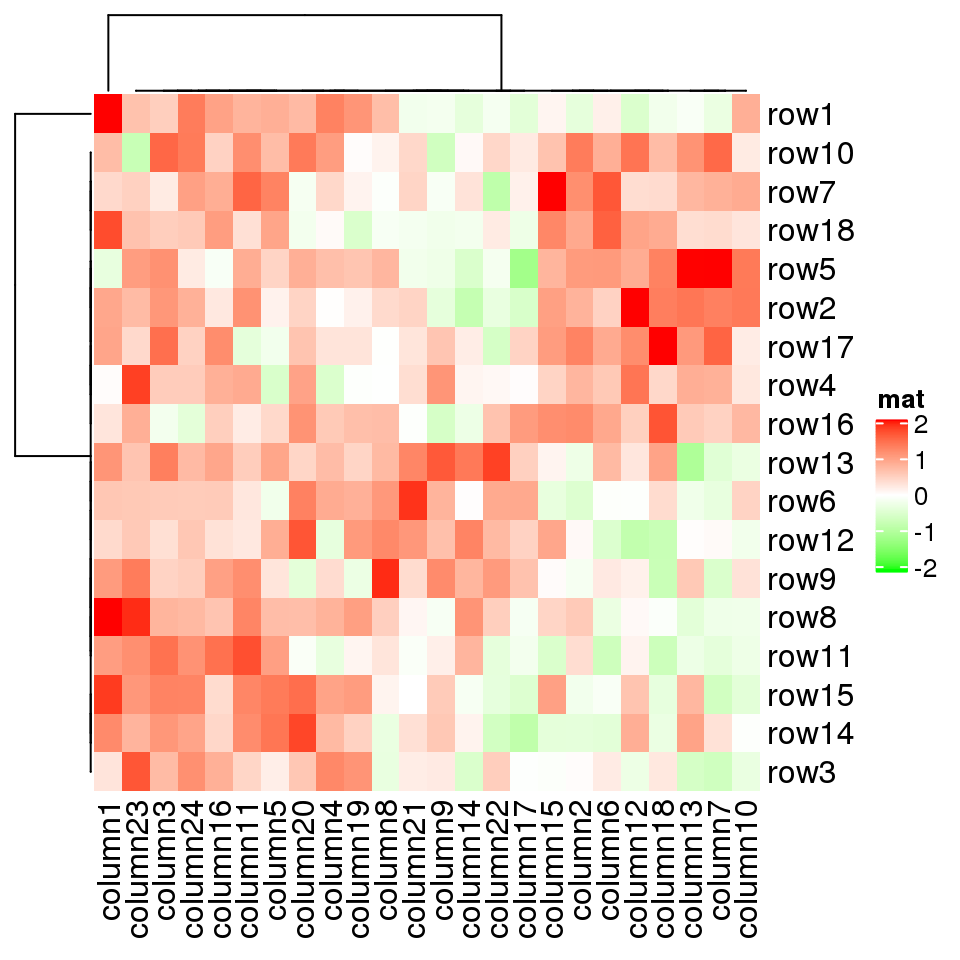More importantly, colorRamp2() makes colors in multiple heatmaps comparible if they are set with a same color mapping function. In following three heatmaps, a same color always corresponds to a same value.

Heatmap(mat, name = "mat", col = col_fun, column_title = "mat")
Heatmap(mat/4, name = "mat", col = col_fun, column_title = "mat/4")
Heatmap(abs(mat), name = "mat", col = col_fun, column_title = "abs(mat)")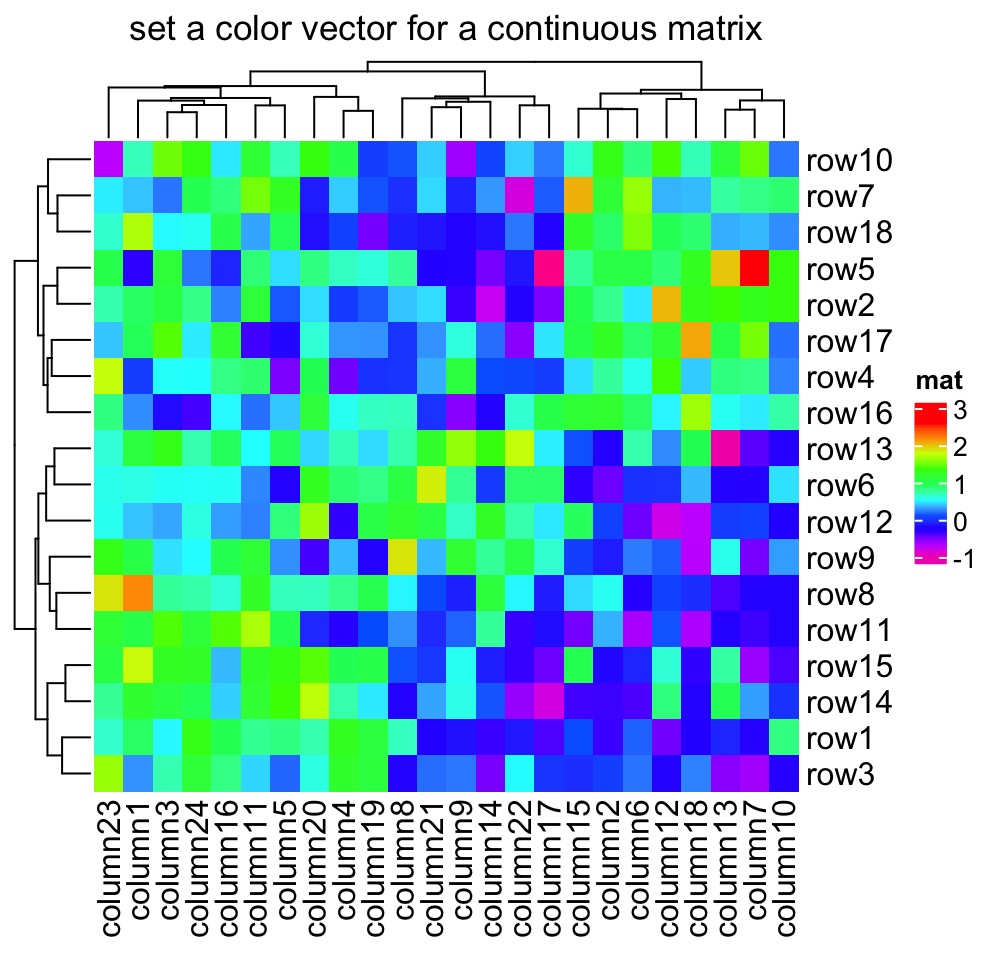If the matrix is continuous, you can also simply provide a vector of colors and colors will be linearly interpolated. But remember this method is not robust to outliers because the mapping starts from the minimal value in the matrix and ends with the maximal value. Following color mapping setting is identical to colorRamp2(seq(min(mat), max(mat), length = 10), rev(rainbow(10))).

Heatmap(mat, name = "mat", col = rev(rainbow(10)))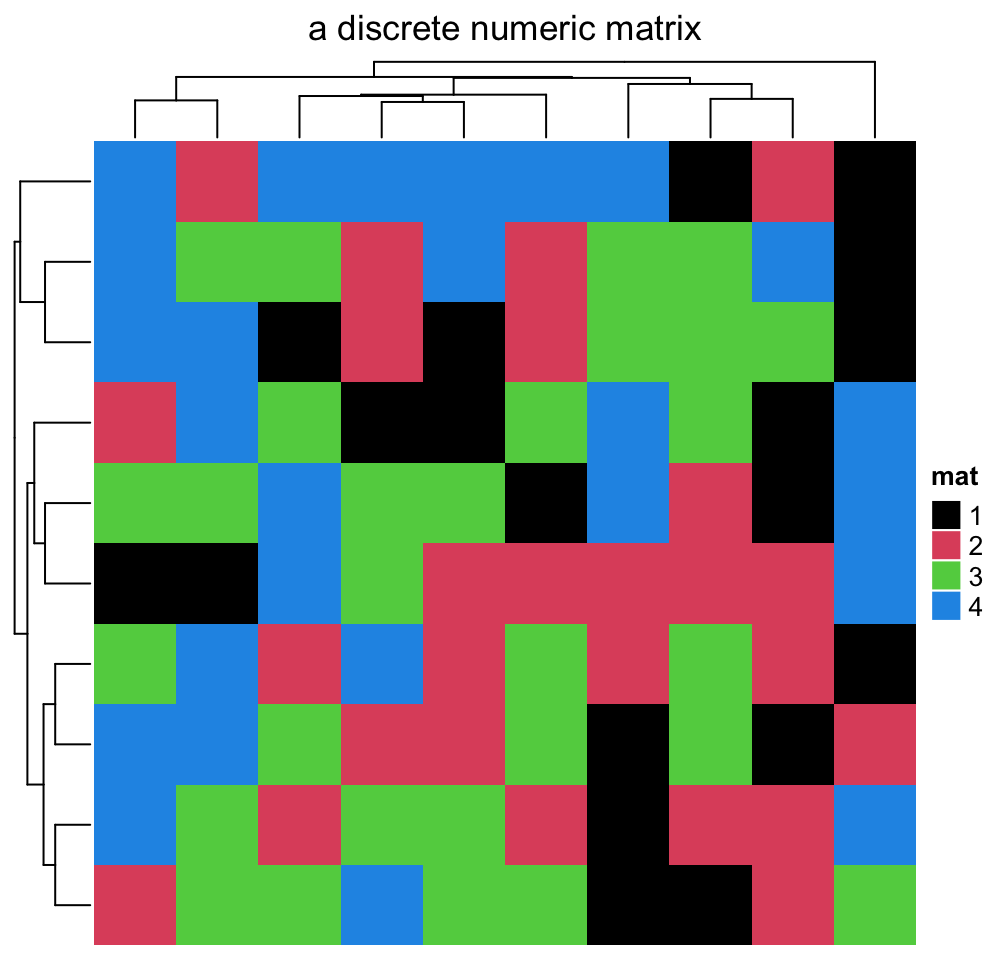If the matrix contains discrete values (either numeric or character), colors should be specified as a named vector to make it possible for the mapping from discrete values to colors. If there is no name for the color, the order of colors corresponds to the order of unique(mat). Note now the legend is generated from the color mapping vector.

Following sets colors for a discrete numeric matrix (you don’t need to convert it to a character matrix).

discrete_mat = matrix(sample(1:4, 100, replace = TRUE), 10, 10)
colors = structure(1:4, names = c("1", "2", "3", "4")) # black, red, green, blue
Heatmap(discrete_mat, name = "mat", col = colors)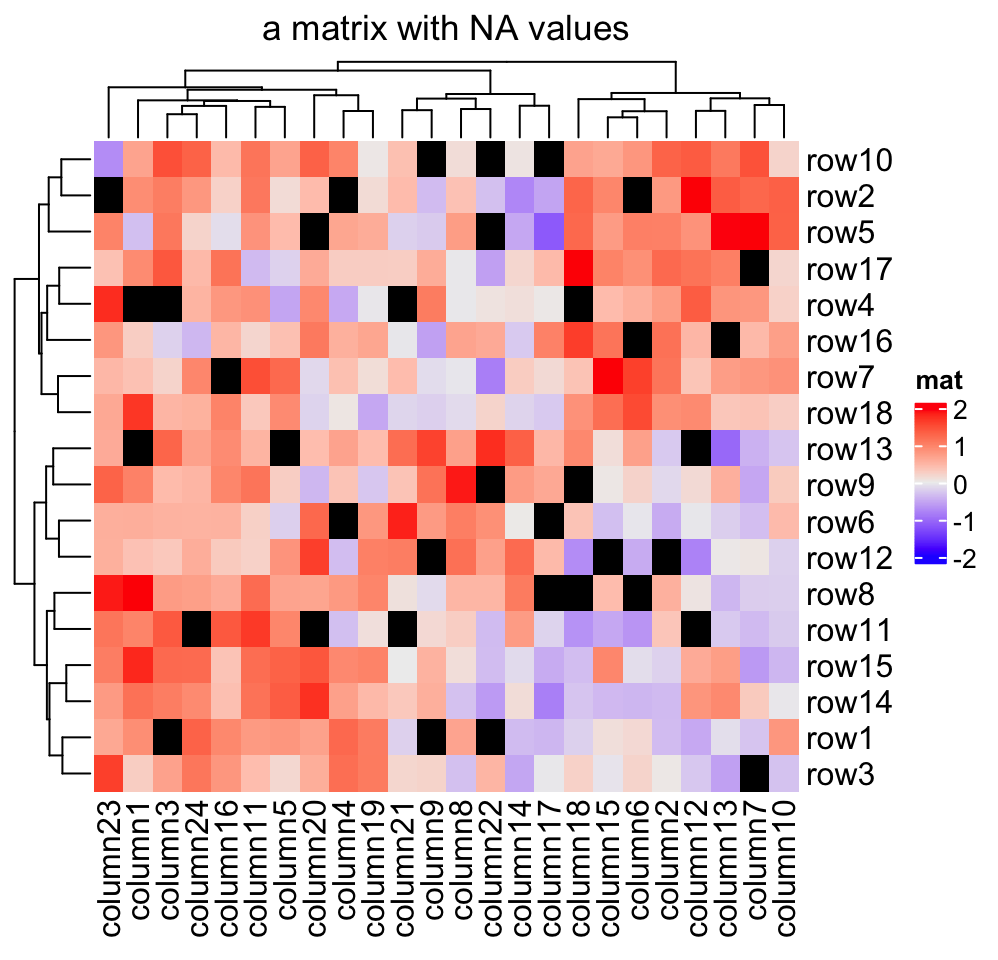Or a character matrix:

discrete_mat = matrix(sample(letters[1:4], 100, replace = TRUE), 10, 10)
colors = structure(1:4, names = letters[1:4])
Heatmap(discrete_mat, name = "mat", col = colors)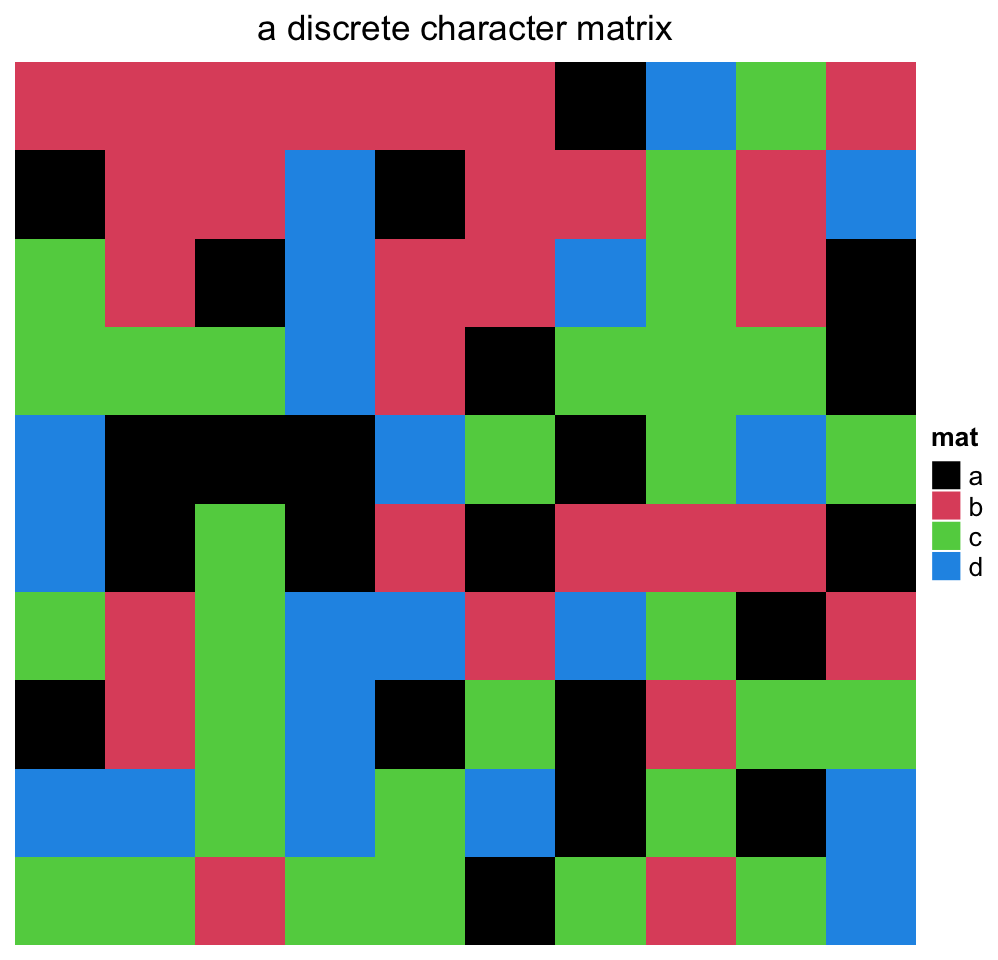As you see in the two examples above, for the numeric matrix (no matter the color is continuous mapping or discrete mapping), by default clustering is applied on both dimensions while for character matrix, clustering is turned off (but you can still cluster a character matrix if you provide a proper distance metric for two character vectors, see example in Section 2.3.1).

NA is allowed in the matrix. You can control the color of NA by na_col argument (by default it is grey for NA). The matrix that contains NA can be clustered by Heatmap().

Note the NA value is not presented in the legend.

mat_with_na = mat
na_index = sample(c(TRUE, FALSE), nrow(mat)*ncol(mat), replace = TRUE, prob = c(1, 9))
mat_with_na[na_index] = NA
Heatmap(mat_with_na, name = "mat", na_col = "black")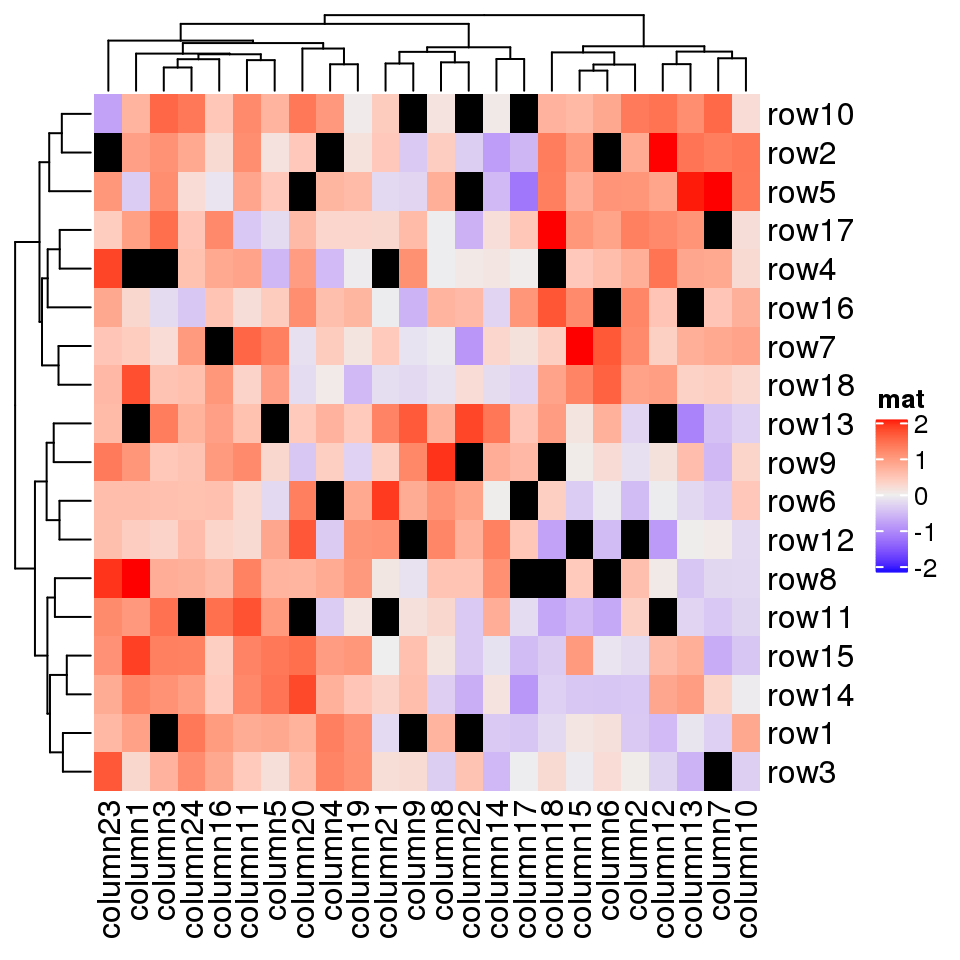Color space is important for interpolating colors. By default, colors are linearly interpolated in LAB color space, but you can select the color space in colorRamp2() function. Compare following two plots. Can you see the difference?

f1 = colorRamp2(seq(min(mat), max(mat), length = 3), c("blue", "#EEEEEE", "red"))
f2 = colorRamp2(seq(min(mat), max(mat), length = 3), c("blue", "#EEEEEE", "red"),
space = "RGB")
Heatmap(mat, name = "mat1", col = f1, column_title = "LAB color space")
Heatmap(mat, name = "mat2", col = f2, column_title = "RGB color space")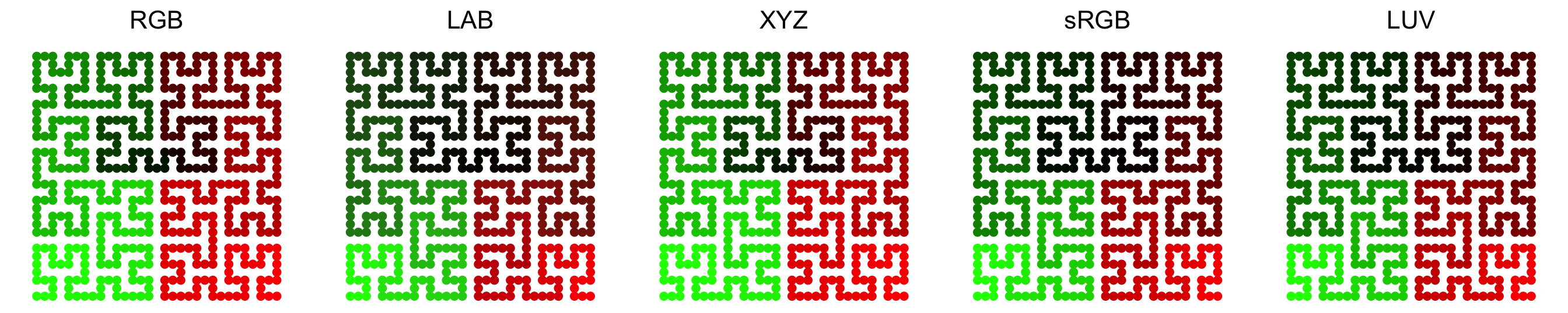In following plots, corresponding values change evenly on the folded lines, you can see how colors change under different color spaces (top plots: green-black-red, bottom plots: blue-white-red. The plot is made by HilbertCurvepackage).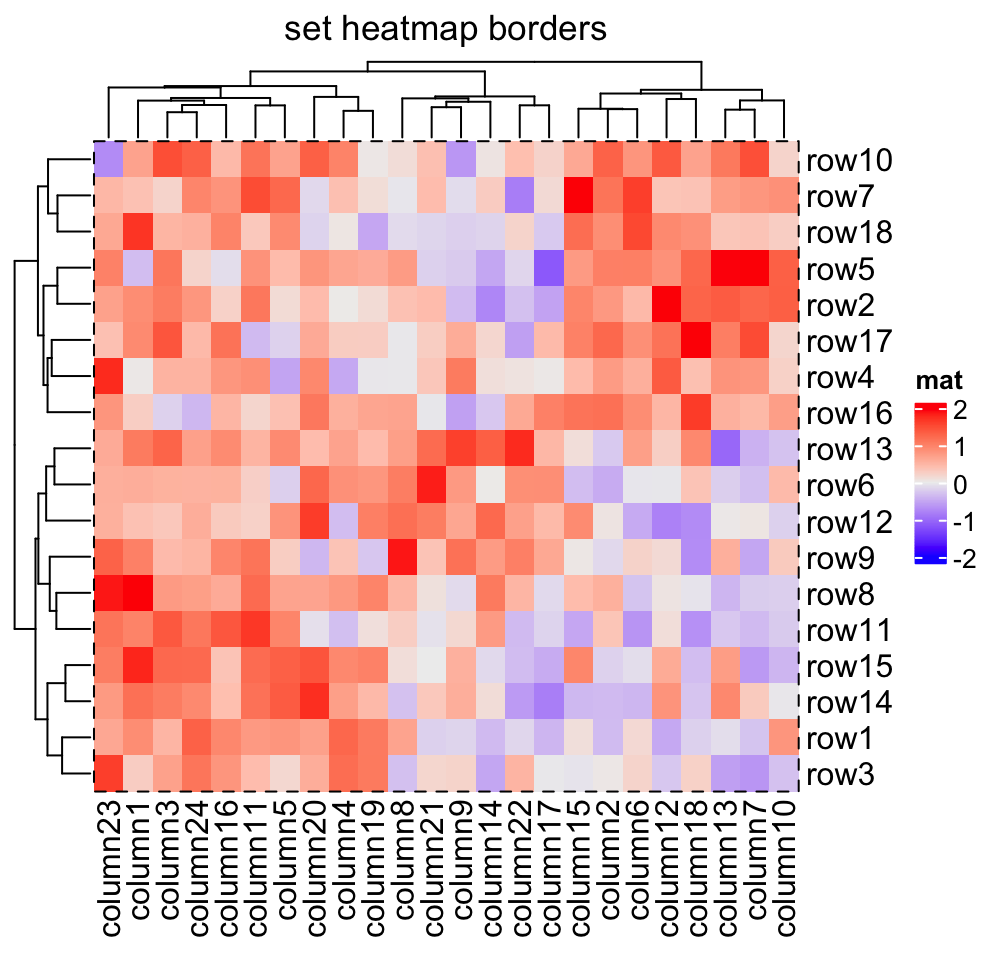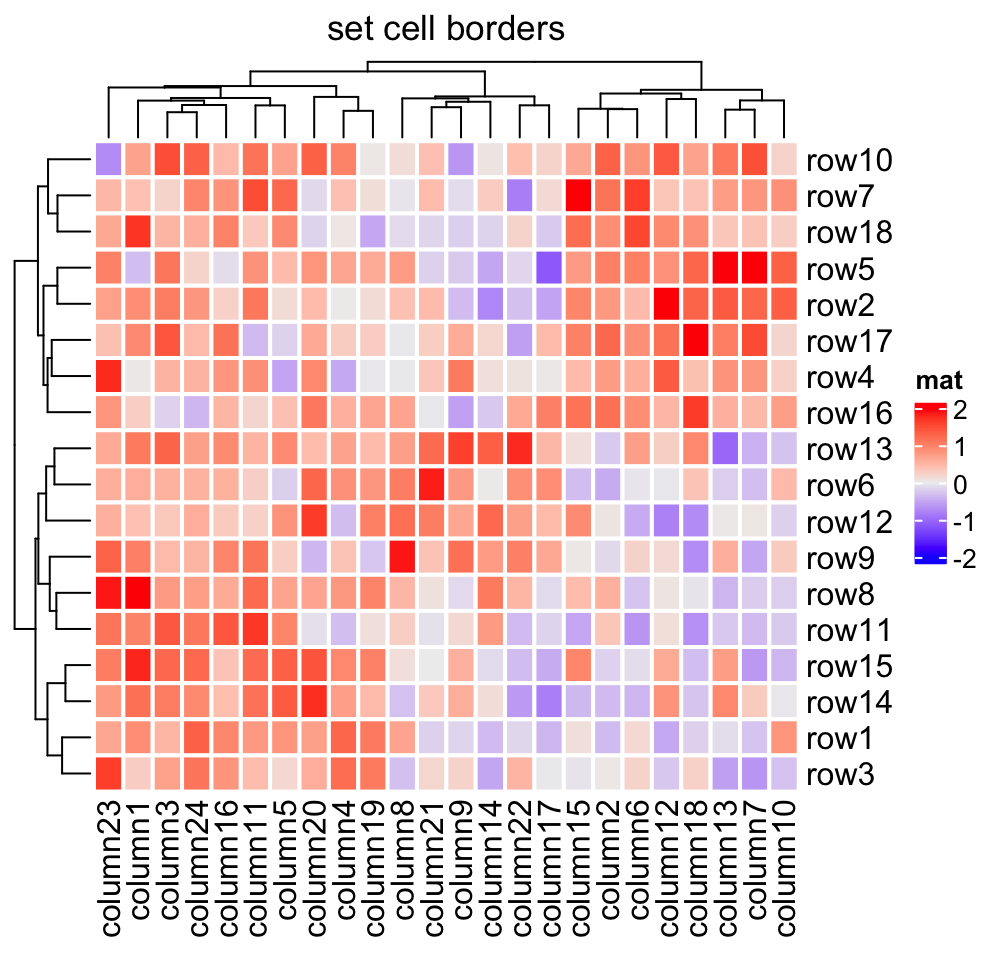Last but not the least, colors for the heatmap borders can be set by the border and rect_gp arguments. border controls the global border of the heatmap body and rect_gp controls the border of the grids in the heatmap.

The value of border can be logical (TRUE corresponds to black) or a character of color (e.g. red).

rect_gp is a gpar object which means you can only set it by grid::gpar(). Since the filled color is already controlled by the heatmap color mapping, you can only set the col parameter in gpar() to control the border of the heatmap grids.

Heatmap(mat, name = "mat", border = TRUE)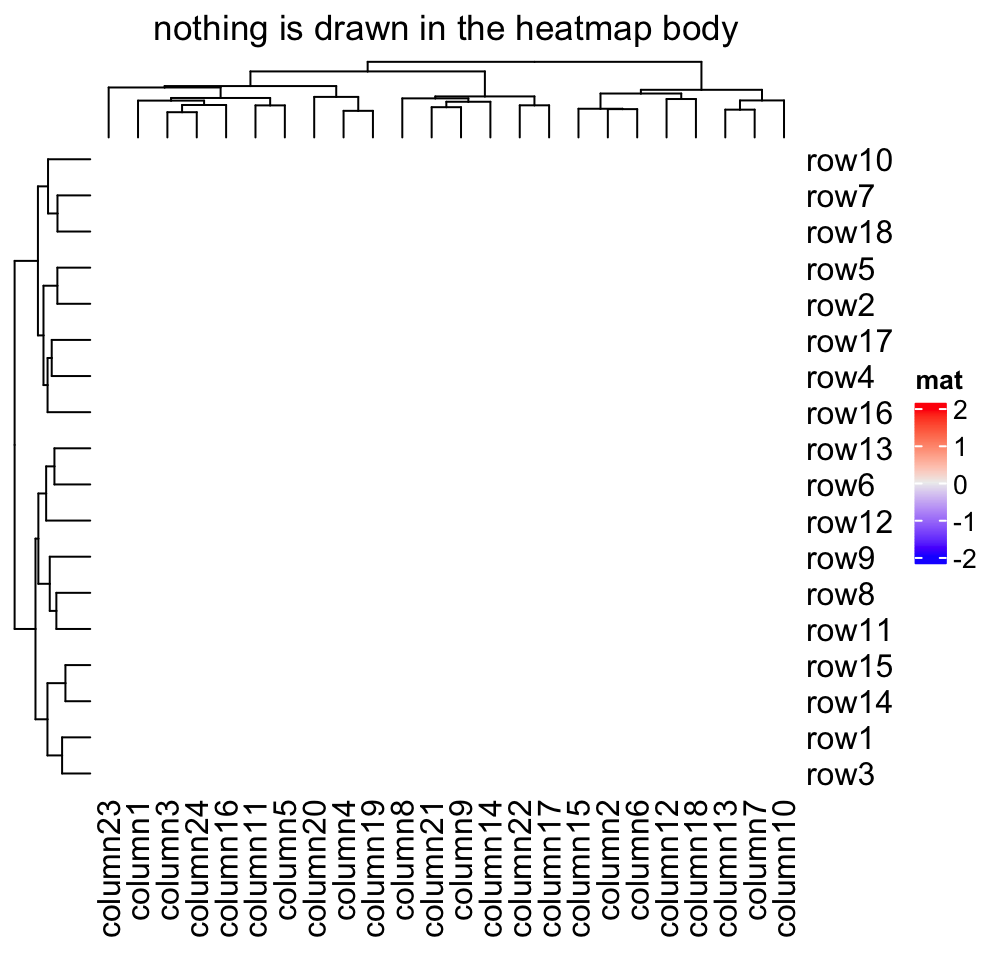Heatmap(mat, name = "mat", rect_gp = gpar(col = "white", lwd = 2))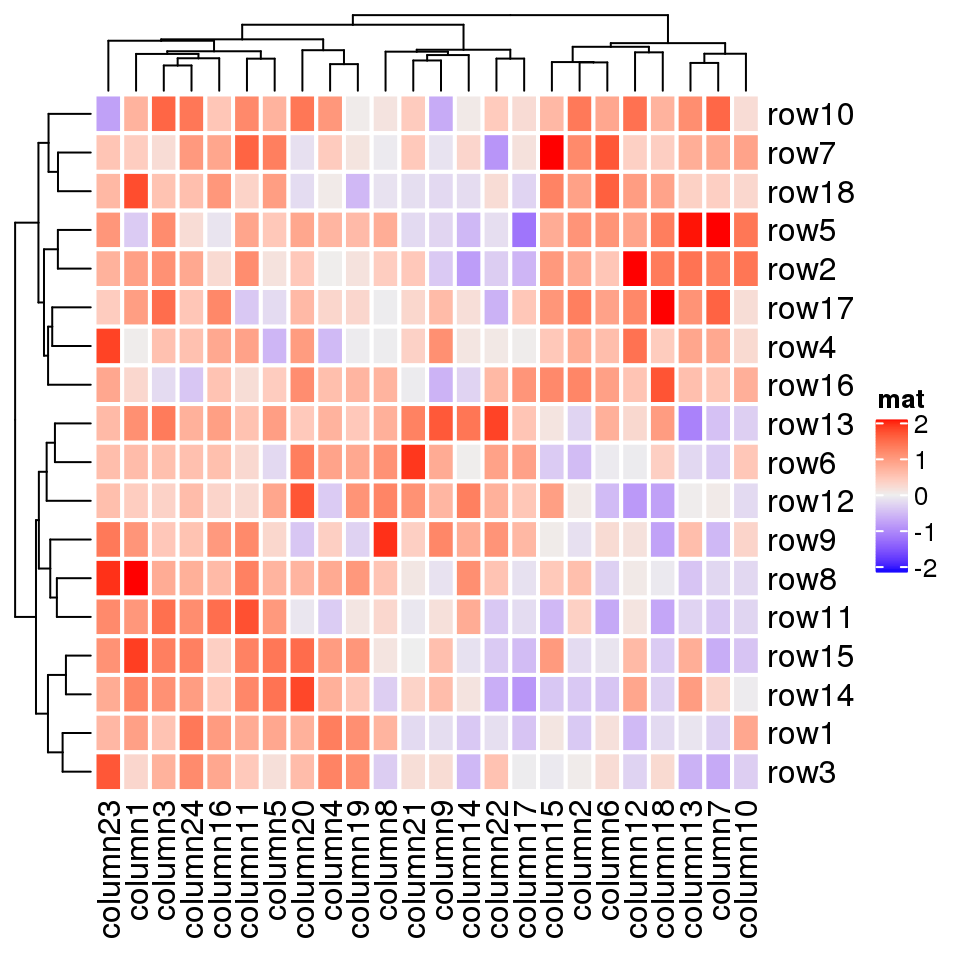If col is not set, the default color mapping by Heatmap() is designed with trying to be as convinient and meaningful as possible. Following are the rules for the default color mapping (by ComplexHeatmap:::default_col()):

• If the values are characters, the colors are generated by circlize::rand_color();
• If the values are from the heatmap annotation and are numeric, colors are mapped between white and one random color by linearly interpolating to the mininum and maxinum.
• If the values are from the matrix (let’s denote it as $$M$$) which corresponds to the heatmap body:
• If the fraction of positive values in $$M$$ is between 25% and 75%, colors are mapped to blue, white and red by linearly interpolating to $$-q$$, 0 and $$q$$, where $$q$$ is the maximum of $$|M|$$ if the number of unique values is less than 100, or $$q$$ is the 99^th percentile of $$|M|$$. This color mapping is centric to zero.
• Or else the colors are mapped to blue, white and red by linearly interpolating to $$q_1$$, $$(q_1 + q_2)/2$$ and $$q_2$$, where $$q_1$$ and $$q_2$$ are mininum and maxinum if the number of unique values is $$M$$ is less than 100, or $$q1$$ is the 1^th percentile and $$q2$$ is the 99^th percentile in $$M$$.

rect_gp allows a non-standard parameter type. If it is set to "none", the clustering is still applied but nothing in drawn on the heatmap body. The customized graphics on heatmap body can be added via a self-defined cell_fun or layer_fun (see Section 2.9).

Heatmap(mat, name = "mat", rect_gp = gpar(type = "none"))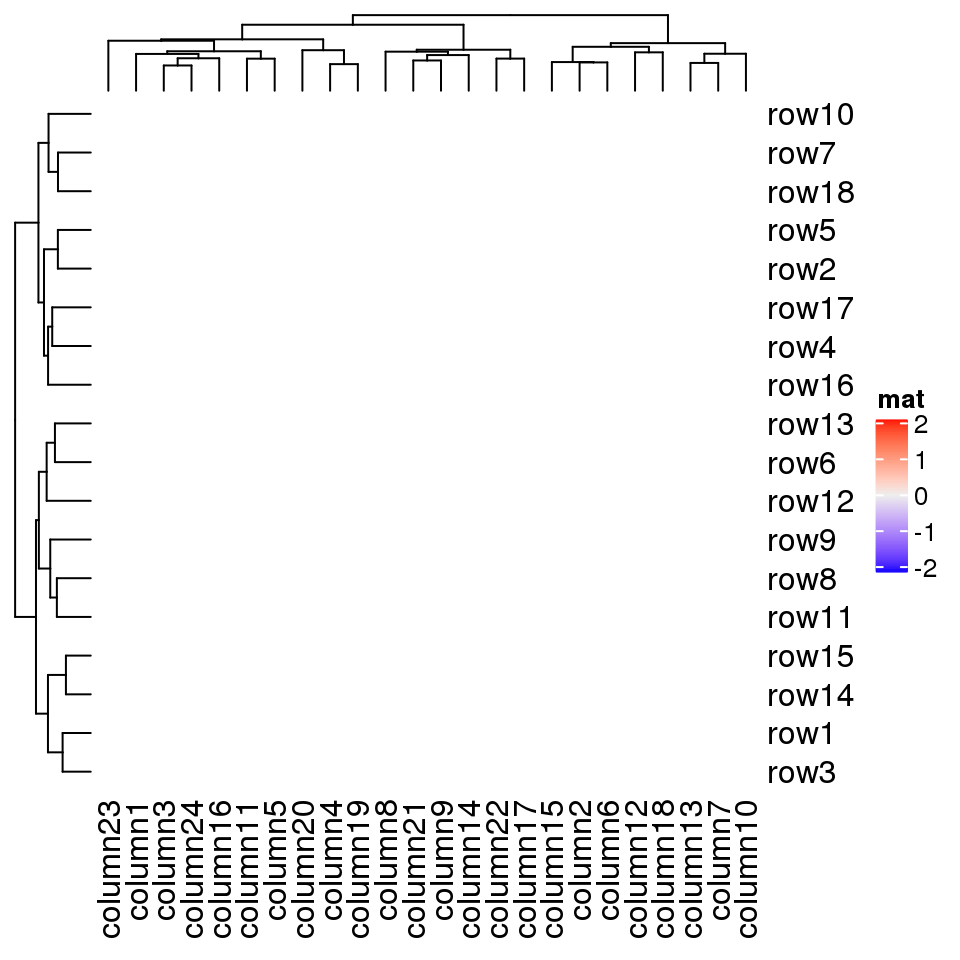## 2.2 Titles

The title of the heatmap basically tells what the plot is about. In ComplexHeatmap package, you can set heatmap title either by the row or/and by the column. Note at a same time you can only put e.g. column title either on the top or at the bottom of the heatmap.

The graphic parameters can be set by row_title_gp and column_title_gp respectively. Please remember you should use gpar() to specify graphic parameters.

Heatmap(mat, name = "mat", column_title = "I am a column title",
row_title = "I am a row title")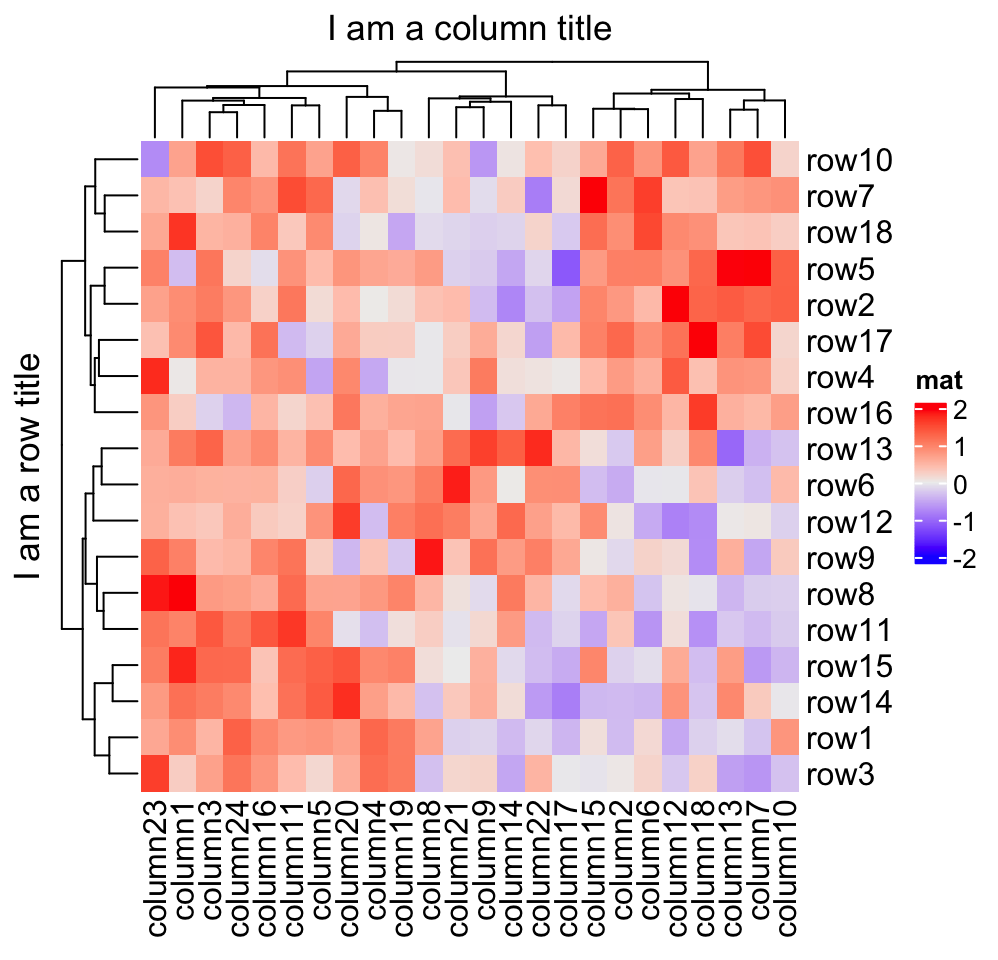Heatmap(mat, name = "mat", column_title = "I am a column title at the bottom",
column_title_side = "bottom")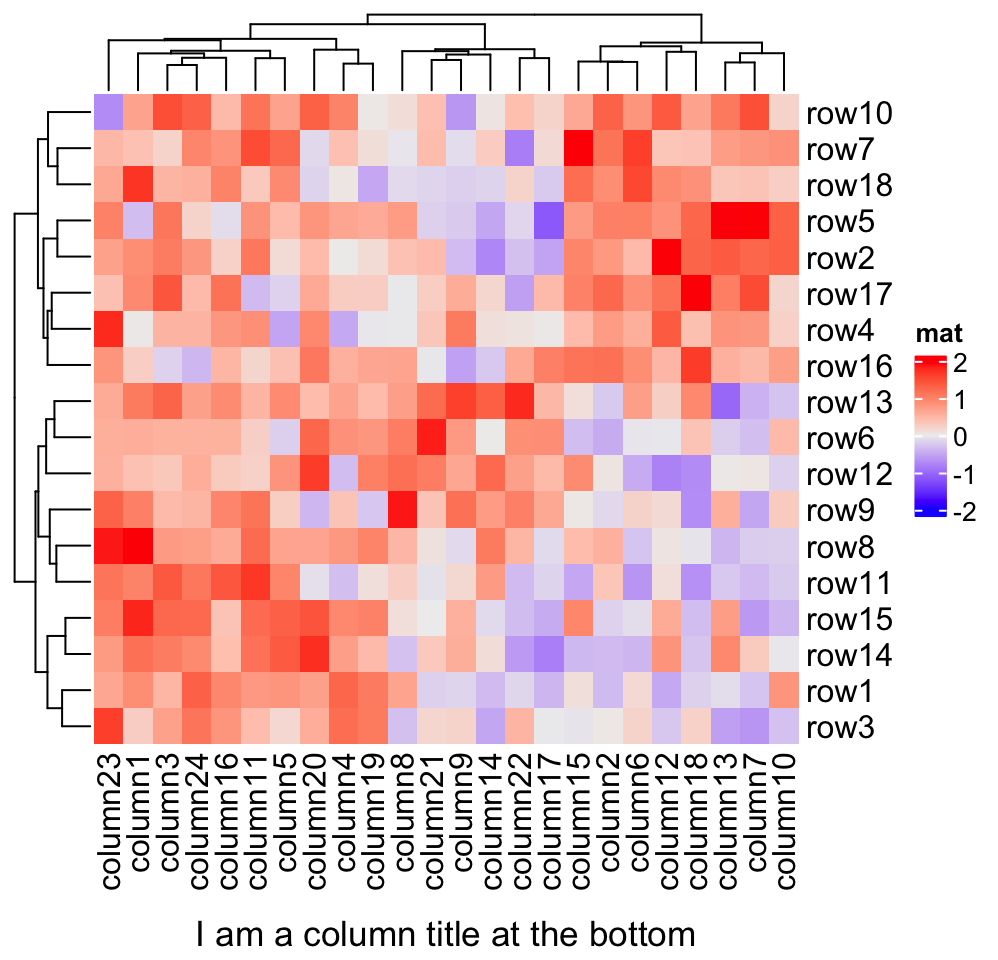Heatmap(mat, name = "mat", column_title = "I am a big column title",
column_title_gp = gpar(fontsize = 20, fontface = "bold"))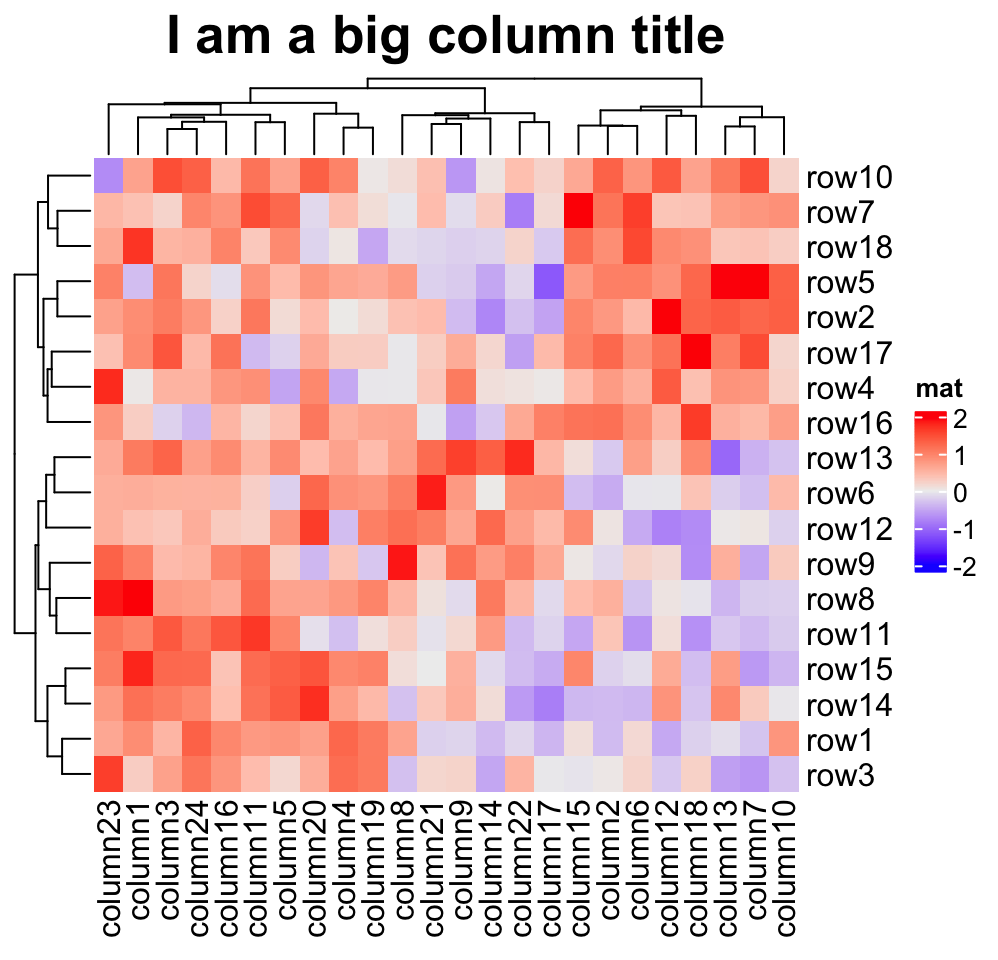Rotations for titles can be set by row_title_rot and column_title_rot, but only horizontal and vertical rotations are allowed.

Heatmap(mat, name = "mat", row_title = "row title", row_title_rot = 0)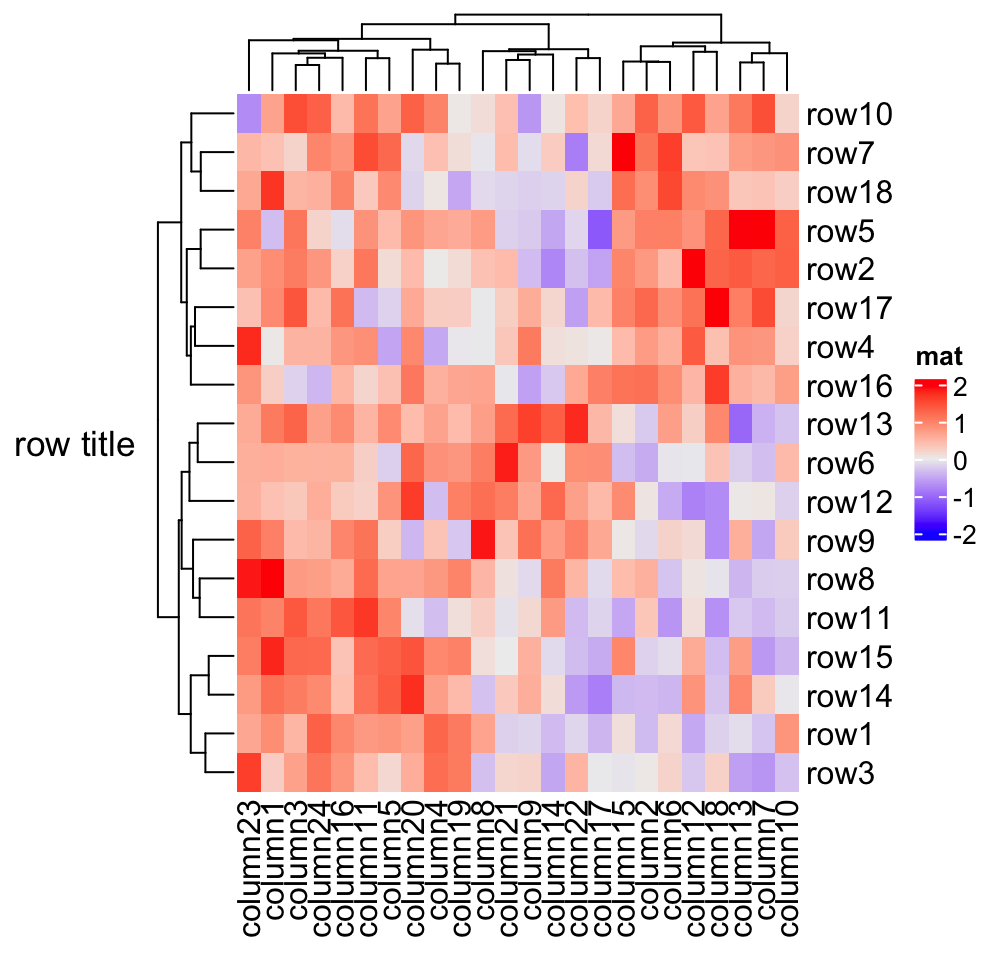Row or column title supports as a template which is used when rows or columns are split in the heatmap (because there will be multiple row/column titles). This functionality is introduced in Section 2.7. A quick example would be:

# code only for demonstration
# row title would be cluster_1 and cluster_2
Heatmap(mat, name = "mat", row_km = 2, row_title = "cluster_%s")

You can set fill parameter in row_title_gp and column_title_gp to set the background color of titles. Since col in e.g. row_title_gp controls the color of text, border is used to control the color of the background border.

Heatmap(mat, name = "mat", column_title = "I am a column title",
column_title_gp = gpar(fill = "red", col = "white", border = "red"))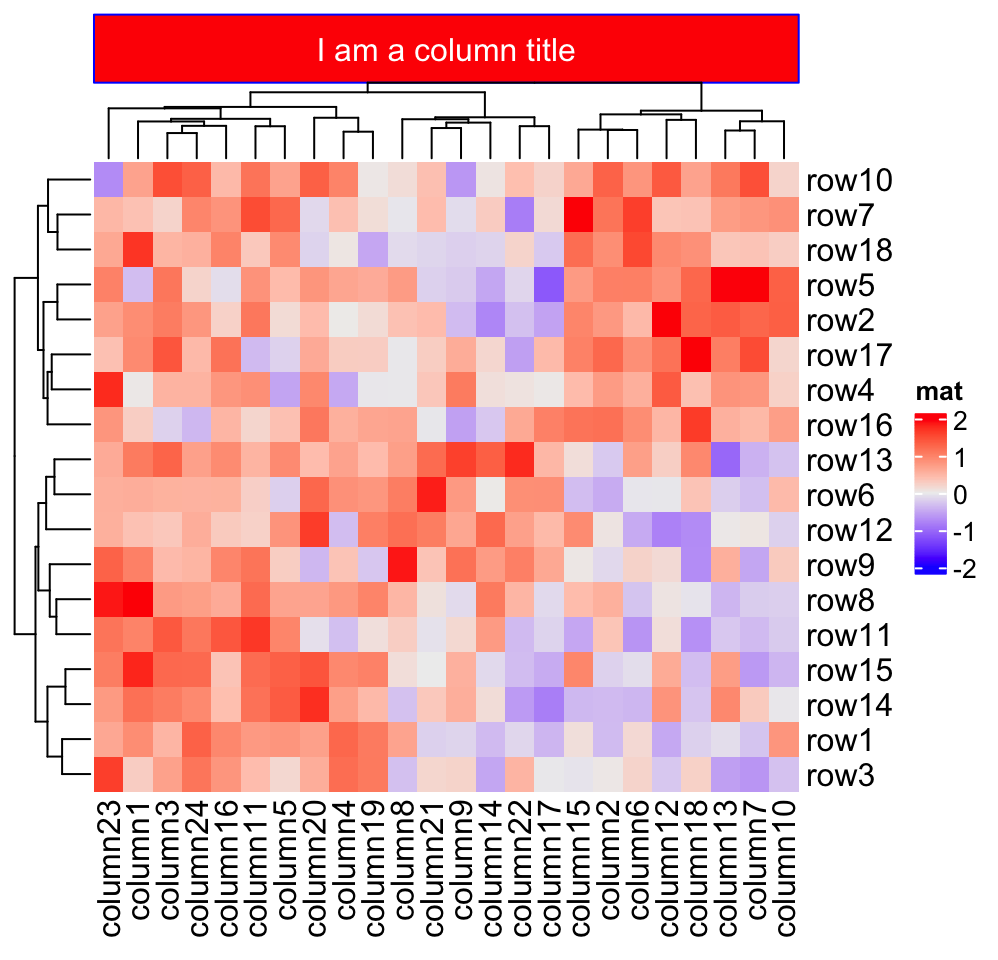If the graphic elements are texts, they can be set as mathematical formulas.

Heatmap(mat, name = "mat",
column_title = expression(hat(beta) == (X^t * X)^{-1} * X^t * y))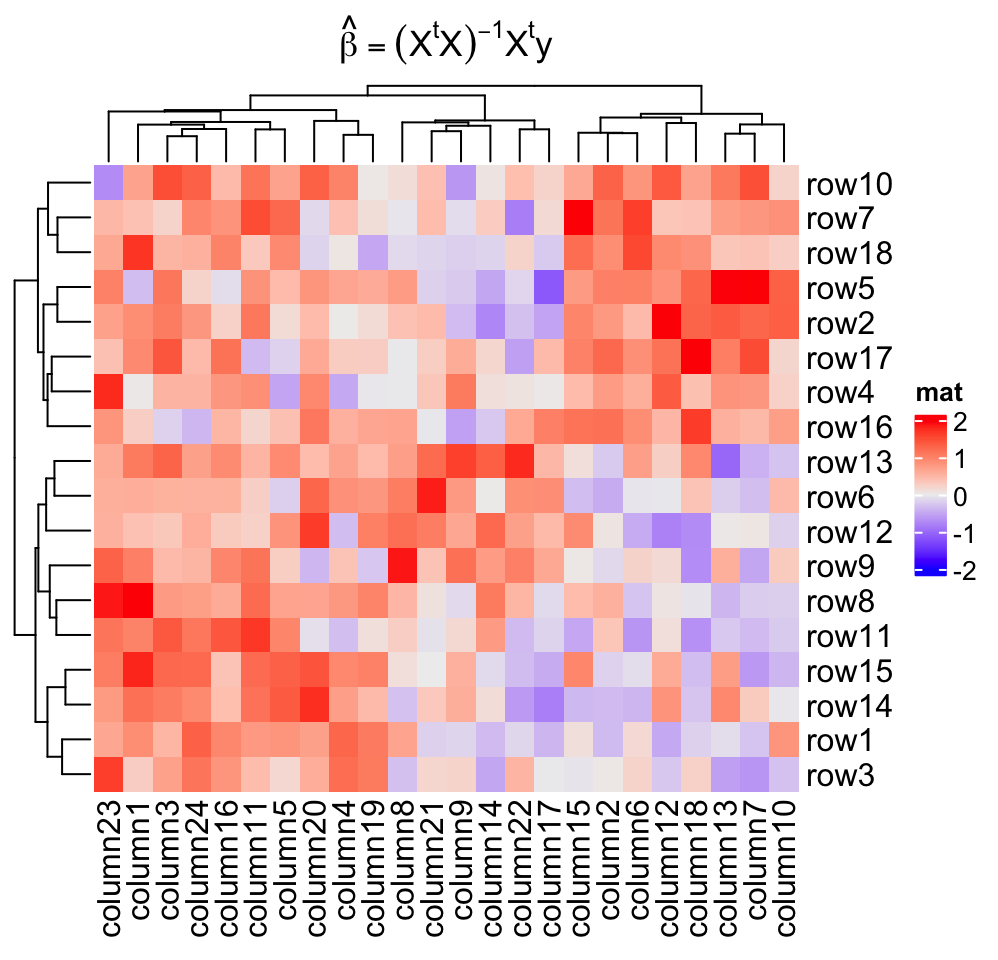## 2.3 Clustering

Clustering might be the key component of the heatmap visualization. In ComplexHeatmap package, hierarchical clustering is supported with great flexibility. You can specify the clustering either by:

• a pre-defined distance method (e.g. "euclidean" or "pearson"),
• a distance function,
• a object that already contains clustering (a hclust or dendrogram object or object that can be coerced to dendrogram class),
• a clustering function.

It is also possible to render the dendrograms with different colors and styles for different branches for better revealing structures of the dendrogram (e.g. by dendextend::color_branches()).

First, there are general settings for the clustering, e.g. whether apply clustering or show dendrograms, the side of the dendrograms and heights of the dendrograms.

Heatmap(mat, name = "mat", cluster_rows = FALSE) # turn off row clustering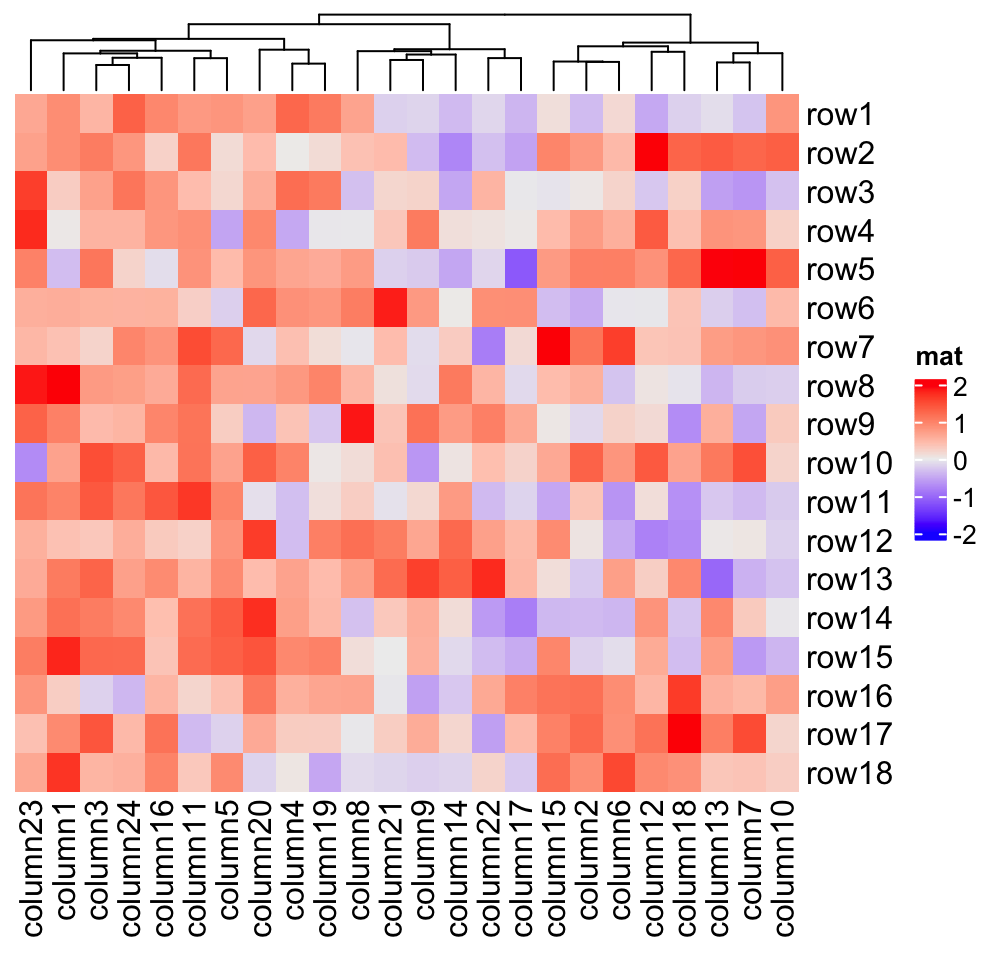Heatmap(mat, name = "mat", show_column_dend = FALSE) # hide column dendrogram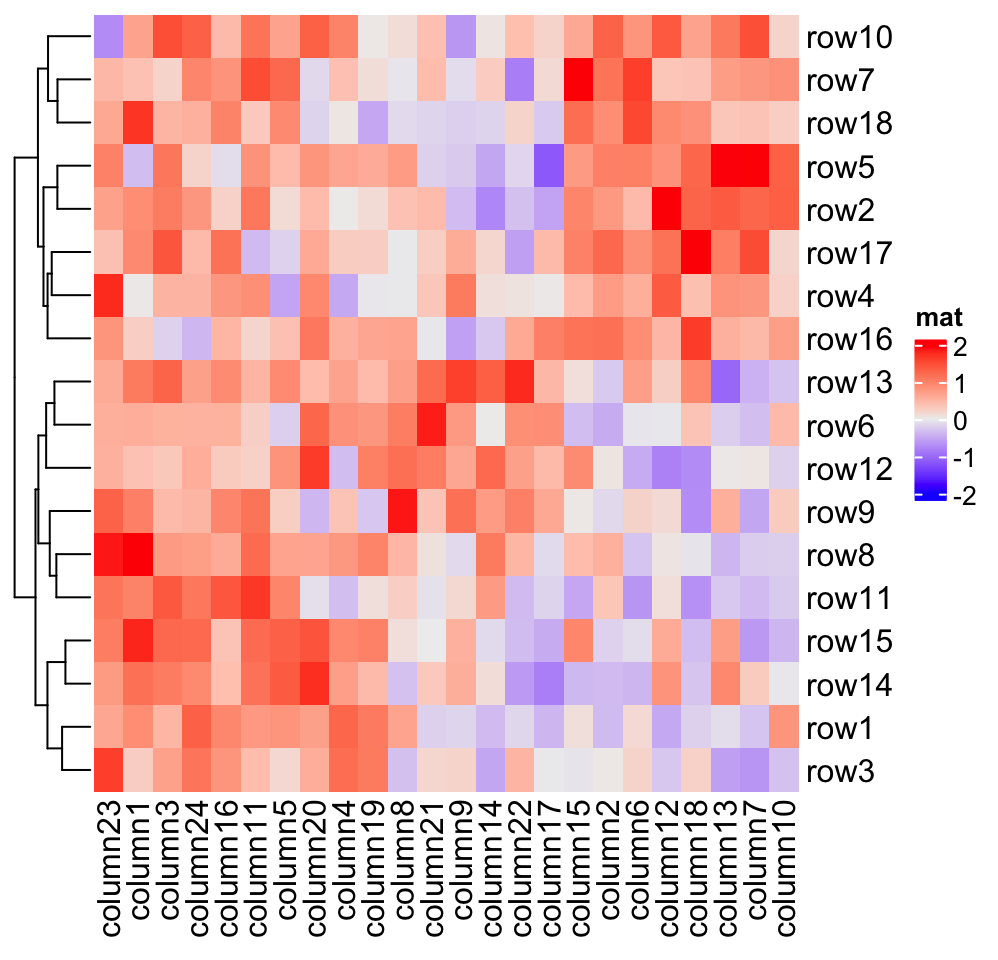Heatmap(mat, name = "mat", row_dend_side = "right", column_dend_side = "bottom")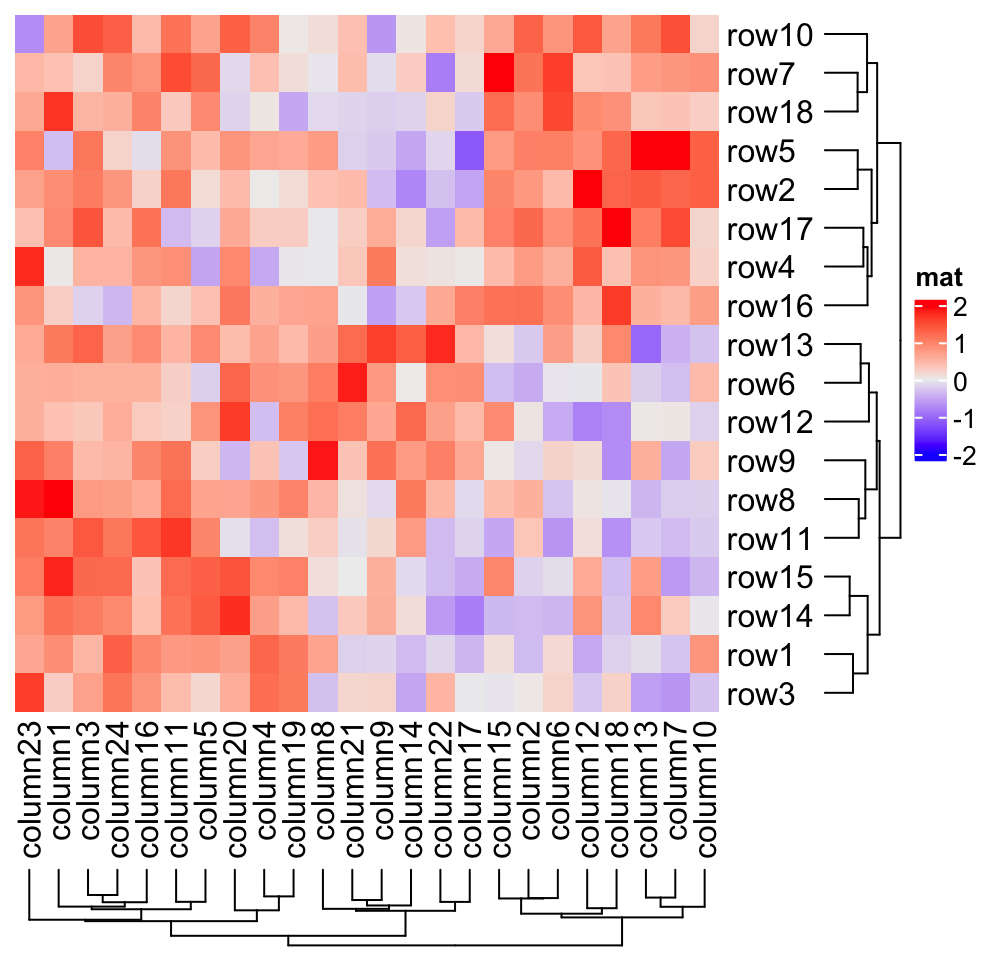Heatmap(mat, name = "mat", column_dend_height = unit(4, "cm"),
row_dend_width = unit(4, "cm"))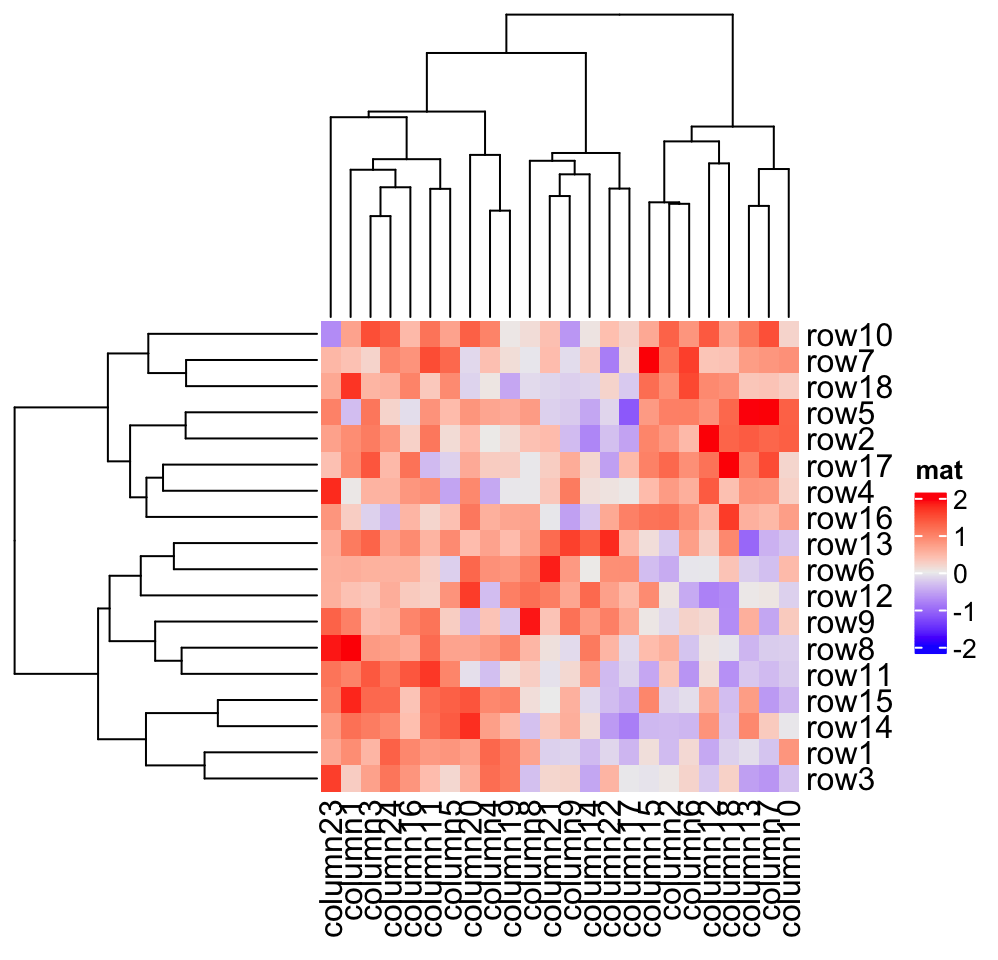### 2.3.1 Distance methods

Hierarchical clustering is performed in two steps: calculate the distance matrix and apply clustering. There are three ways to specify distance metric for clustering:

• specify distance as a pre-defined option. The valid values are the supported methods in dist() function and in "pearson", "spearman" and "kendall". The correlation distance is defined as 1 - cor(x, y, method). All these built-in distance methods allow NA values.
• a self-defined function which calculates distance from a matrix. The function should only contain one argument. Please note for clustering on columns, the matrix will be transposed automatically.
• a self-defined function which calculates distance from two vectors. The function should only contain two arguments. Note this might be slow because it is implemented by two nested for loop.
Heatmap(mat, name = "mat", clustering_distance_rows = "pearson",
column_title = "pre-defined distance method (1 - pearson)")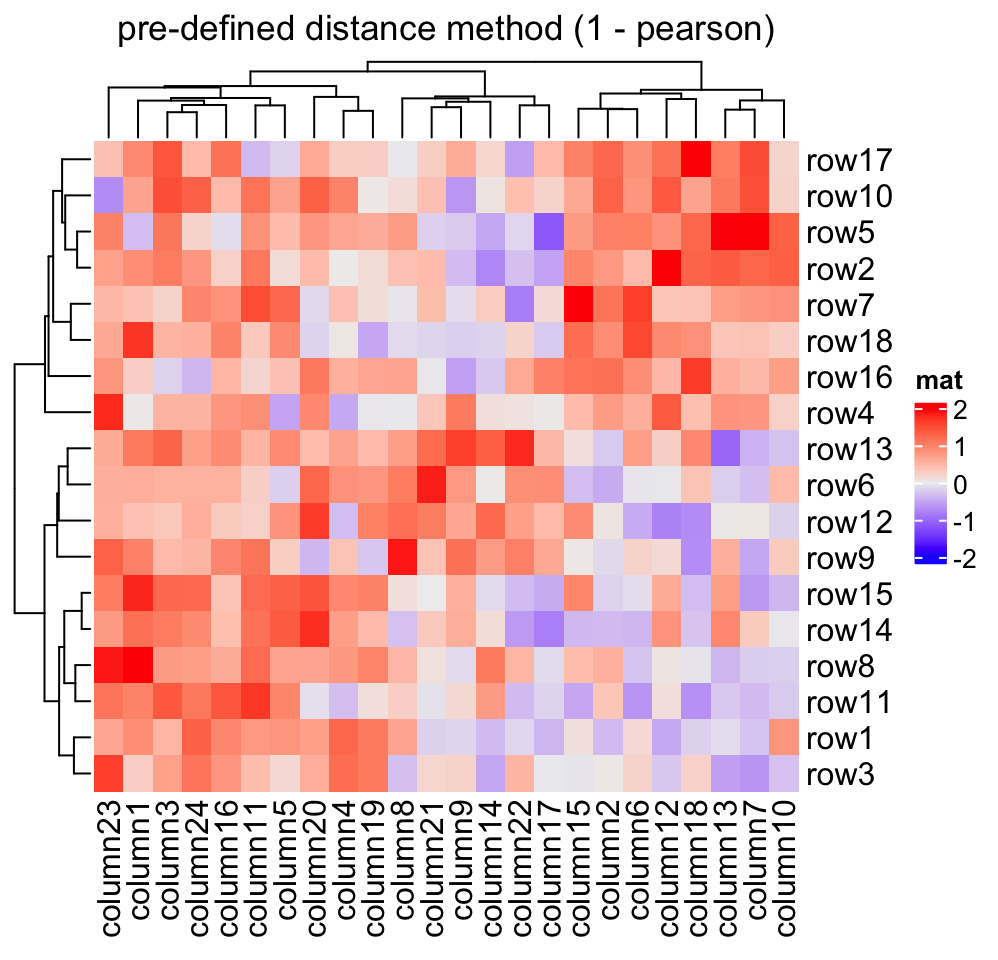Heatmap(mat, name = "mat", clustering_distance_rows = function(m) dist(m),
column_title = "a function that calculates distance matrix")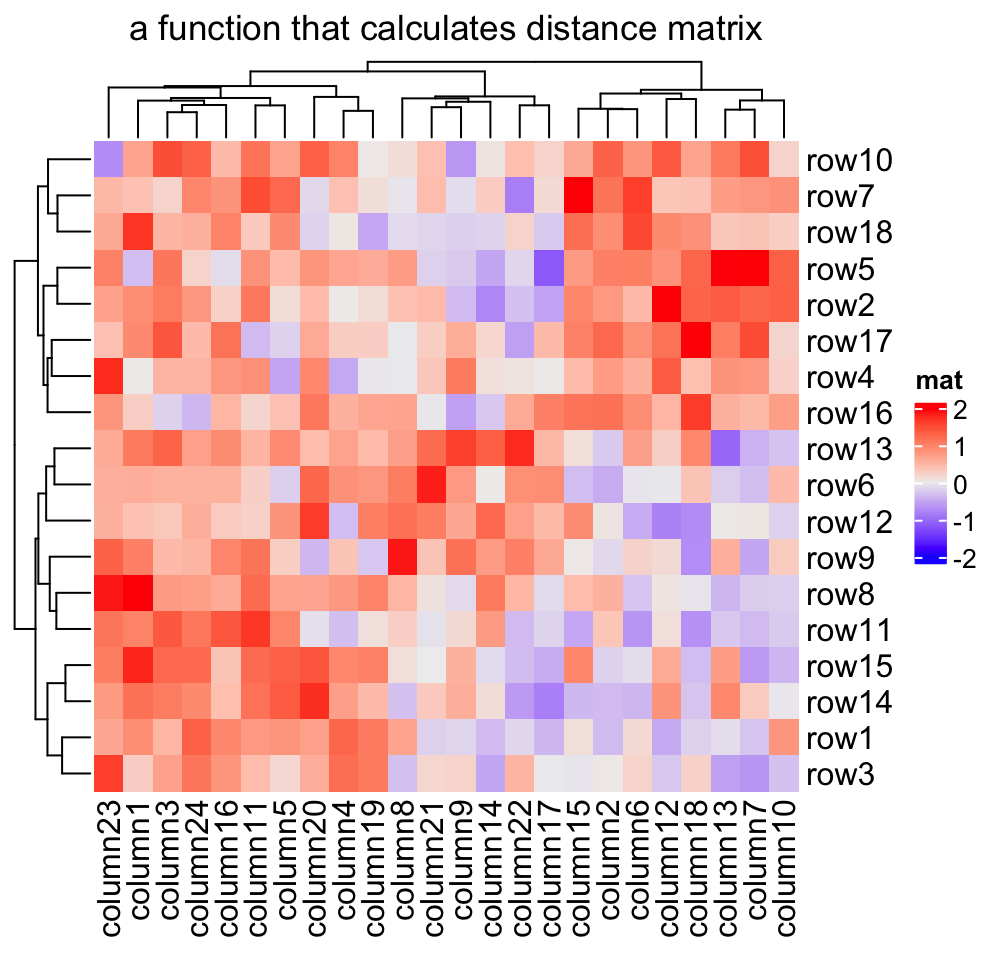Heatmap(mat, name = "mat", clustering_distance_rows = function(x, y) 1 - cor(x, y),
column_title = "a function that calculates pairwise distance")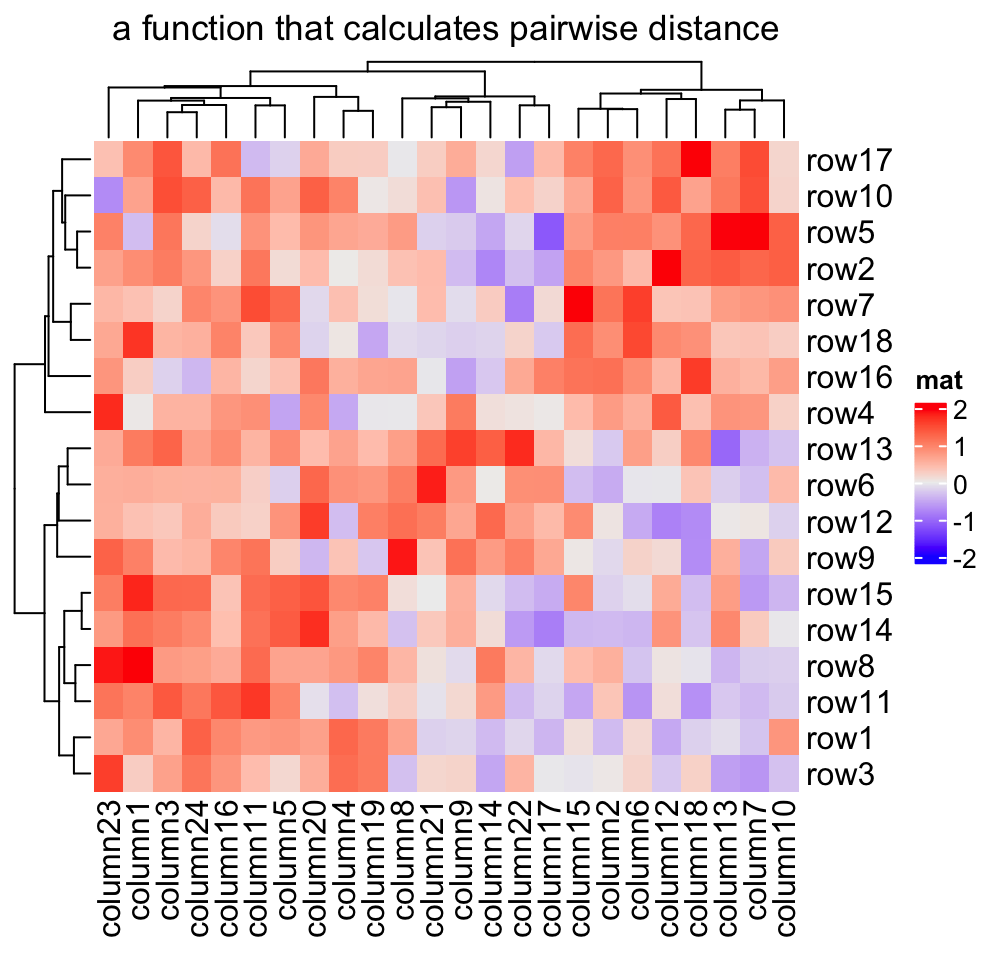Based on these features, we can apply clustering which is robust to outliers based on the pairwise distance. Note here we set the color mapping function because we don’t want outliers affect the colors.

mat_with_outliers = mat
for(i in  1:10) mat_with_outliers[i, i] = 1000
robust_dist = function(x, y) {
qx = quantile(x, c(0.1, 0.9))
qy = quantile(y, c(0.1, 0.9))
l = x > qx & x < qx & y > qy & y < qy
x = x[l]
y = y[l]
sqrt(sum((x - y)^2))
}

We can compare the two heatmaps with or without the robust distance method:

Heatmap(mat_with_outliers, name = "mat",
col = colorRamp2(c(-2, 0, 2), c("green", "white", "red")),
column_title = "dist")
Heatmap(mat_with_outliers, name = "mat",
col = colorRamp2(c(-2, 0, 2), c("green", "white", "red")),
clustering_distance_rows = robust_dist,
clustering_distance_columns = robust_dist,
column_title = "robust_dist")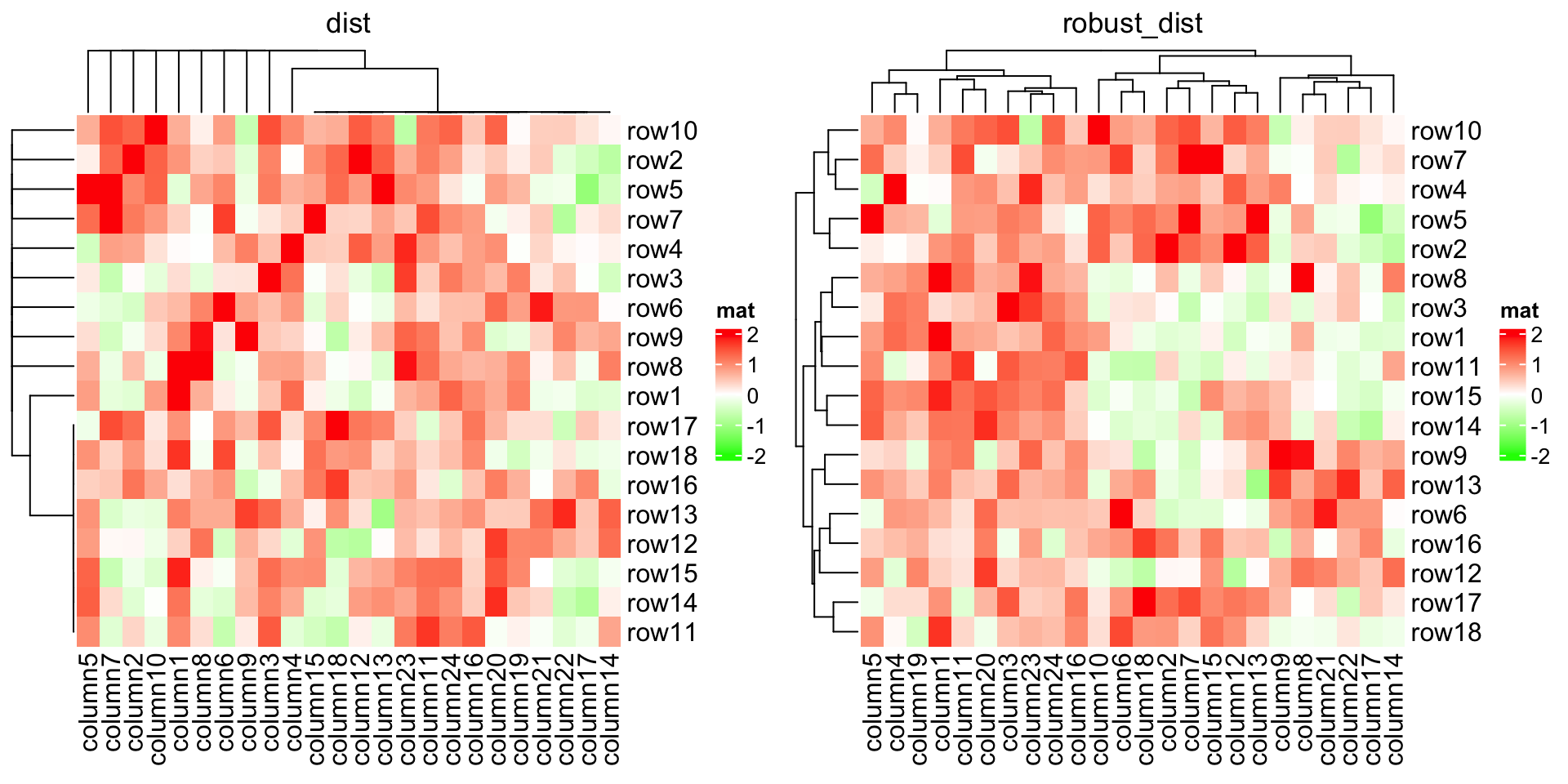If there are proper distance methods (like methods in stringdist package), you can also cluster a character matrix. cell_fun argument will be introduced in Section 2.9.

mat_letters = matrix(sample(letters[1:4], 100, replace = TRUE), 10)
# distance in the ASCII table
dist_letters = function(x, y) {
x = strtoi(charToRaw(paste(x, collapse = "")), base = 16)
y = strtoi(charToRaw(paste(y, collapse = "")), base = 16)
sqrt(sum((x - y)^2))
}
Heatmap(mat_letters, name = "letters", col = structure(2:5, names = letters[1:4]),
clustering_distance_rows = dist_letters, clustering_distance_columns = dist_letters,
cell_fun = function(j, i, x, y, w, h, col) { # add text to each grid
grid.text(mat_letters[i, j], x, y)
})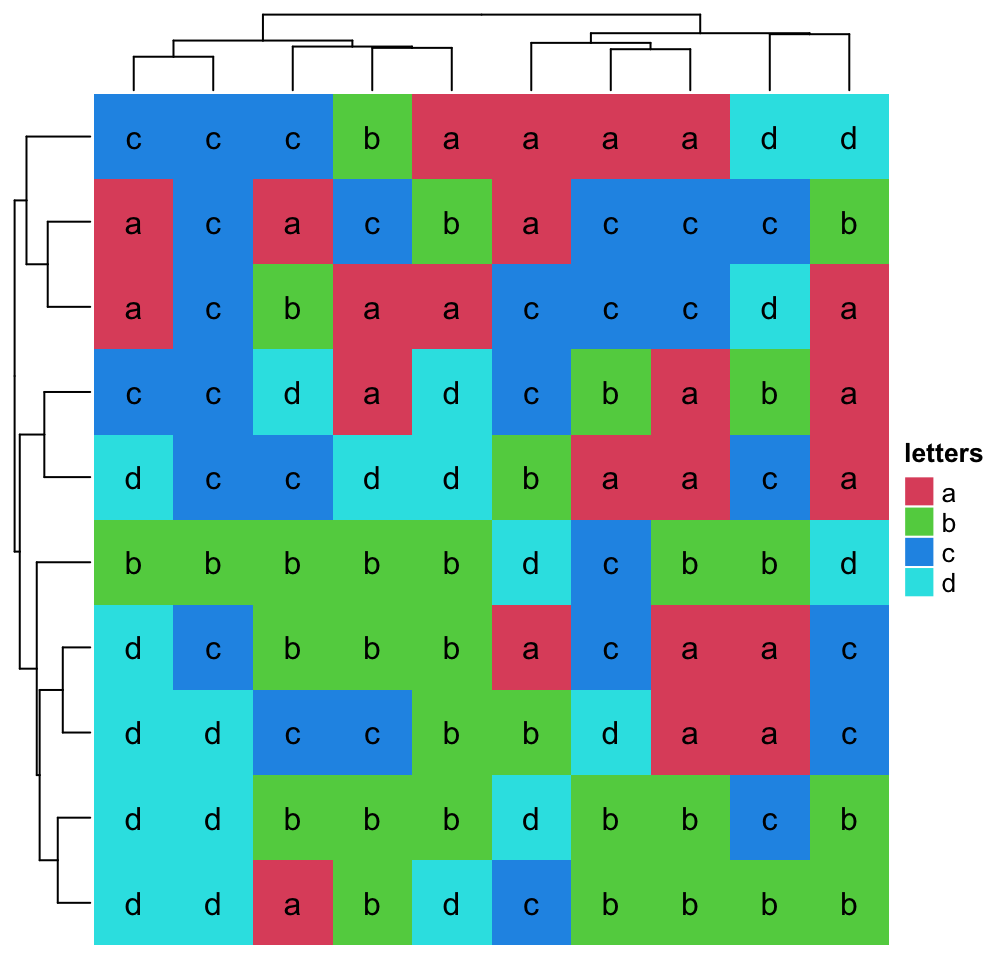### 2.3.2 Clustering methods

Method to perform hierarchical clustering can be specified by clustering_method_rows and clustering_method_columns. Possible methods are those supported in hclust() function.

Heatmap(mat, name = "mat", clustering_method_rows = "single")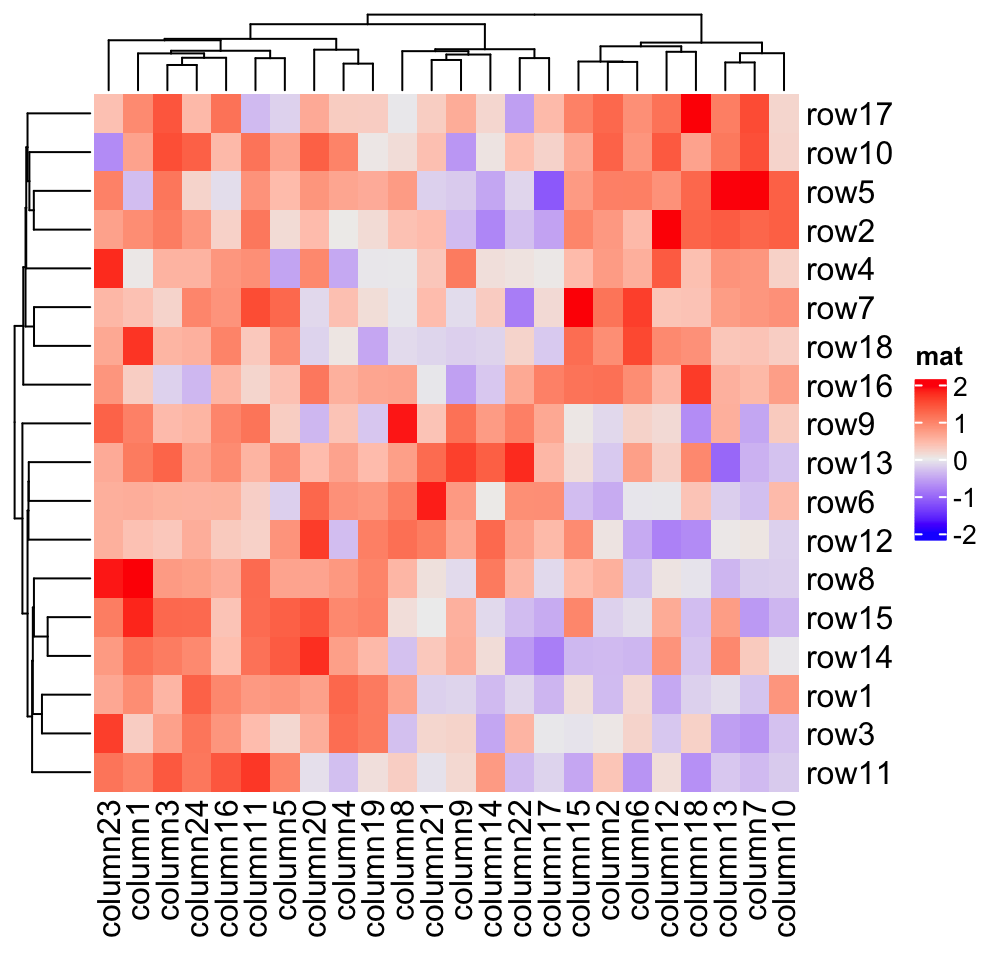If you already have a clustering object or a function which directly returns a clustering object, you can ignore the distance settings and set cluster_rows or cluster_columns to the clustering objects or clustering functions. If it is a clustering function, the only argument should be the matrix and it should return a hclust or dendrogram object or a object that can be coerced to the dendrogram class.

In following example, we perform clustering with methods from cluster package either by a pre-calculated clustering object or a clustering function:

library(cluster)
Heatmap(mat, name = "mat", cluster_rows = diana(mat),
cluster_columns = agnes(t(mat)), column_title = "clustering objects")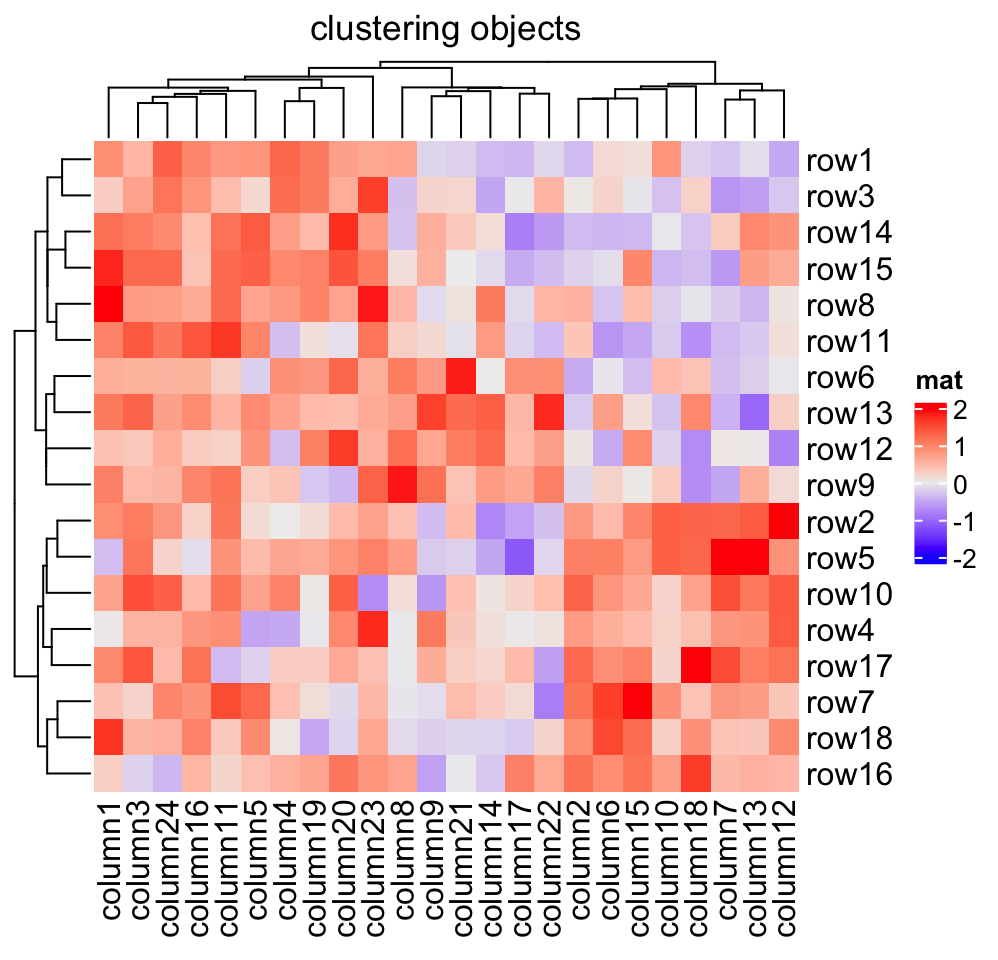# if cluster_columns is set as a function, you don't need to transpose the matrix
Heatmap(mat, name = "mat", cluster_rows = diana,
cluster_columns = agnes, column_title = "clustering functions")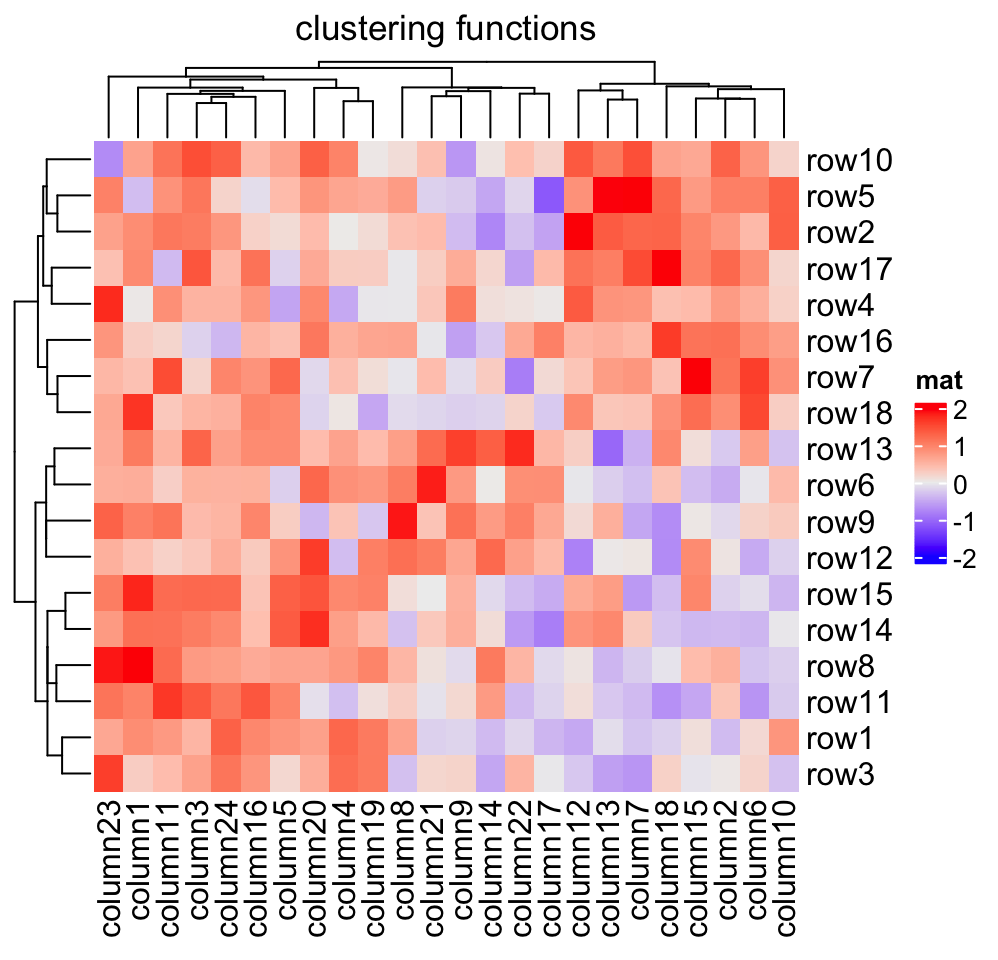The last command is as same as :

# code only for demonstration
Heatmap(mat, name = "mat", cluster_rows = function(m) as.dendrogram(diana(m)),
cluster_columns = function(m) as.dendrogram(agnes(m)),
column_title = "clutering functions")

Please note, when cluster_rows is set as a function, the argument m is the input mat itself, while for cluster_columns, m is the transpose of mat.

fastcluster::hclust implements a faster version of hclust(). You can set it to cluster_rows and cluster_columns to use the faster version of hclust().

# code only for demonstration
fh = function(x) fastcluster::hclust(dist(x))
Heatmap(mat, name = "mat", cluster_rows = fh, cluster_columns = fh)

To make it more convinient to use the faster version of hclust() (assuming you have many heatmaps to construct), it can be set as a global option. The usage of ht_opt is introduced in Section 4.13.

# code only for demonstration
ht_opt$fast_hclust = TRUE # now fastcluster::hclust is used in all heatmaps This is one specific scenario that you might already have a subgroup classification for the matrix rows or columns, and you only want to perform clustering for the features in the same subgroup. There is one way that you can split the heatmap by the subgroup variable (see Section 2.7), or you can use cluster_within_group() clustering function to generate a special dendrogram. group = kmeans(t(mat), centers = 3)$cluster
Heatmap(mat, name = "mat", cluster_columns = cluster_within_group(mat, group))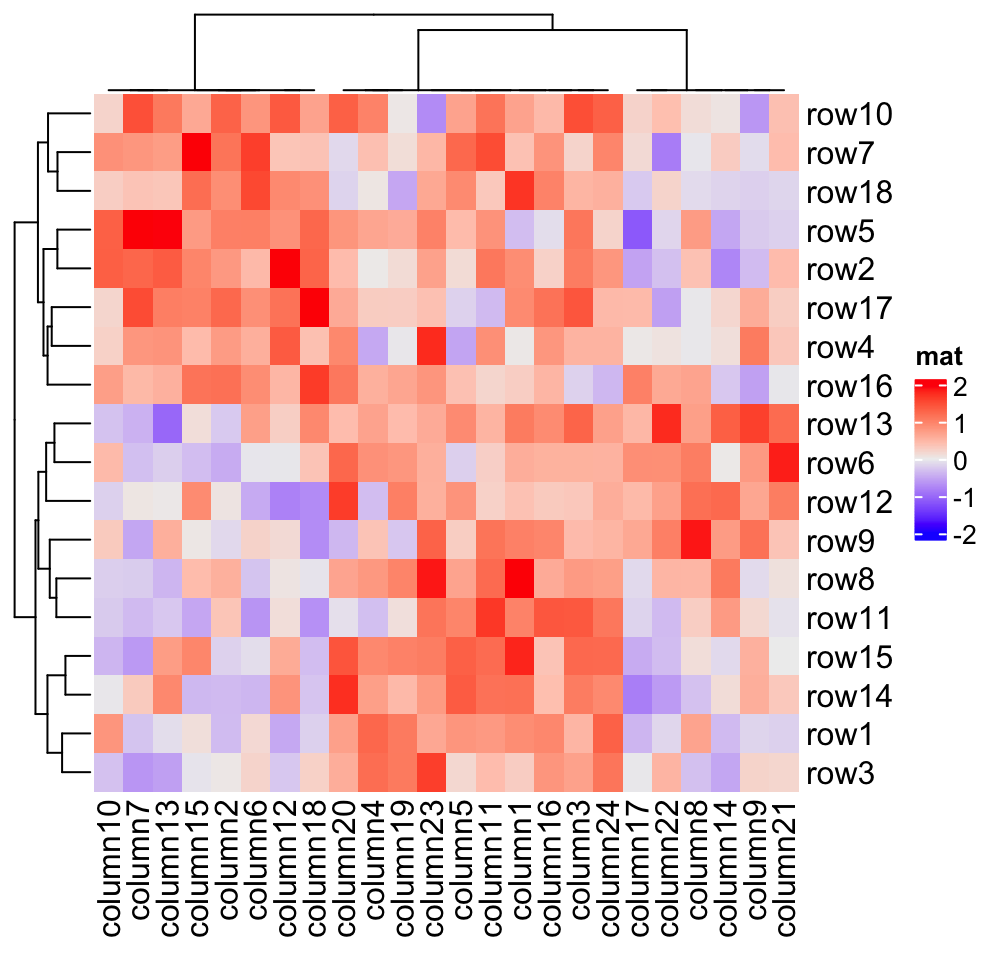In above example, columns in a same group are still clustered, but the dendrogram is degenerated as a flat line. The dendrogram on columns shows the hierarchy of the groups.

### 2.3.3 Render dendrograms

If you want to render the dendrogram, normally you need to generate a dendrogram object and render it in the first place, then send it to the cluster_rows or cluster_columns argument.

You can render your dendrogram object by the dendextend package to make a more customized visualization of the dendrogram. Note ComplexHeatmap only allows rendering on the dendrogram lines.

library(dendextend)
row_dend = as.dendrogram(hclust(dist(mat)))
row_dend = color_branches(row_dend, k = 2) # color_branches() returns a dendrogram object
Heatmap(mat, name = "mat", cluster_rows = row_dend)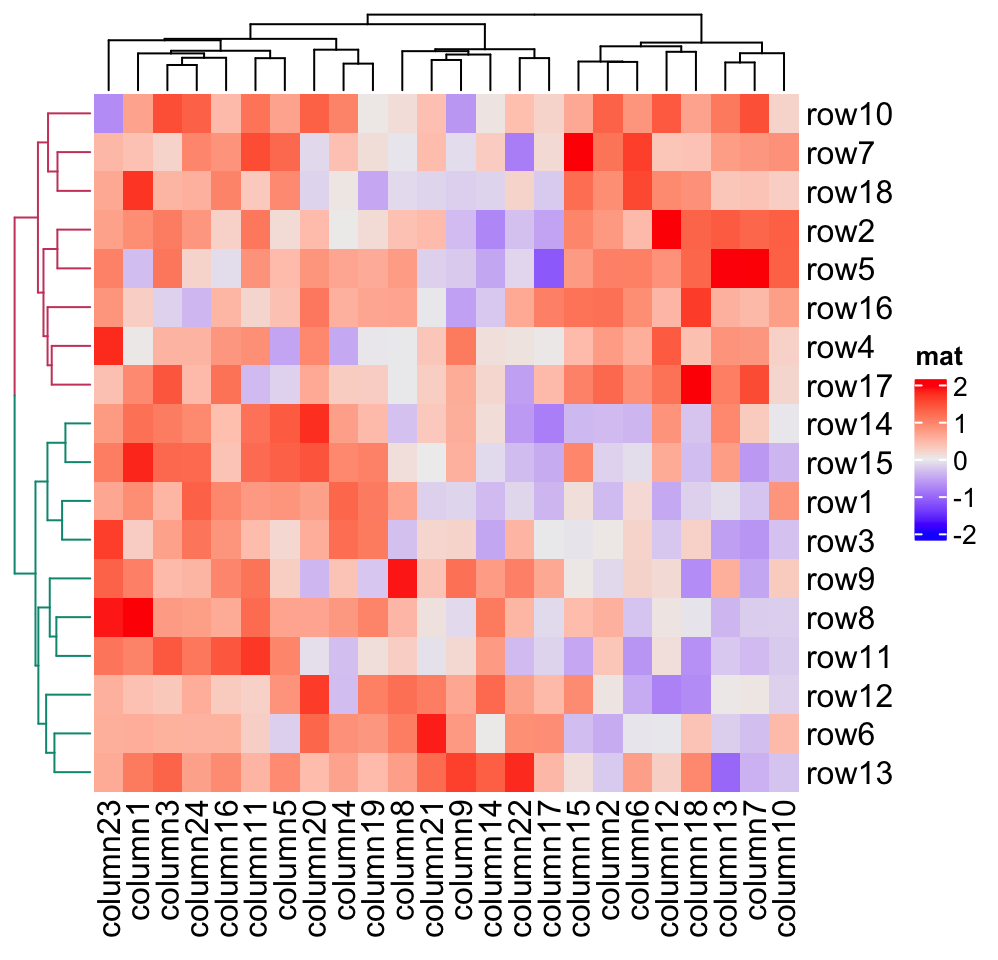row_dend_gp and column_dend_gp control the global graphic setting for dendrograms. Note e.g. graphic settings in row_dend will be overwritten by row_dend_gp.

Heatmap(mat, name = "mat", cluster_rows = row_dend, row_dend_gp = gpar(col = "red"))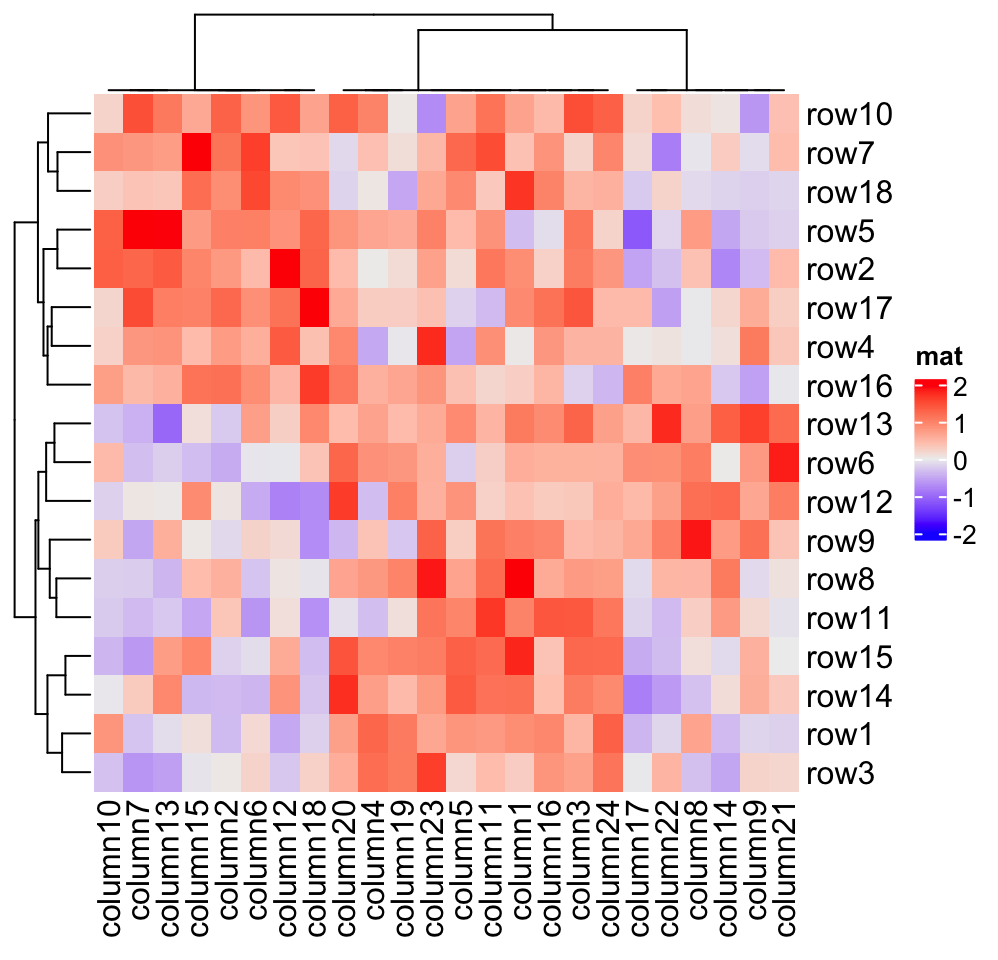### 2.3.4 Reorder dendrograms

In the Heatmap() function, dendrograms are reordered to make features with larger difference more separated from each others (please refer to the documentation of reorder.dendrogram()). Here the difference (or it is called the weight) is measured by the row means if it is a row dendrogram or by the column means if it is a column dendrogram. row_dend_reorder and column_dend_reorder control whether to apply dendrogram reordering if the value is set as logical. The two arguments also control the weight for the reordering if they are set to numeric vectors (it will be sent to the wts argument of reorder.dendrogram()). The reordering can be turned off by setting e.g. row_dend_reorder = FALSE.

By default, dendrogram reordering is turned on if cluster_rows/cluster_columns is set as logical value or a clustering function. It is turned off if cluster_rows/cluster_columns is set as clustering object.

Compare following two heatmaps:

m2 = matrix(1:100, nr = 10, byrow = TRUE)
Heatmap(m2, name = "mat", row_dend_reorder = FALSE, column_title = "no reordering")
Heatmap(m2, name = "mat", row_dend_reorder = TRUE, column_title = "apply reordering")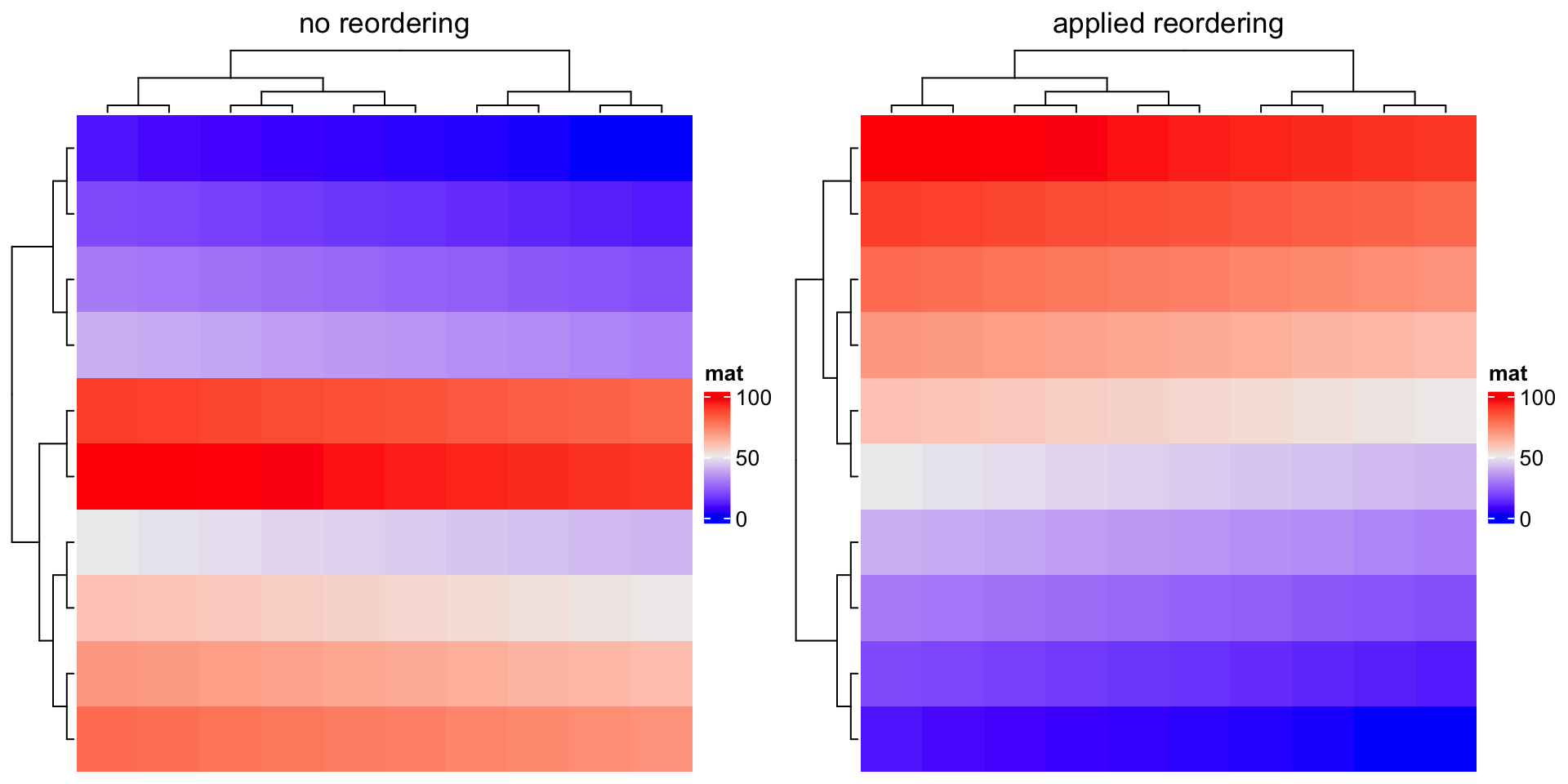There are many other methods for reordering dendrograms, e.g. the dendsort package. Basically, all these methods still return a dendrogram that has been reordered, thus, we can firstly generate the row or column dendrogram based on the data matrix, reorder it by some method, and assign it back to cluster_rows or cluster_columns.

Compare following two reorderings. Can you tell which is better?

Heatmap(mat, name = "mat", column_title = "default reordering")

library(dendsort)
dend = dendsort(hclust(dist(mat)))
Heatmap(mat, name = "mat", cluster_rows = dend, column_title = "reorder by dendsort")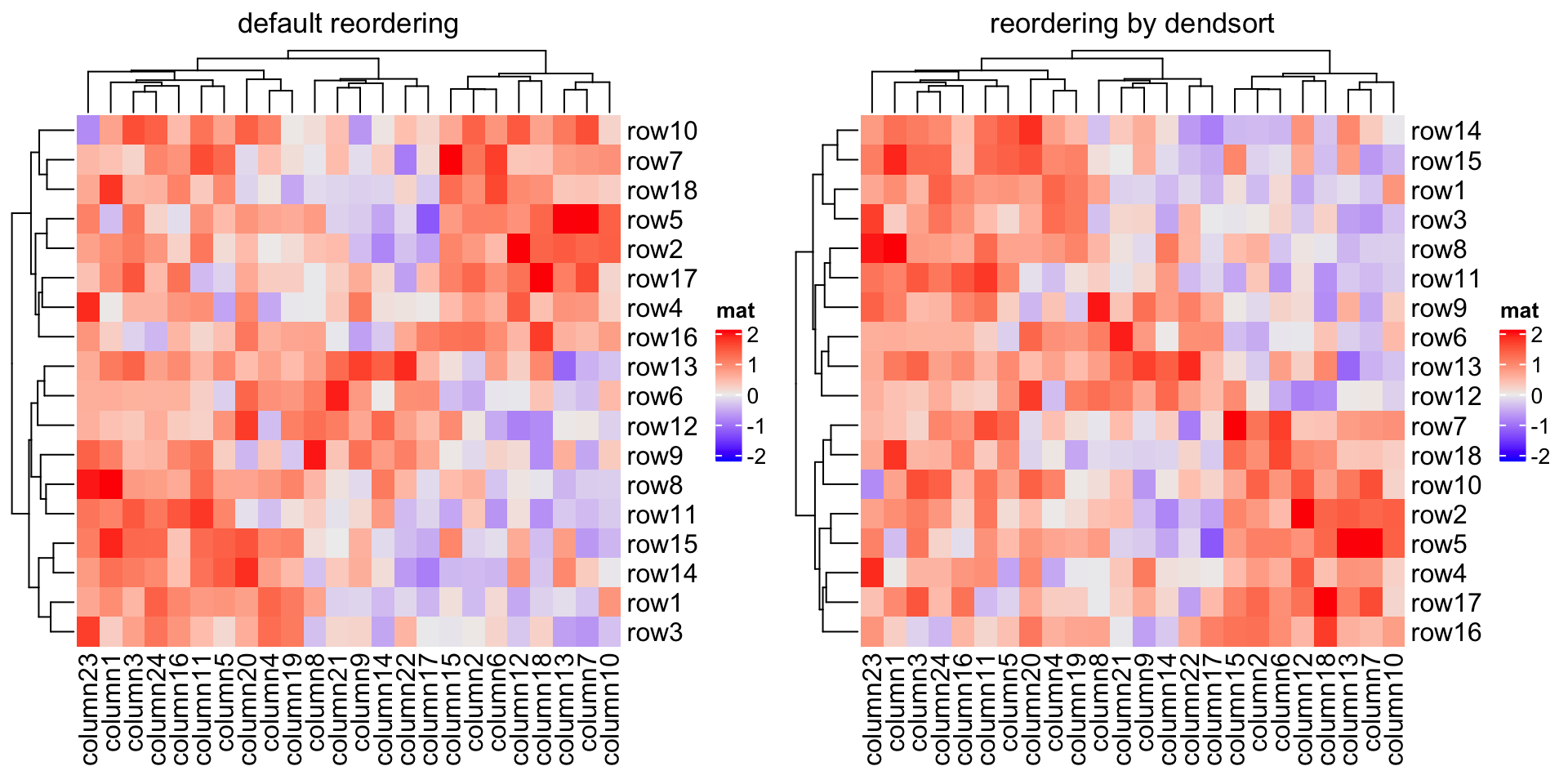## 2.4 Set row and column orders

Clustering is used to adjust row orders and column orders of the heatmap, but you can still set the order manually by row_order and column_order. If e.g. row_order is set, row clustering is turned off by default.

Heatmap(mat, name = "mat", row_order = order(as.numeric(gsub("row", "", rownames(mat)))),
column_order = order(as.numeric(gsub("column", "", colnames(mat)))))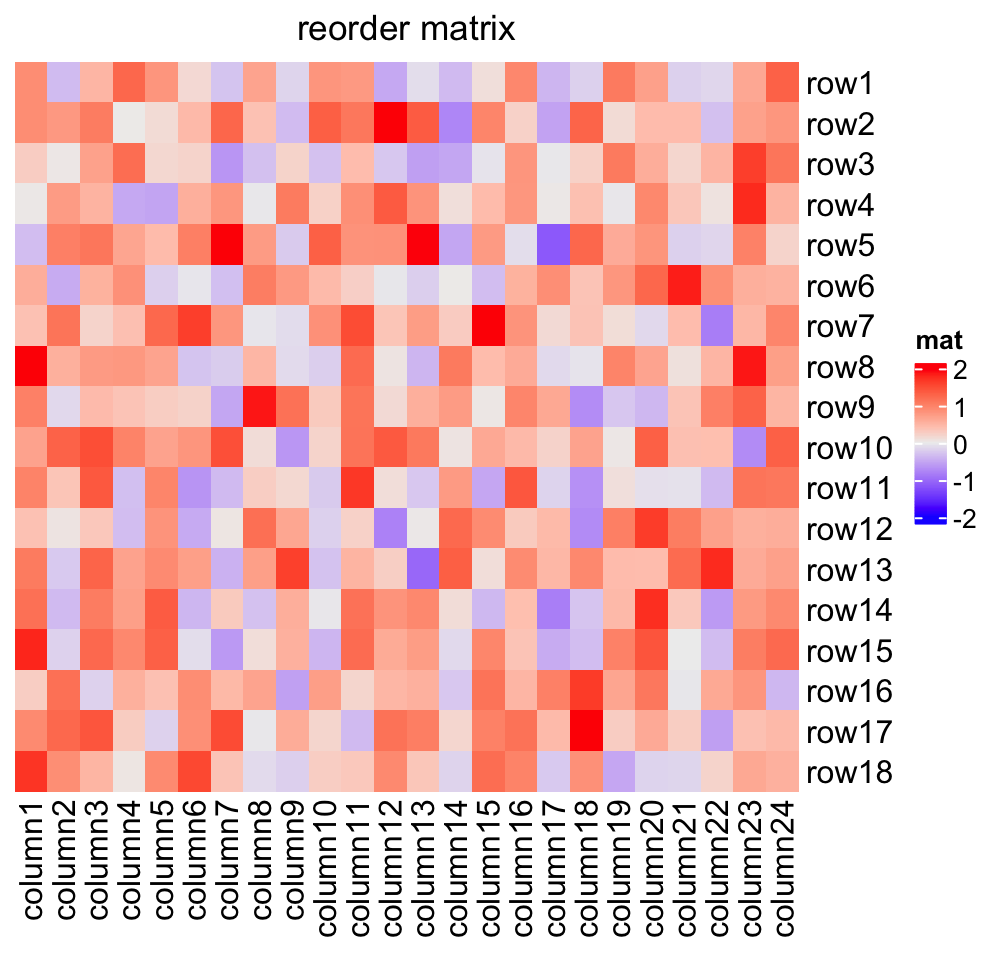The orders can be character vectors if they are just shuffles of the matrix row names or column names.

Heatmap(mat, name = "mat", row_order = sort(rownames(mat)),
column_order = sort(colnames(mat)))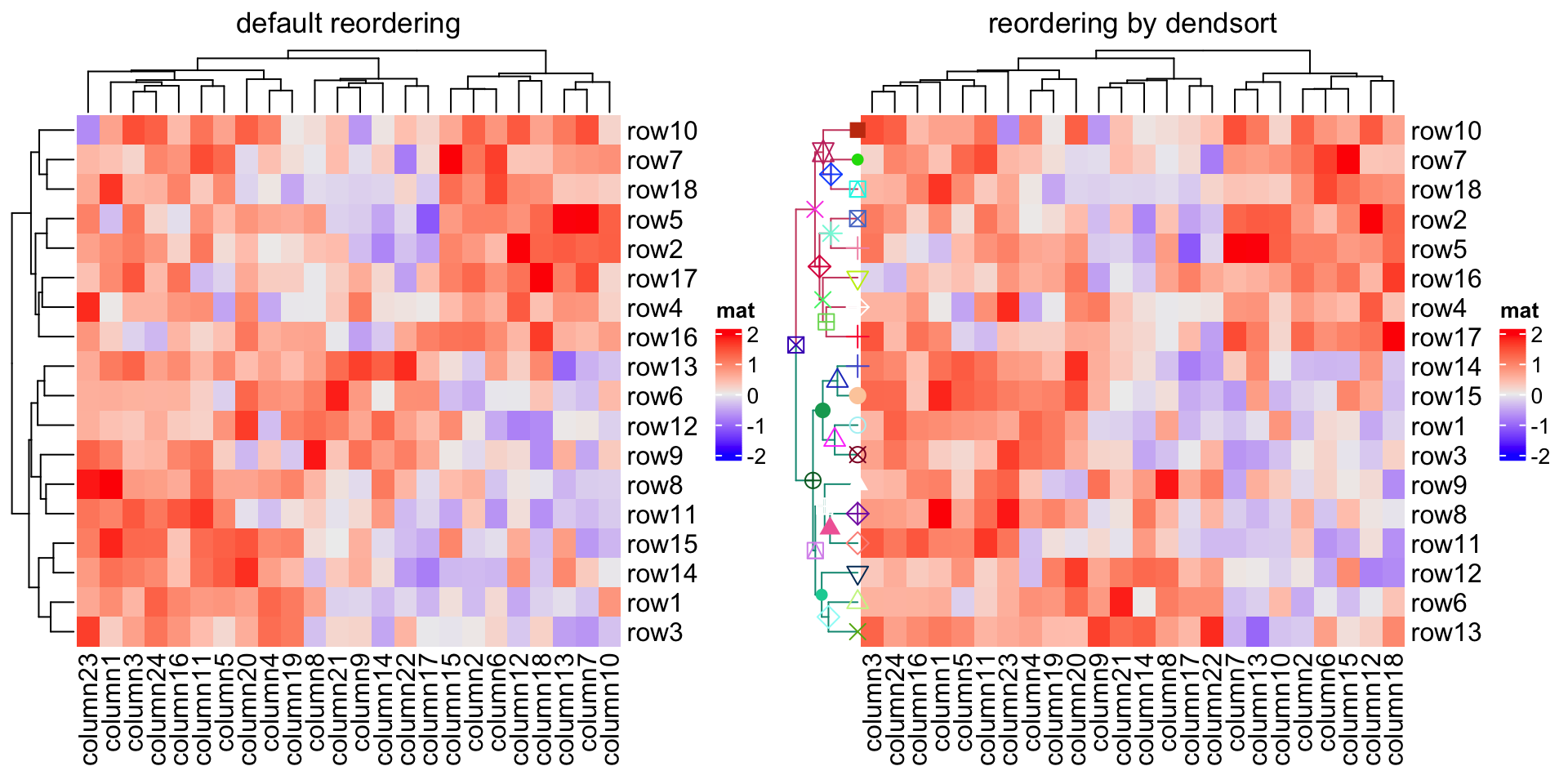Note row_dend_reorder and row_order are two different things. row_dend_reorder is applied on the dendrogram. For any node in the dendrogram, rotating its two branches actually gives an identical dendrogram, thus, reordering the dendrogram by automatically rotating sub-dendrogram at every node can help to separate elements further from each other which show more difference. As a comparison, row_order is simply applied on the matrix and normally dendrograms should be turned off.

## 2.5 Seriation

Seriation is an interesting technique for ordering the matrix (see this interesting post: http://nicolas.kruchten.com/content/2018/02/seriation/). The powerful seriation package implements quite a lot of methods for seriation. Since it is easy to extract row orders and column orders from the object returned by the core function seriate() from seriation package. They can be directly assigned to row_order and column_order to make the heatmap.

The first example demonstrates to directly apply seriate() on the matrix. Since the "BEA_TSP" method only allows a non-negative matrix, we modify the matrix to max(mat) - mat.

library(seriation)
o = seriate(max(mat) - mat, method = "BEA_TSP")
Heatmap(max(mat) - mat, name = "mat",
row_order = get_order(o, 1), column_order = get_order(o, 2))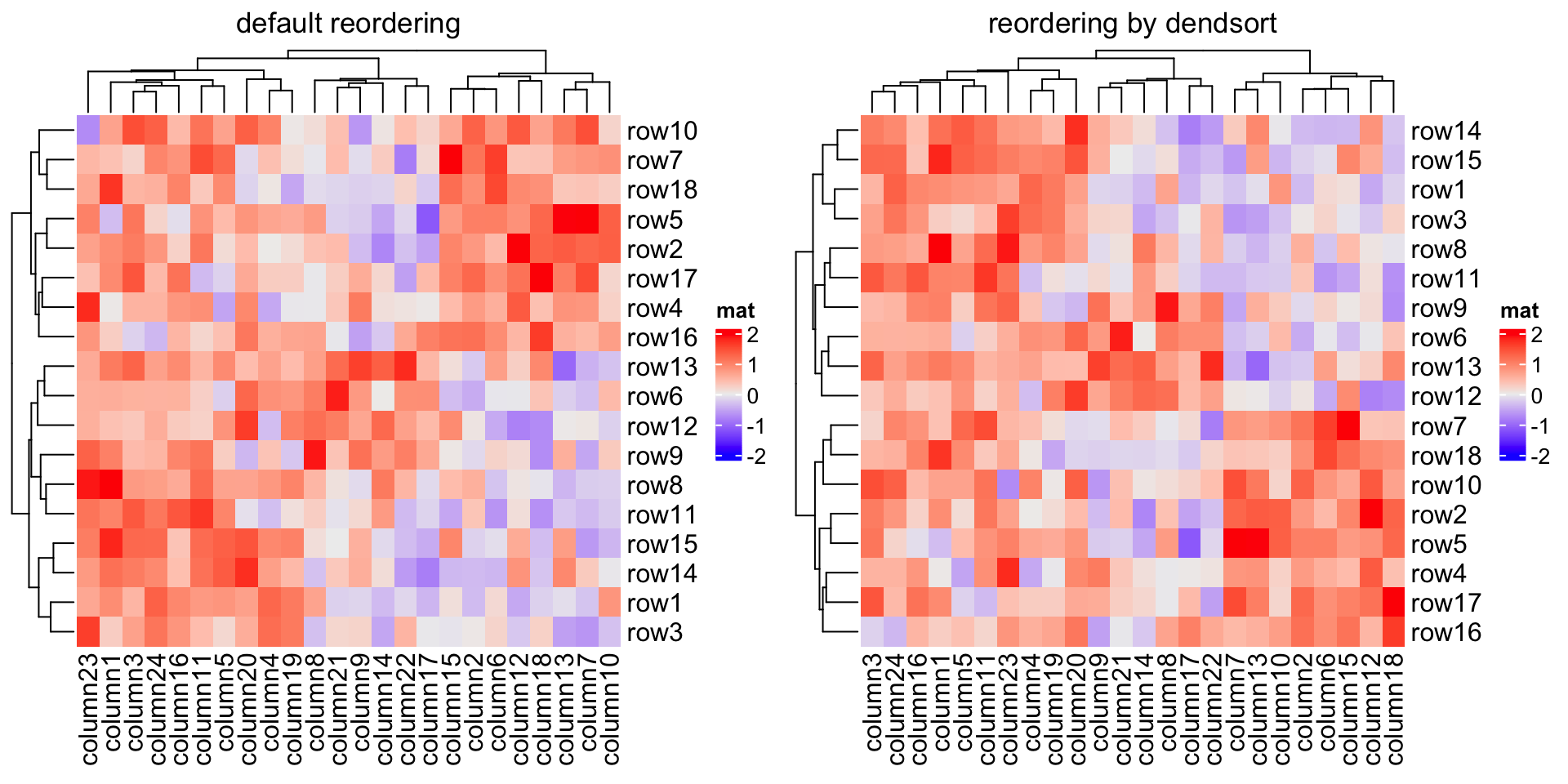Or you can apply seriate() to the distance matrix. Now the order for rows and columns needs to be calcualted separatedly because the distance matrix needs to be calculated separatedly for columns and rows.

o1 = seriate(dist(mat), method = "TSP")
o2 = seriate(dist(t(mat)), method = "TSP")
Heatmap(mat, name = "mat", row_order = get_order(o1), column_order = get_order(o2))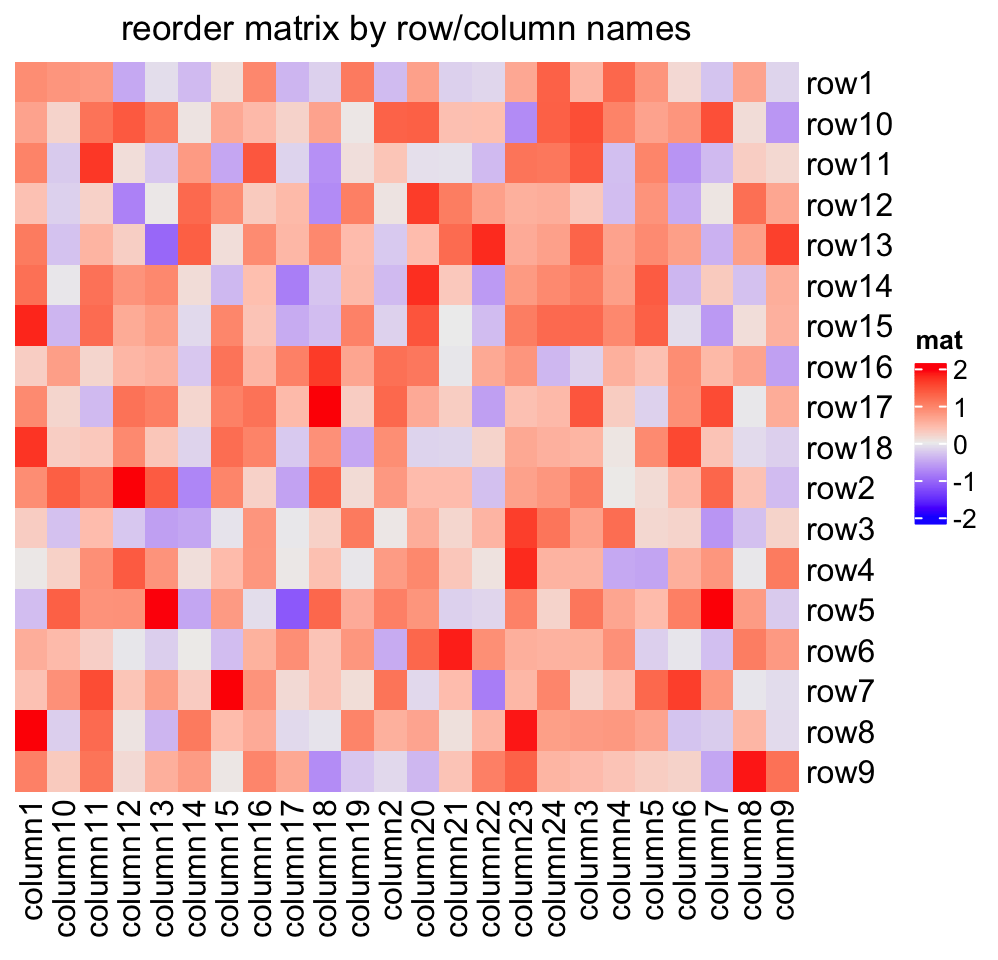Some seriation methods also contain the hierarchical clustering information. Let’s try:

o1 = seriate(dist(mat), method = "GW")
o2 = seriate(dist(t(mat)), method = "GW")

o1 and o2 are actually mainly composed of hclust objects:

class(o1[])
##  "ser_permutation_vector" "hclust"

And the orders are the same by using hclust$order or get_order(). o1[]$order
##    5  2 10 17  4 16 18  7 15 14 11  8  1  3 12  6 13  9
# should be the same as the previous one
get_order(o1)
##    5  2 10 17  4 16 18  7 15 14 11  8  1  3 12  6 13  9

And we can add the dendrograms to the heatmap.

Heatmap(mat, name = "mat", cluster_rows = as.dendrogram(o1[]),
cluster_columns = as.dendrogram(o2[]))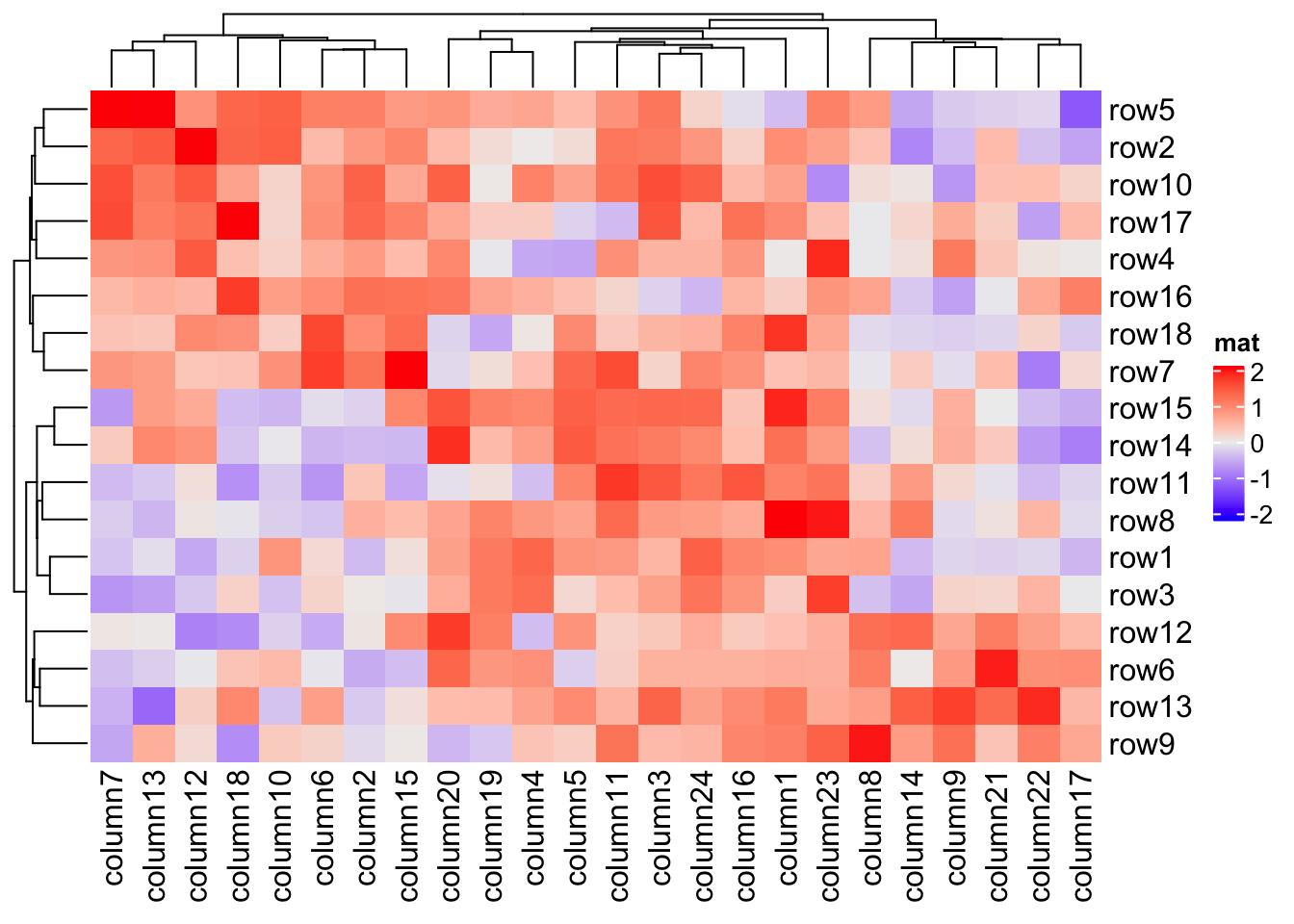For more use of the seriate() function, please refer to the seriation package.

## 2.6 Dimension names

The row names and column names are drawn on the right and bottom sides of the heatmap by default. Side, visibility and graphic parameters for dimension names can be set as follows:

Heatmap(mat, name = "mat", row_names_side = "left", row_dend_side = "right",
column_names_side = "top", column_dend_side = "bottom")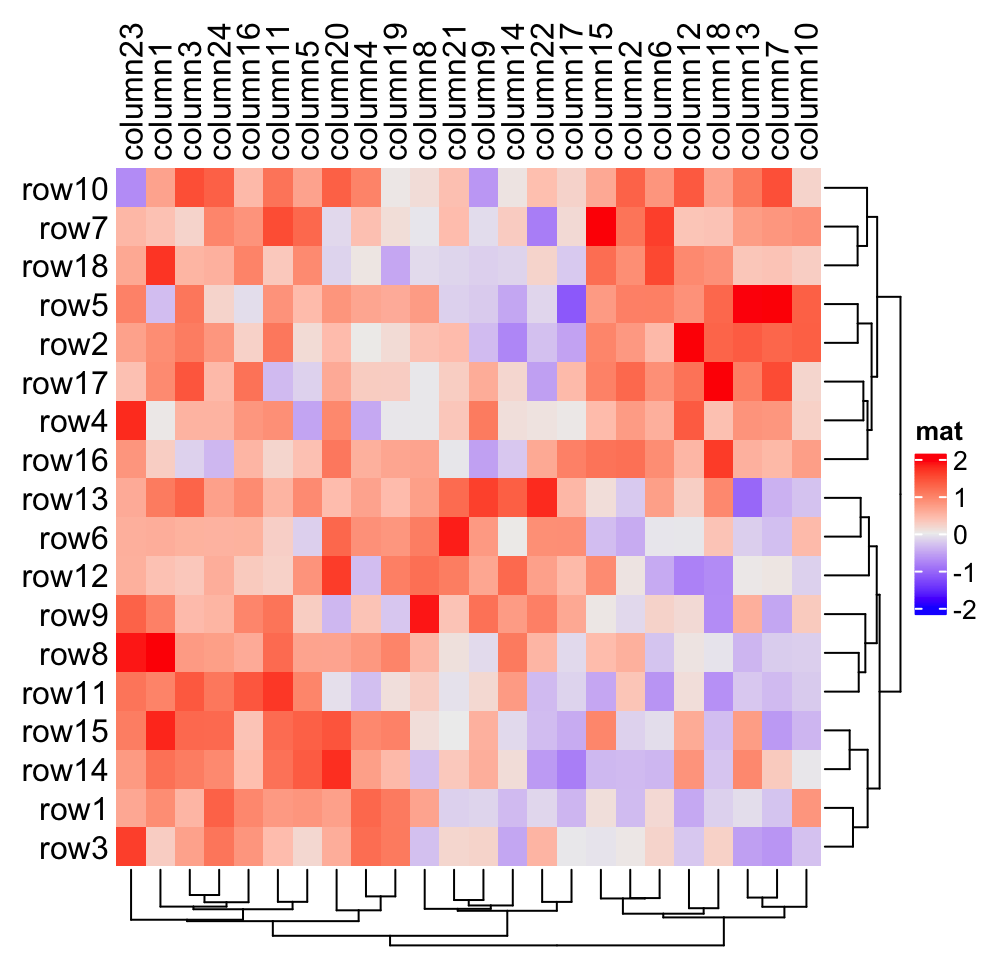Heatmap(mat, name = "mat", show_row_names = FALSE)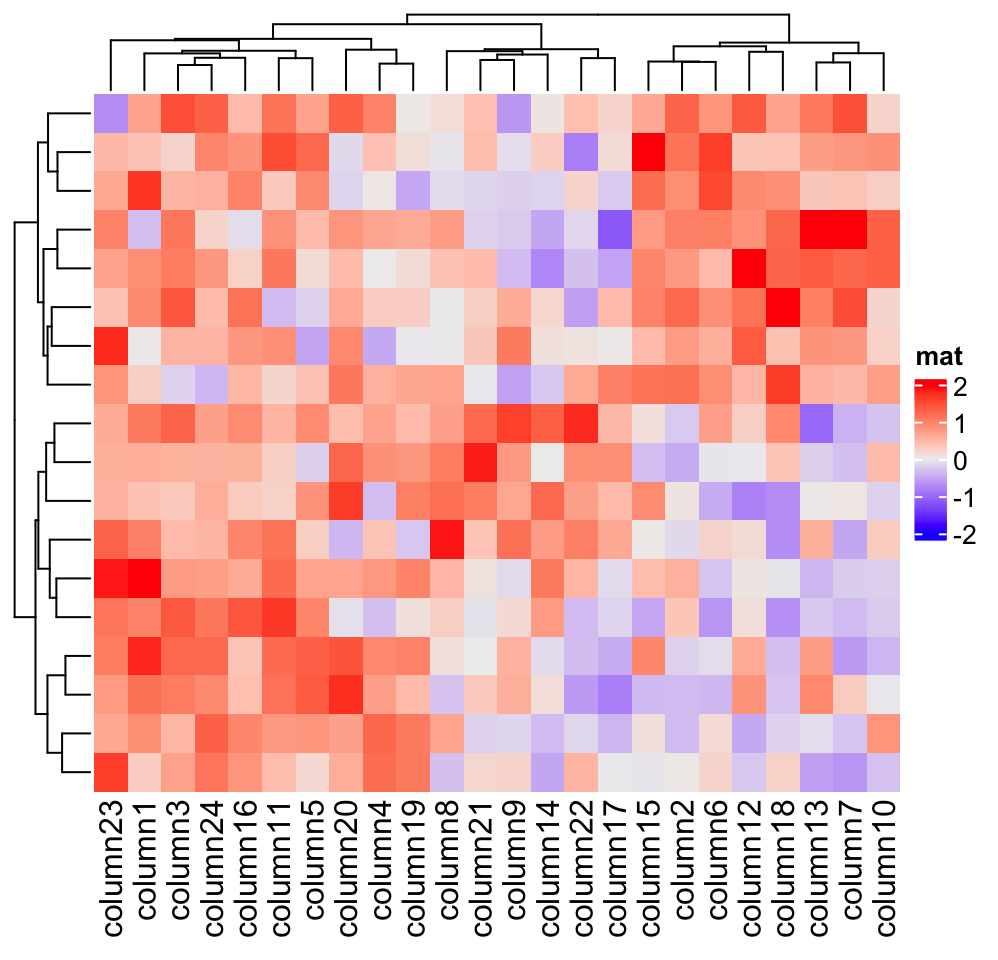Heatmap(mat, name = "mat", row_names_gp = gpar(fontsize = 20))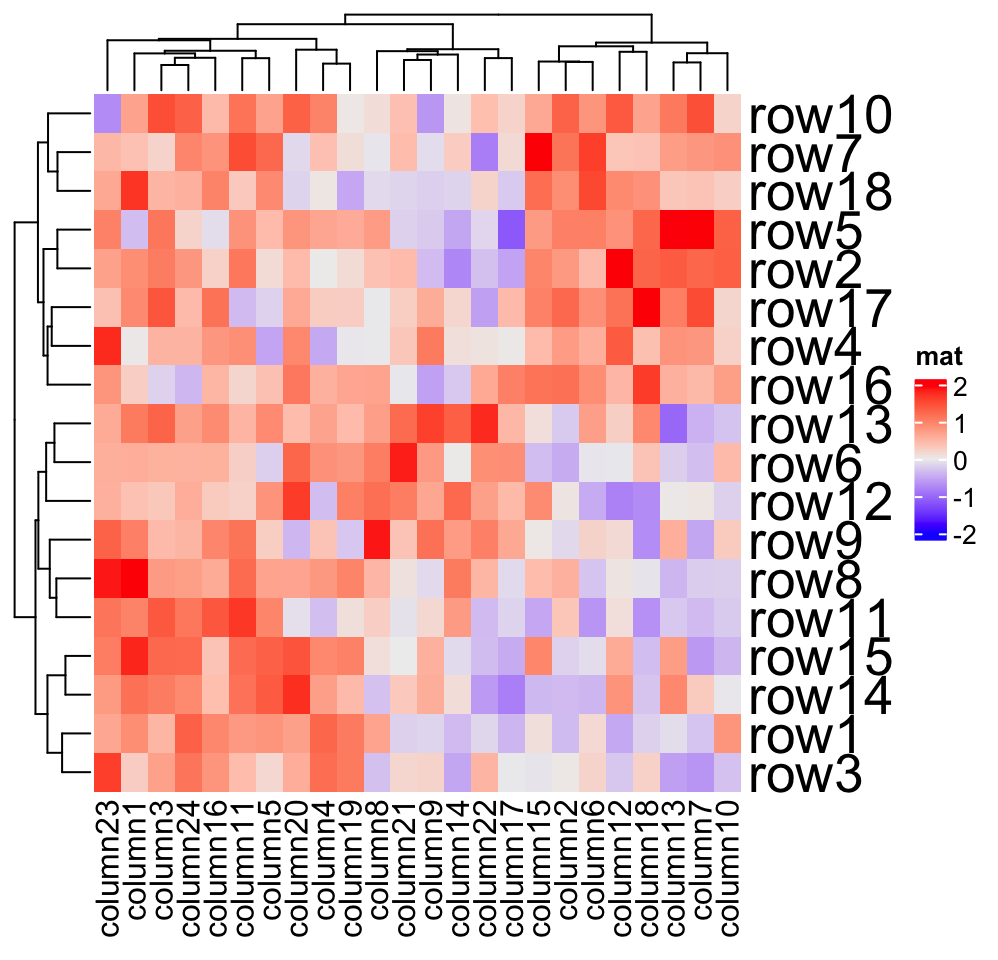Heatmap(mat, name = "mat", row_names_gp = gpar(col = c(rep("red", 10), rep("blue", 8))))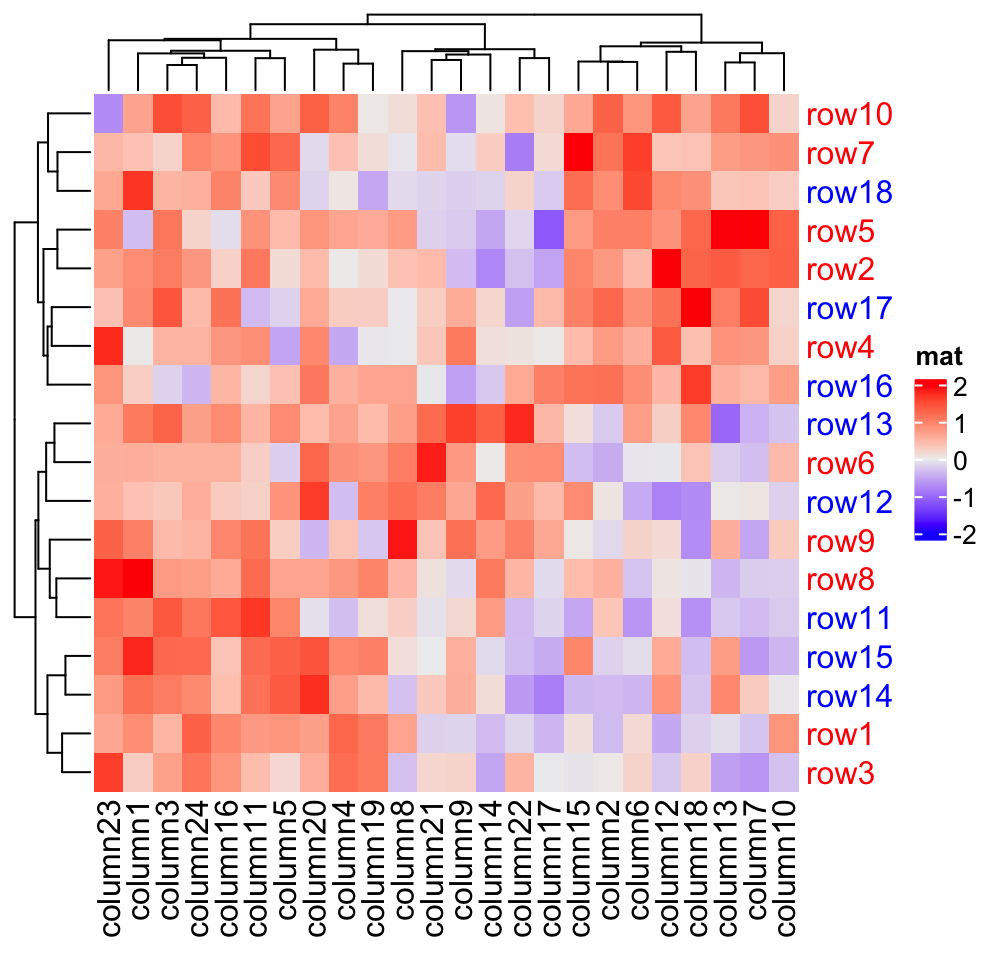Heatmap(mat, name = "mat", row_names_centered = TRUE, column_names_centered = TRUE)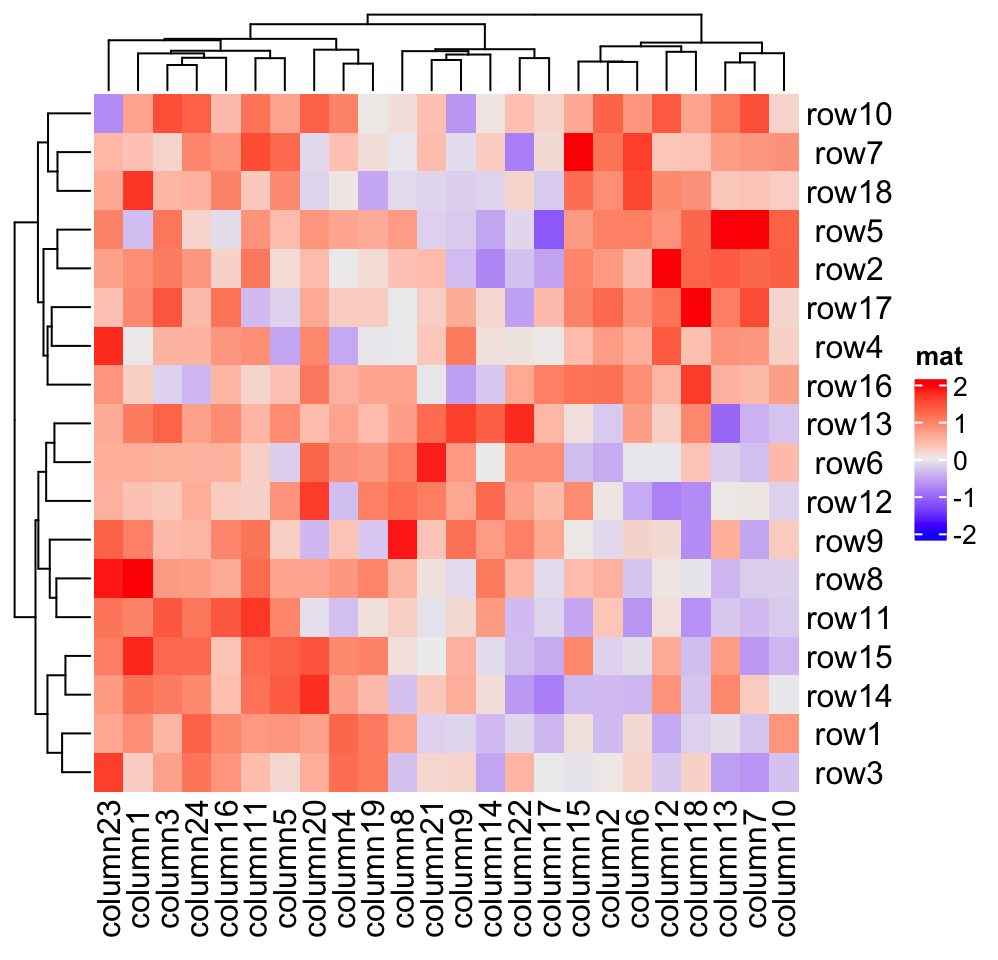The rotation of column names can be set by column_names_rot:

Heatmap(mat, name = "mat", column_names_rot = 45)
Heatmap(mat, name = "mat", column_names_rot = 45, column_names_side = "top",
column_dend_side = "bottom")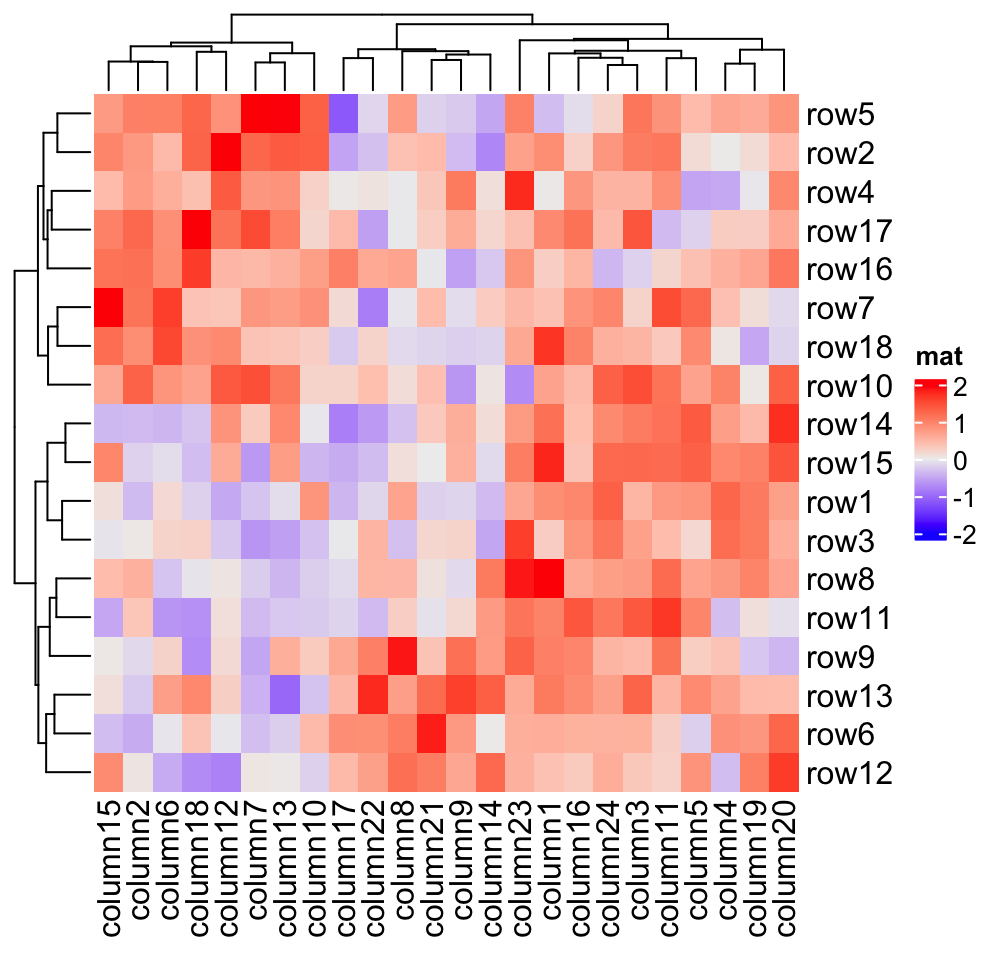If you have row names or column names which are too long, row_names_max_width or column_names_max_height can be used to set the maximal space for them. The default maximal space for row names and column names are all 6 cm. In following code, max_text_width() is a helper function to quick calculate maximal width from a vector of text.

mat2 = mat
rownames(mat2) = paste(c(letters, LETTERS), collapse = "")
Heatmap(mat2, name = "mat")
Heatmap(mat2, name = "mat",
row_names_max_width = max_text_width(
rownames(mat2),
gp = gpar(fontsize = 12)
))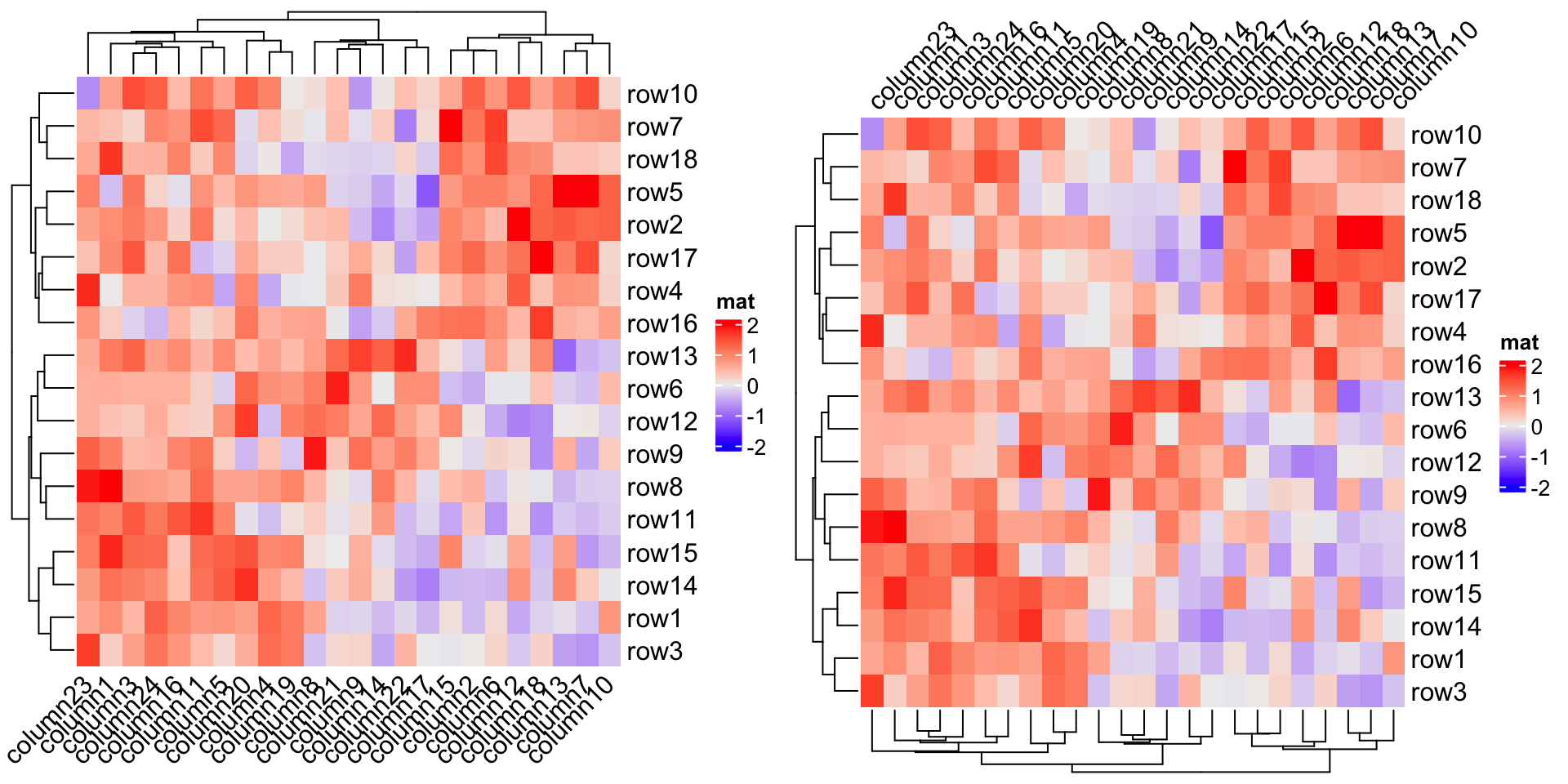Instead of directly using the row/column names from the matrix, you can also provide another character vector which corresponds to the rows or columns and set it by row_labels or column_labels. This is useful because you don’t need to change the dimension names of the matrix to change the labels on the heatmap while you can directly provide the new labels.

There is one typical scenario that row_labels and column_labels are useful. For the gene expression analysis, we might use Ensembl ID as the gene ID which is used as row names of the gene expression matrix. However, the Ensembl ID is for the indexing of the Ensembl database but not for the human reading. Instead, we would prefer to put gene symbols on the heatmap as the row names which is easier to read. To do this, we only need to assign the corresponding gene symbols to row_labels without modifying the original matrix.

The second advantage is row_labels or column_labels allows duplicated labels, while duplicated row names or column names are not allowed in the matrix.

Following gives a simple example that we put letters as row labels and column labels:

# use a named vector to make sure the correspondance between
# row names and row labels is correct
row_labels = structure(paste0(letters[1:24], 1:24), names = paste0("row", 1:24))
column_labels = structure(paste0(LETTERS[1:24], 1:24), names = paste0("column", 1:24))
row_labels
##  row1  row2  row3  row4  row5  row6  row7  row8  row9 row10 row11 row12
##  "a1"  "b2"  "c3"  "d4"  "e5"  "f6"  "g7"  "h8"  "i9" "j10" "k11" "l12"
## row13 row14 row15 row16 row17 row18 row19 row20 row21 row22 row23 row24
## "m13" "n14" "o15" "p16" "q17" "r18" "s19" "t20" "u21" "v22" "w23" "x24"
Heatmap(mat, name = "mat", row_labels = row_labels[rownames(mat)],
column_labels = column_labels[colnames(mat)])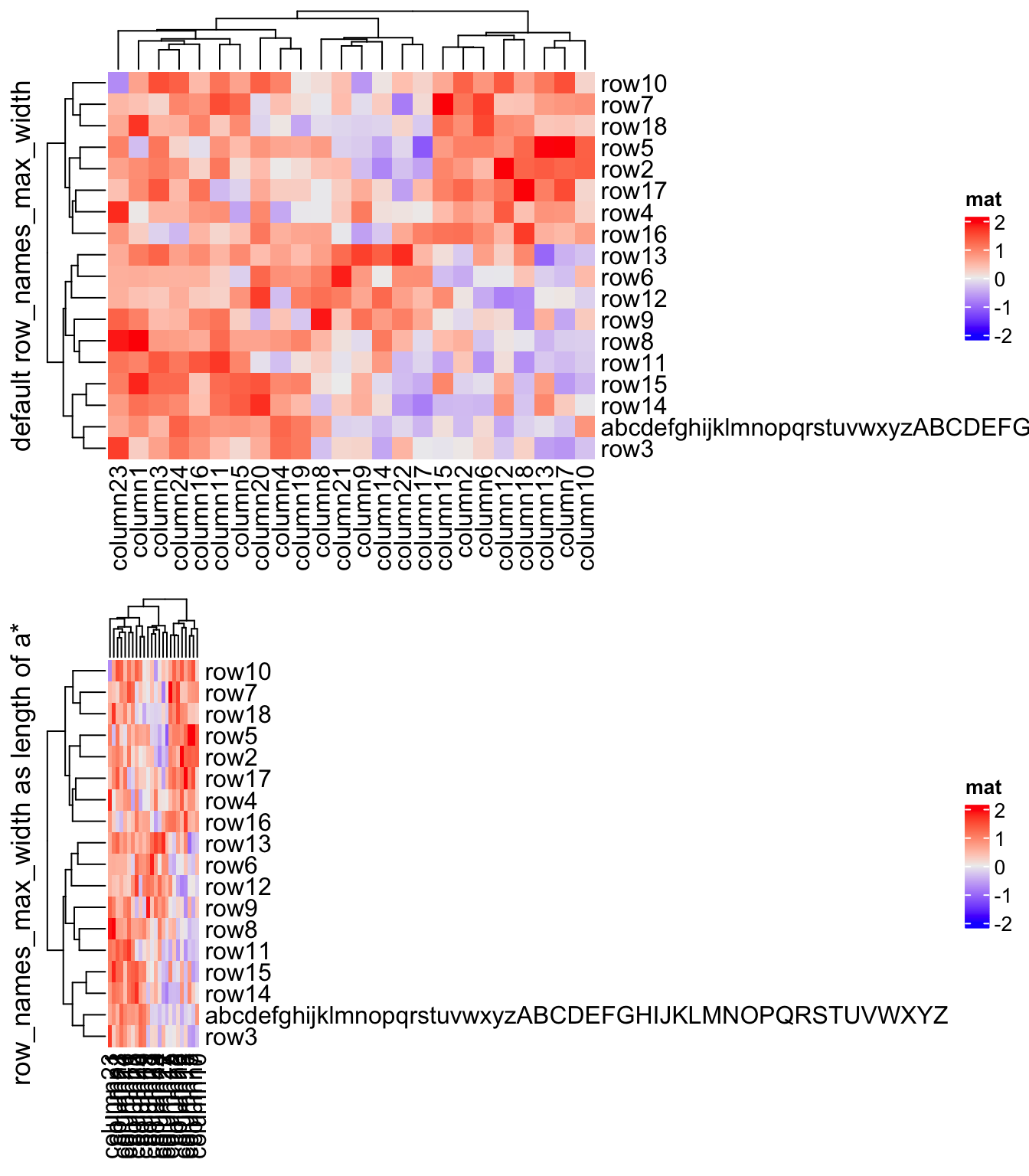The third advantage is mathematical expression can be used as row names in the heatmap.

Heatmap(mat, name = "mat", row_labels = expression(alpha, beta, gamma, delta, epsilon,
zeta, eta, theta, iota, kappa, lambda, mu, nu, xi, omicron, pi, rho, sigma))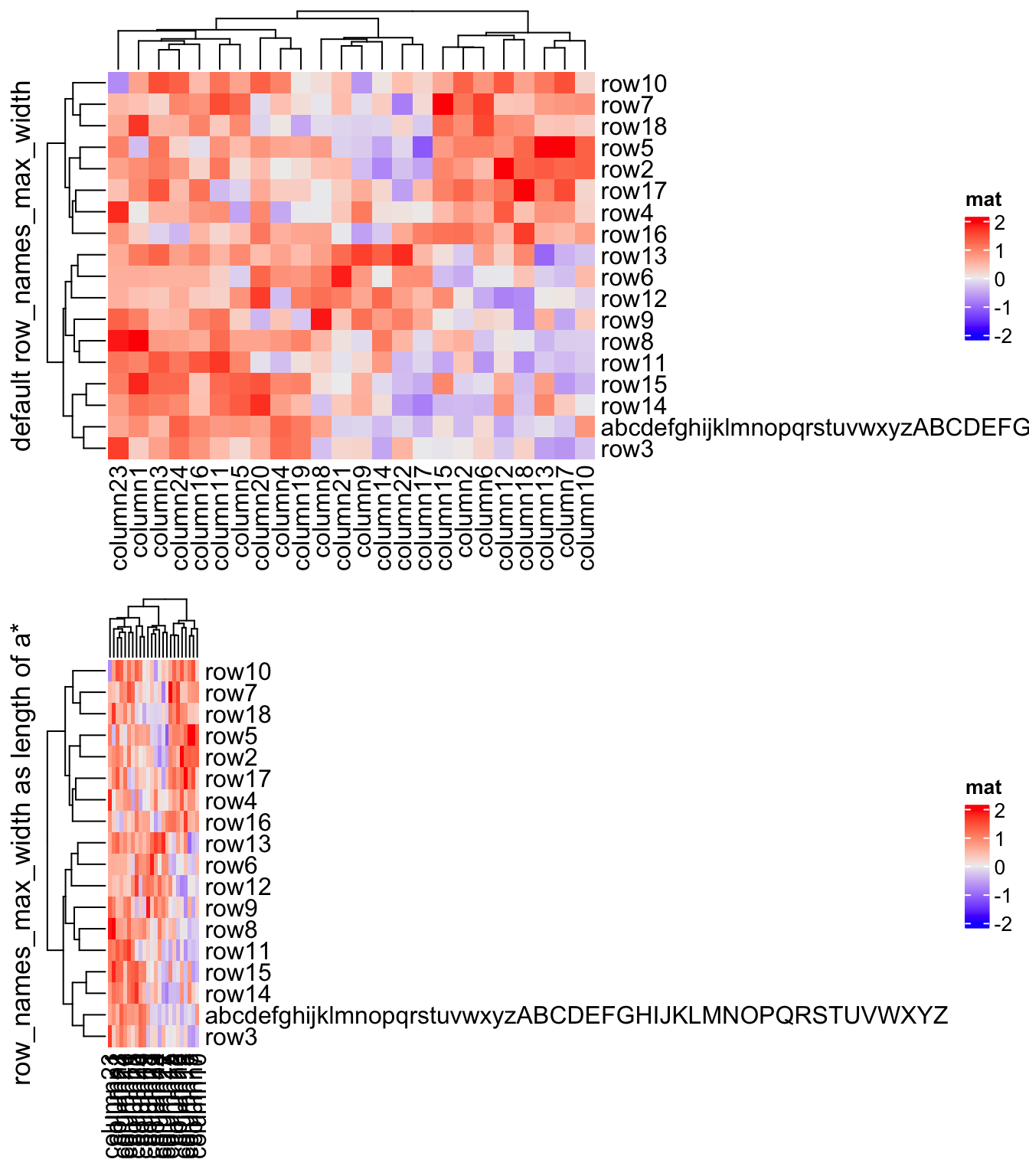anno_text() (Section 3.14) can be used to add more customized labels for heatmap rows and columns.

## 2.7 Heatmap split

One major advantage of ComplexHeatmap package is it supports splitting the heatmap by rows and columns to better group the features and additionally highlight the patterns.

Following arguments control the splitting: row_km, row_split, column_km, column_split. In following, we call the sub-clusters generated by splitting “slices”.

### 2.7.1 Split by k-means clustering

row_km and column_km apply k-means partitioning.

Heatmap(mat, name = "mat", row_km = 2)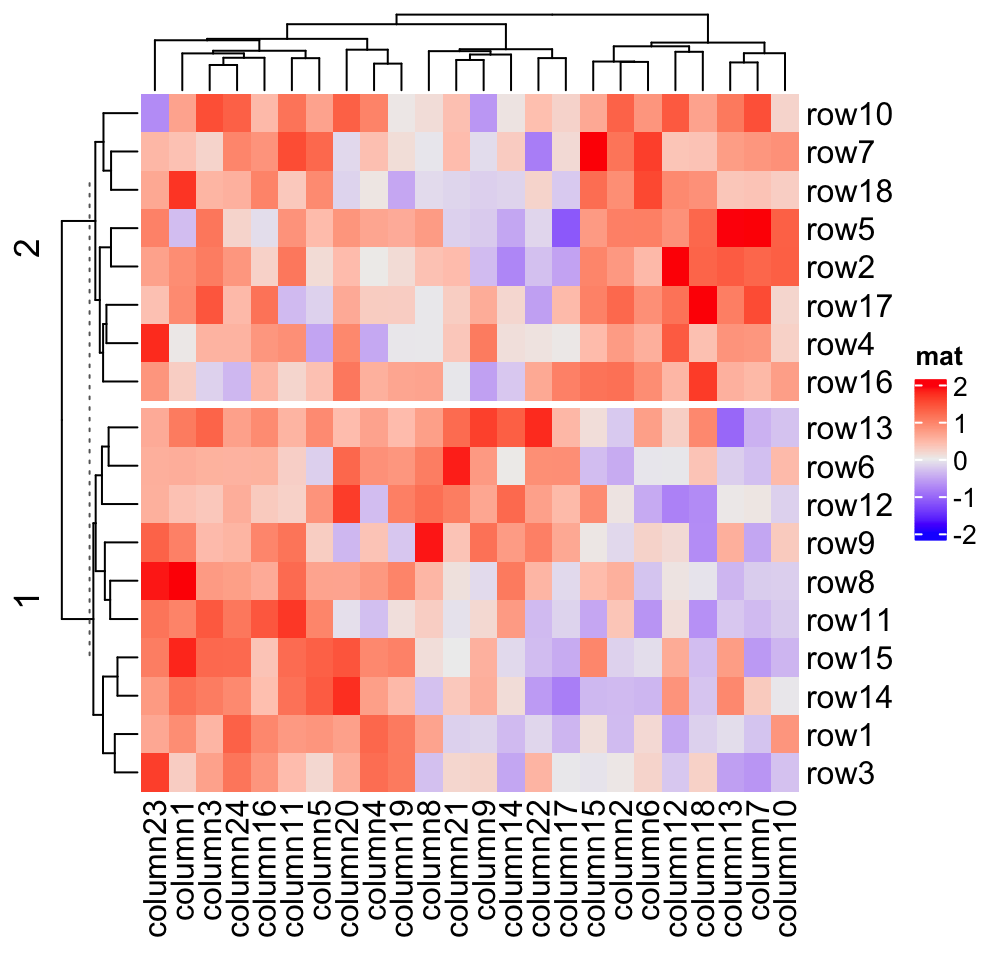Heatmap(mat, name = "mat", column_km = 3)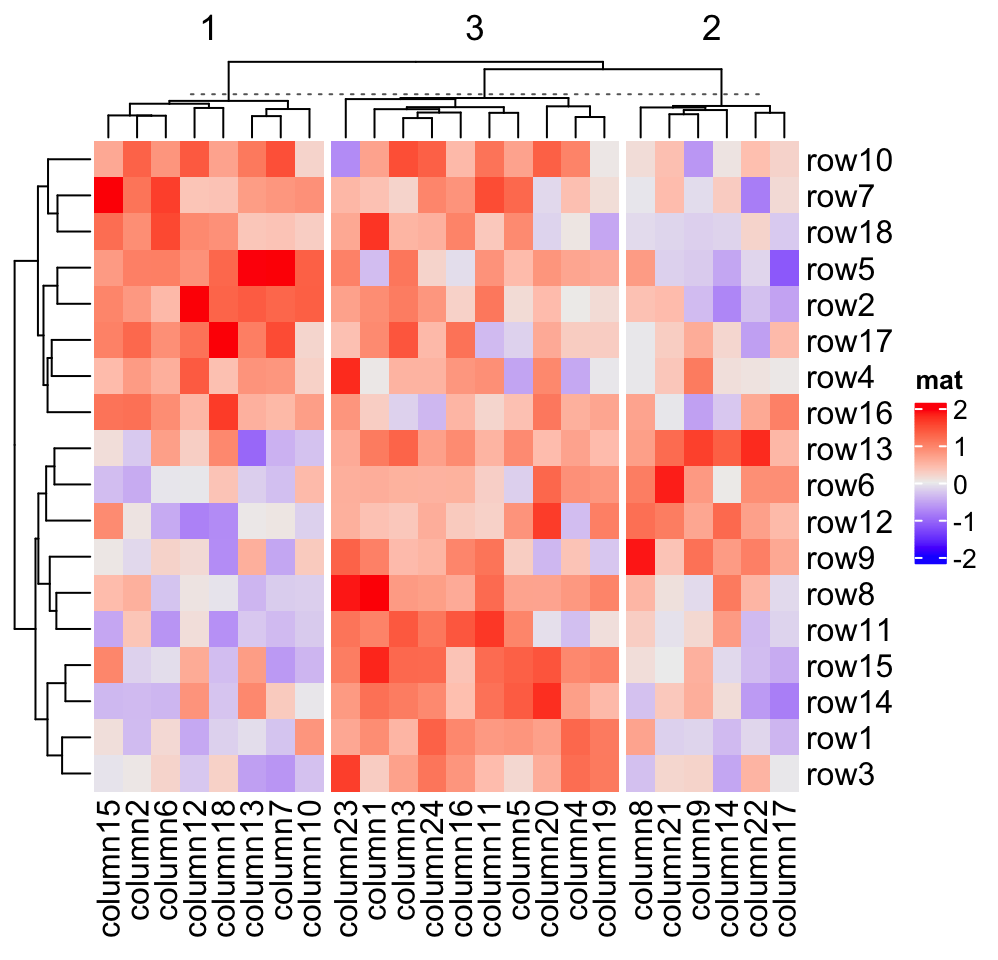Row splitting and column splitting can be performed simultaneously.

Heatmap(mat, name = "mat", row_km = 2, column_km = 3)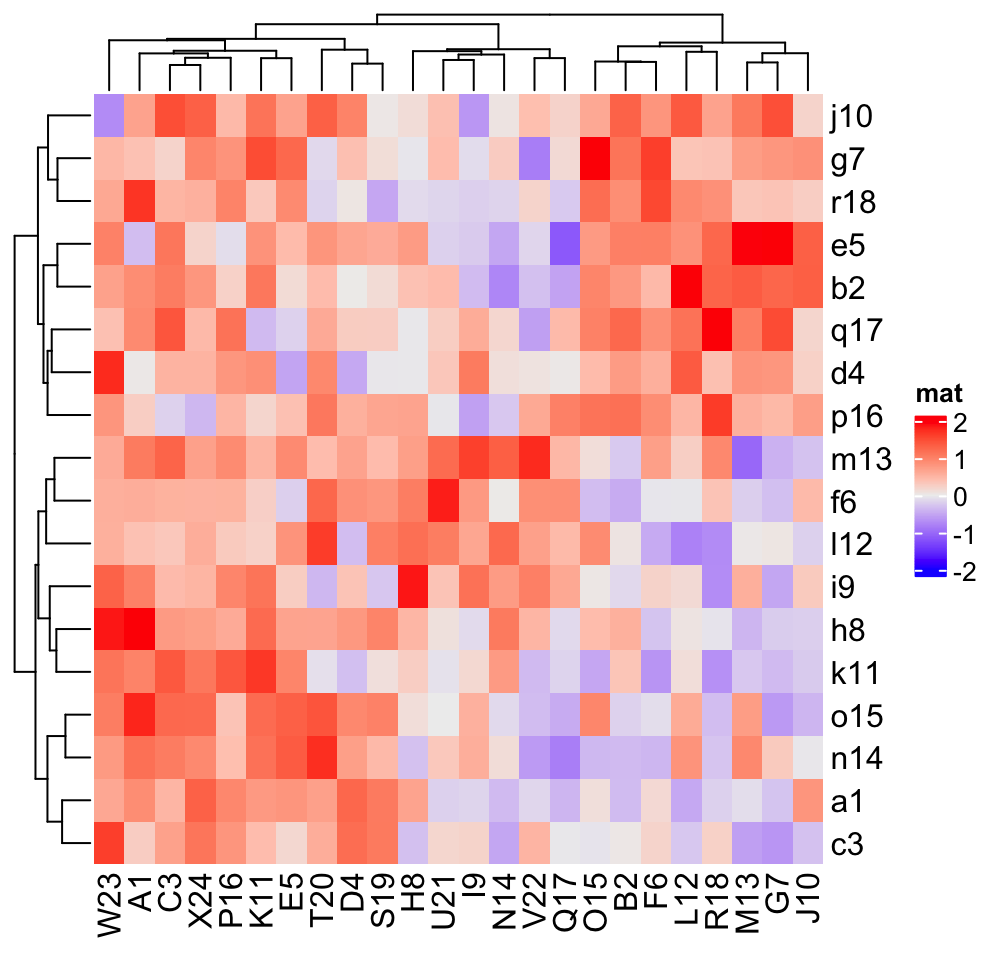You might notice there are dashed lines in the row and column dendrograms, it will be explained in Section 2.7.2 (last paragraph).

Heatmap() internally calls kmeans() with random start points, which results in, for some cases, generating different clusters from repeated runs. To get rid of this problem, row_km_repeats and column_km_repeats can be set to a number larger than 1 to run kmeans() multiple times and a final consensus k-means clustering is used. Please note the final number of clusters form consensus k-means might be smaller than the number set in row_km and column_km.

Heatmap(mat, name = "mat",
row_km = 2, row_km_repeats = 100,
column_km = 3, column_km_repeats = 100)

### 2.7.2 Split by categorical variables

More generally, row_split or column_split can be set to a categorical vector or a data frame where different combinations of levels split the rows/columns in the heatmap. How to control the order of the slices is introduced in Section 2.7.4.

# split by a vector
Heatmap(mat, name = "mat",
row_split = rep(c("A", "B"), 9), column_split = rep(c("C", "D"), 12))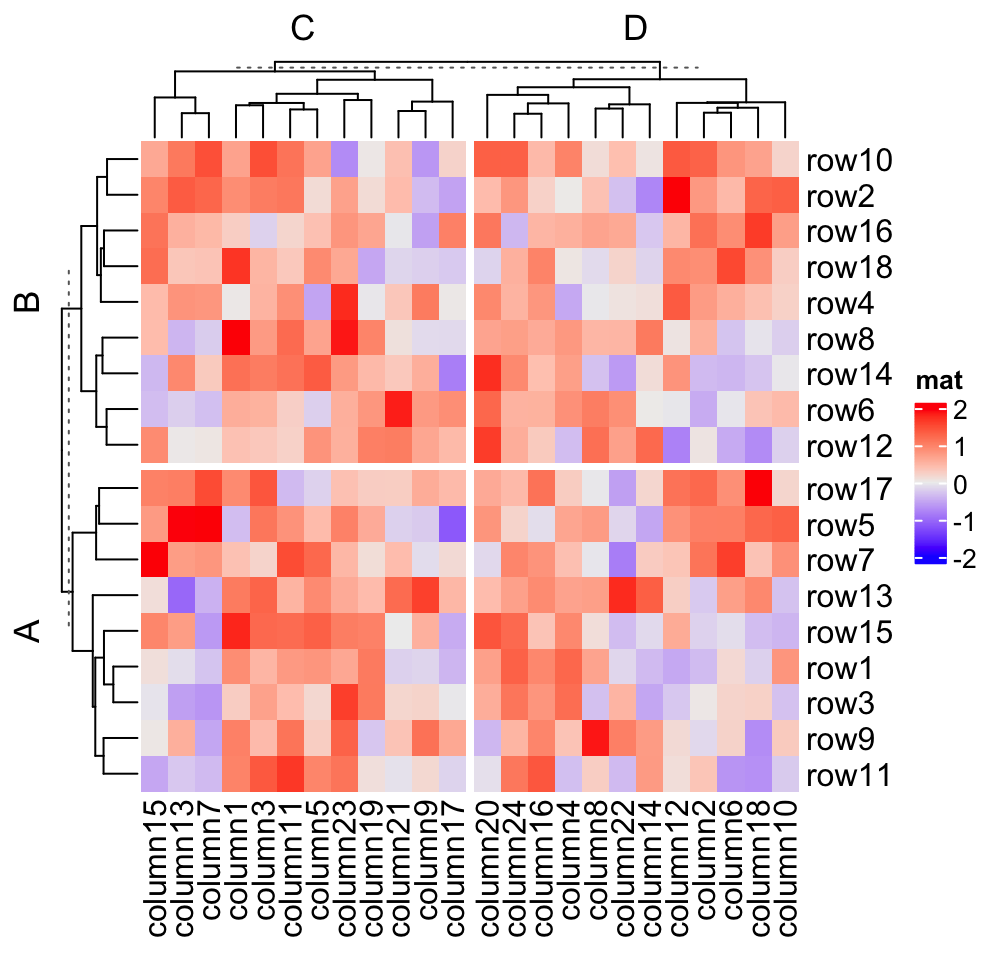# split by a data frame
Heatmap(mat, name = "mat",
row_split = data.frame(rep(c("A", "B"), 9), rep(c("C", "D"), each = 9)))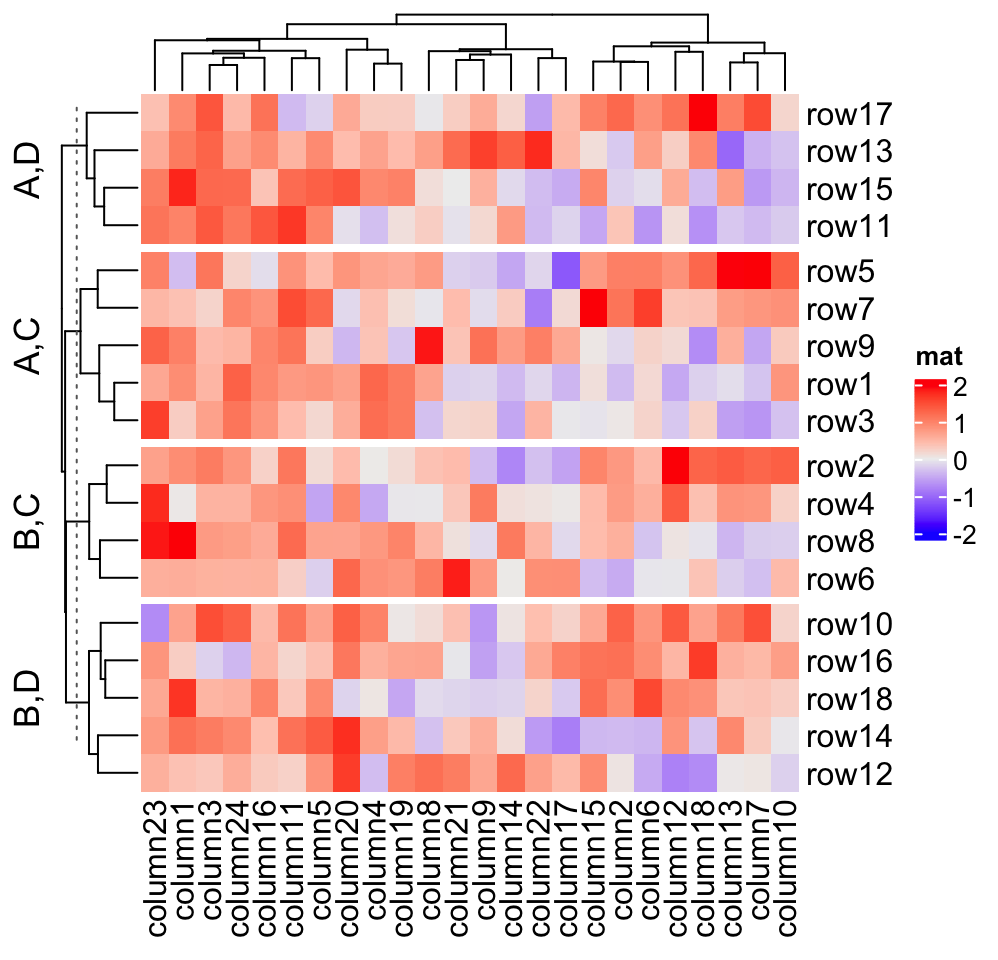# split on both dimensions
Heatmap(mat, name = "mat", row_split = factor(rep(c("A", "B"), 9)),
column_split = factor(rep(c("C", "D"), 12)))Actually, k-means clustering just generates a vector of cluster classes and appends to row_split or column_split. row_km/column_km and be used mixed with row_split and column_split.

Heatmap(mat, name = "mat", row_split = rep(c("A", "B"), 9), row_km = 2)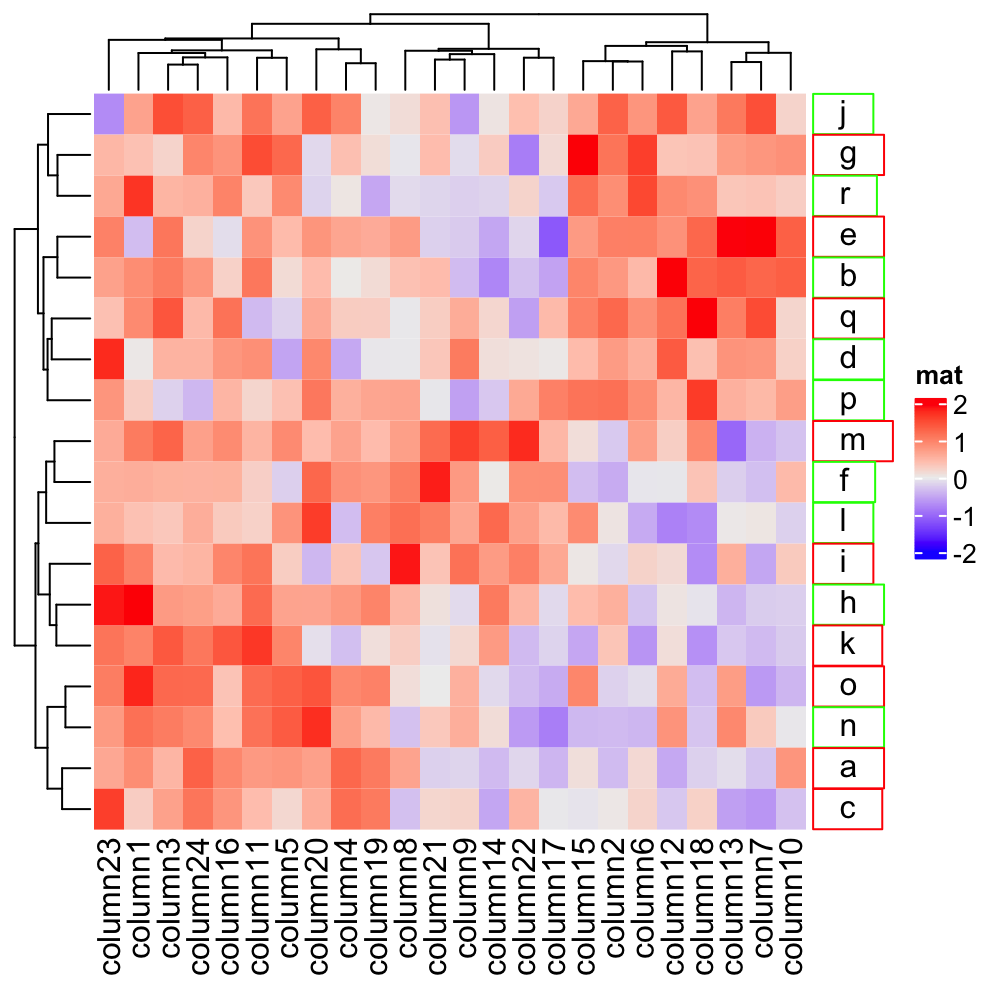which is the same as:

# code only for demonstration
cl = kmeans(mat, centers = 2)$cluster # classes from k-means are always put as the first column in row_split Heatmap(mat, name = "mat", row_split = cbind(cl, rep(c("A", "B"), 9))) If you are not happy with the default k-means partition, it is easy to use other partition methods by just assigning the partition vector to row_split/column_split. pa = cluster::pam(mat, k = 3) Heatmap(mat, name = "mat", row_split = paste0("pam", pa$clustering))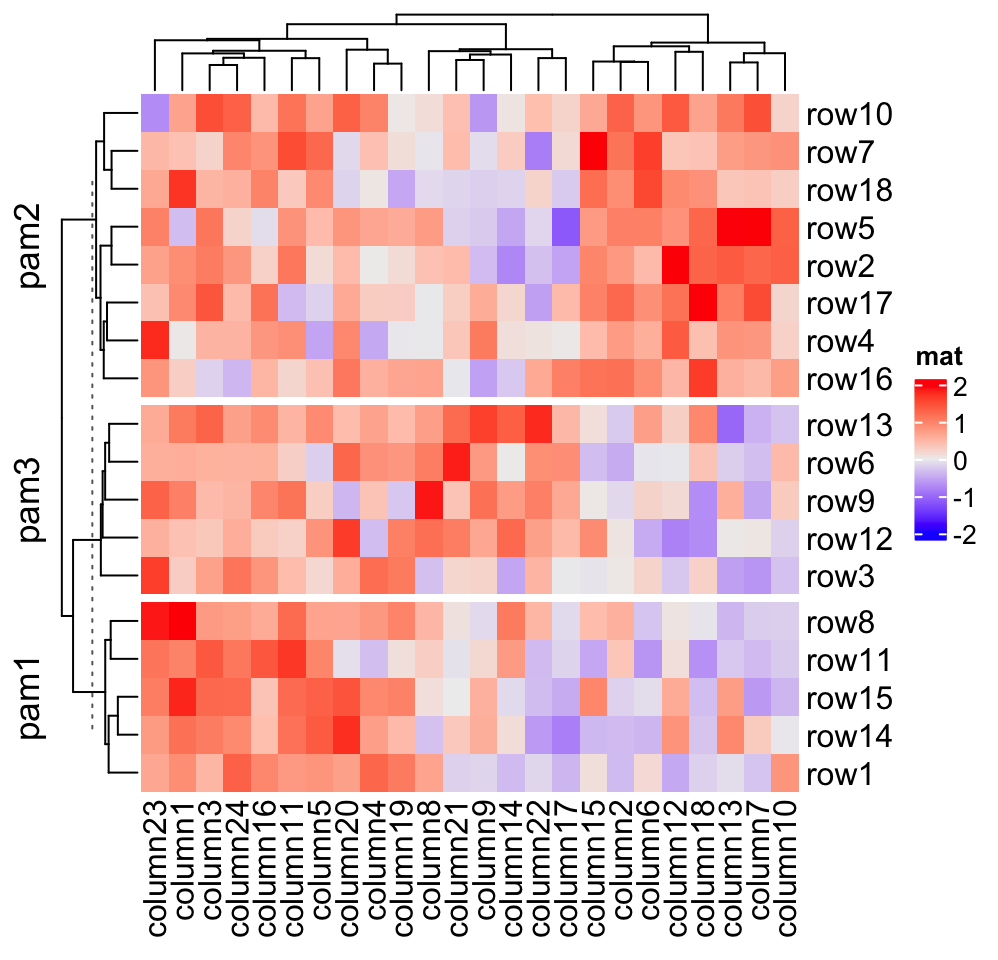If row_order or column_order is set, in each row/column slice, it is still ordered.

# remember when row_order is set, row clustering is turned off
Heatmap(mat, name = "mat", row_order = 18:1, row_km = 2)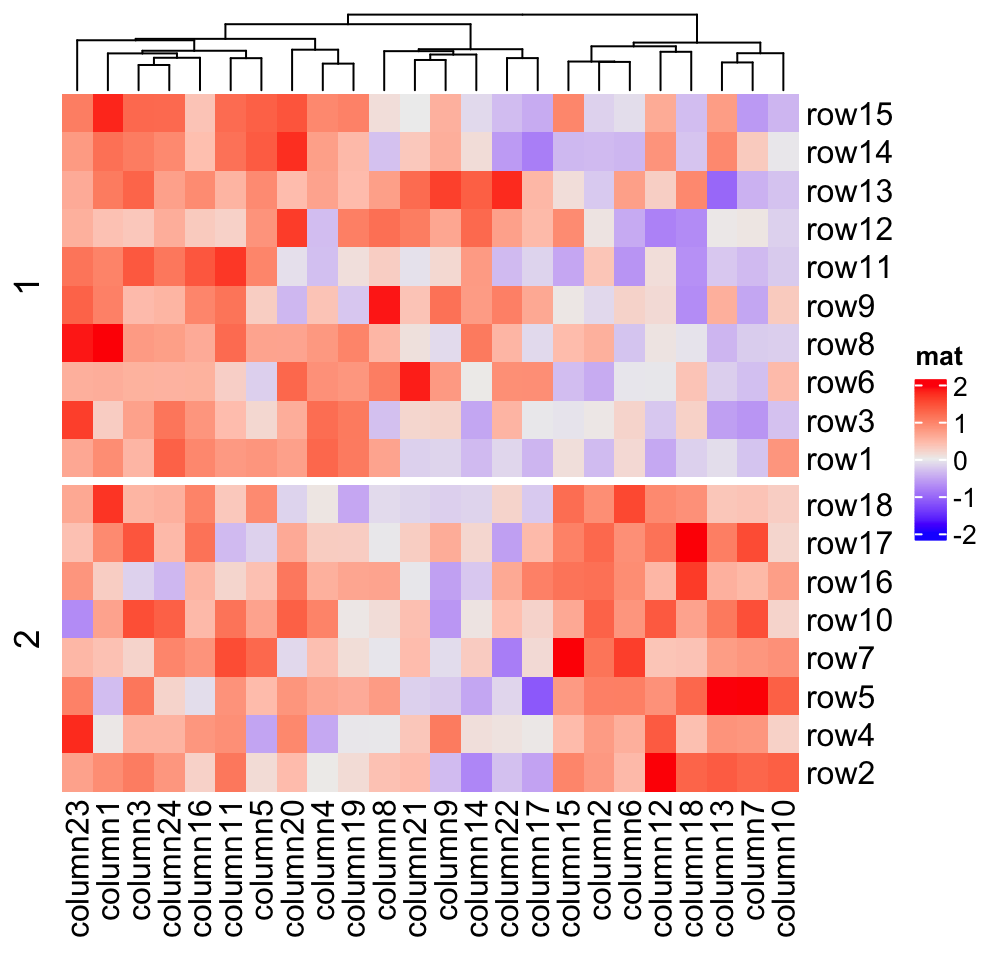Character matrix can only be split by row_split/column_split argument.

# split by the first column in discrete_mat
Heatmap(discrete_mat, name = "mat", col = 1:4, row_split = discrete_mat[, 1])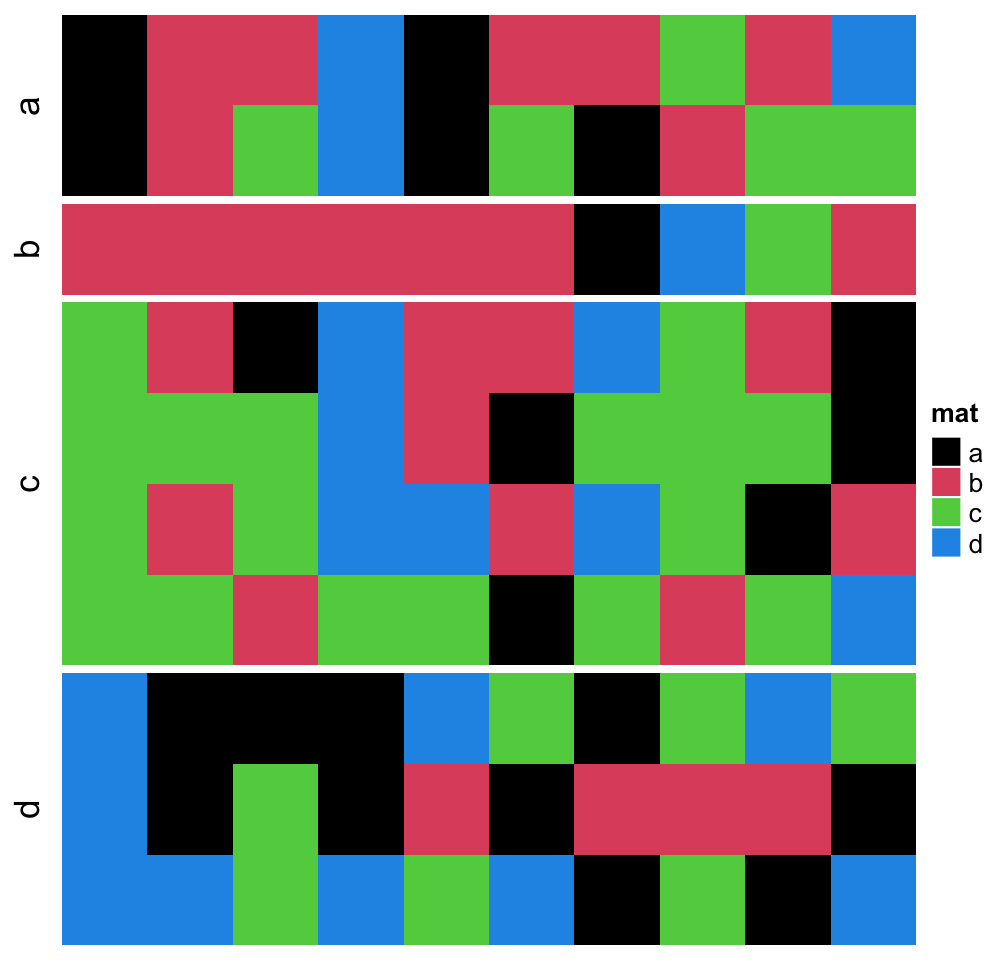If row_km/column_km is set or row_split/column_split is set as a vector or a data frame, hierarchical clustering is first applied to each slice (of course, clustering should be turned on) which generates k dendrograms, then a parent dendrogram is generated based on the mean values of each slice. The height of the parent dendrogram is adjusted by adding the maximal height of the dendrograms in all children slices and the parent dendrogram is added on top of the children dendrograms to form a single global dendrogram. This is why you see dashed lines in the dendrograms in previous heatmaps. They are used to mark the parent dendrogram and the children dendrograms, and alert users they are calculated in different ways. These dashed lines can be removed by setting show_parent_dend_line = FALSE in Heatmap(), or set it as a global option: ht_opt$show_parent_dend_line = FALSE. Heatmap(mat, name = "mat", row_km = 2, column_km = 3, show_parent_dend_line = FALSE)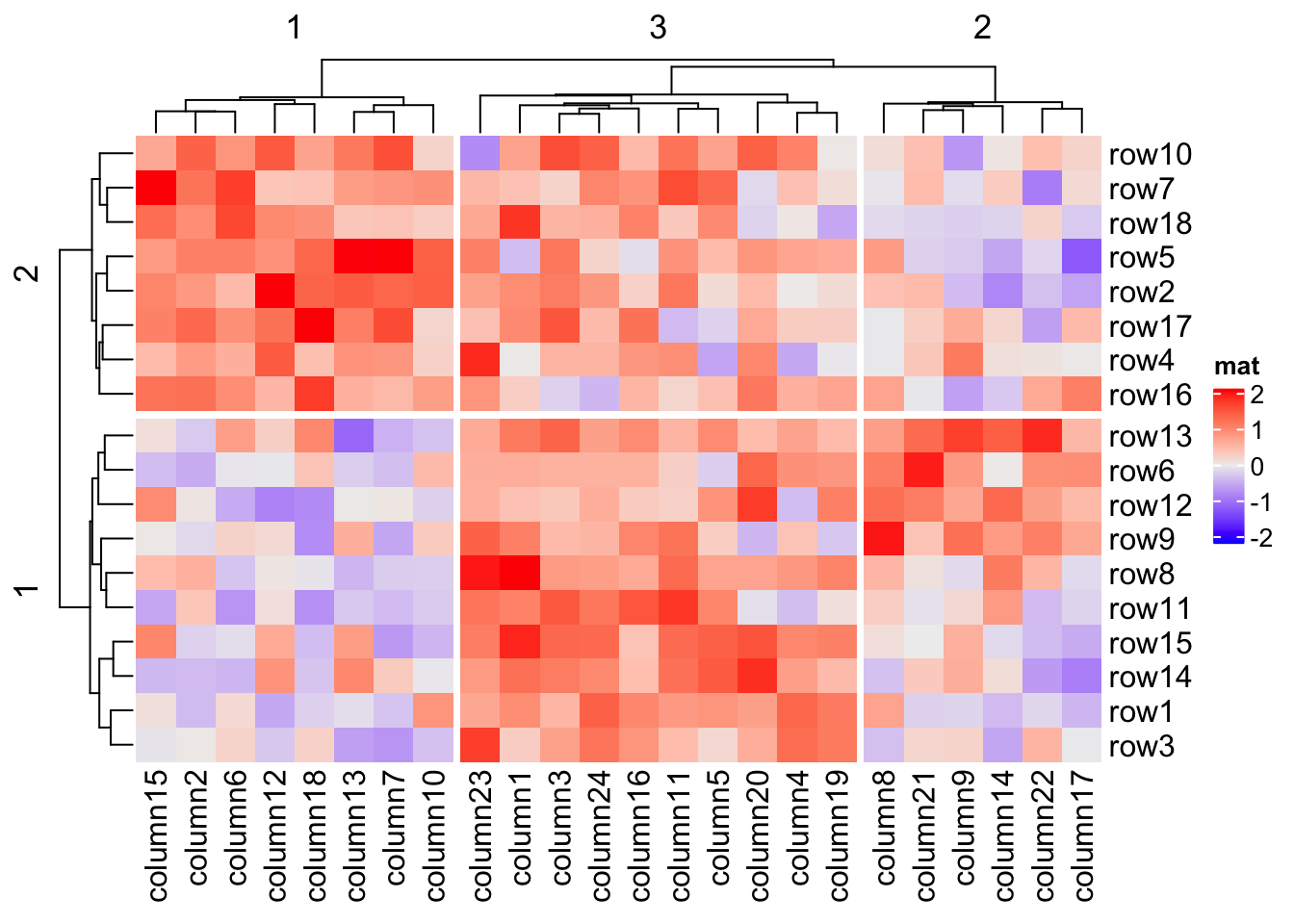### 2.7.3 Split by dendrogram A second scenario for splitting is that users may still want to keep the global dendrogram which is generated from the complete matrix while not split it in the first place. In this case, row_split/column_split can be set to a single number which will apply cutree() on the row/column dendrogram. This works when cluster_rows/cluster_columns is set to TRUE or is assigned with a hclust/dendrogram object. For this case, the dendrogram is still as same as the original one, expect the positions of dendrogram leaves are slightly adjusted by the gaps between slices. (There is no dashed lines, because here the dendrogram is calcualted as a complete one and there is no parent dendrogram or children dendrograms.) Heatmap(mat, name = "mat", row_split = 2, column_split = 3)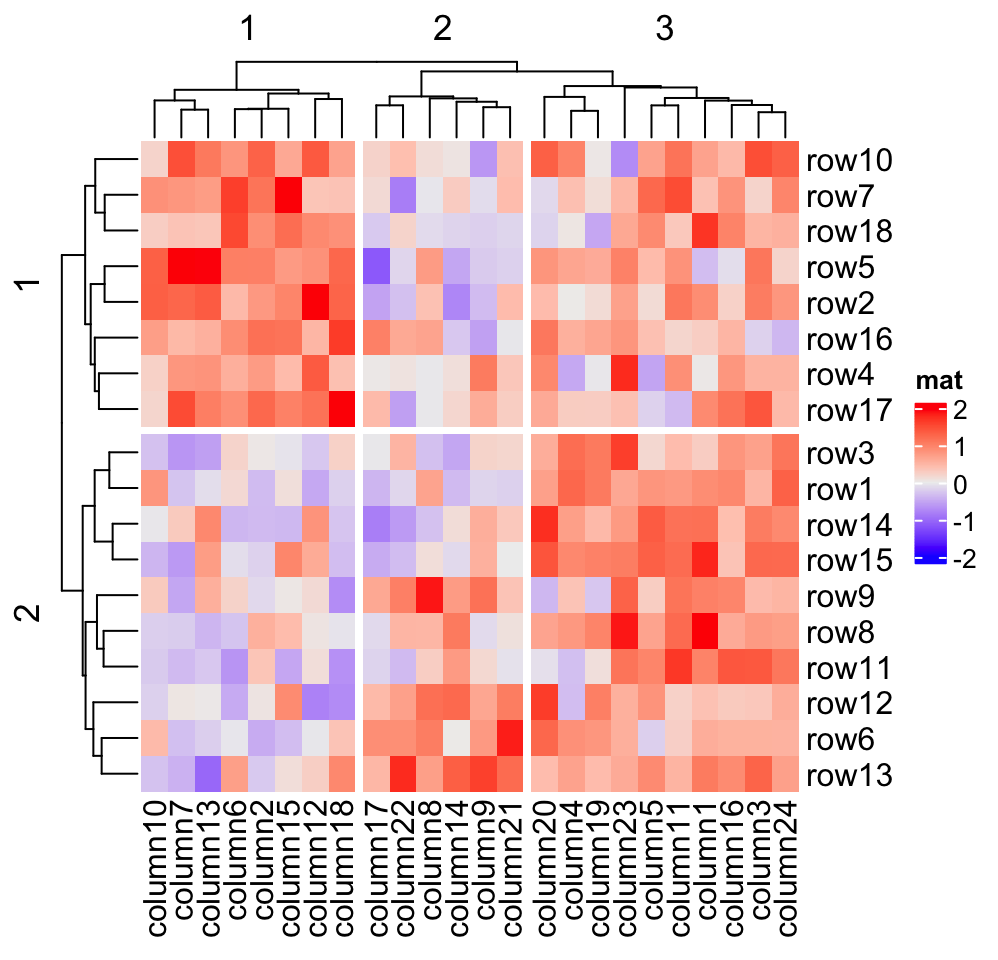dend = hclust(dist(mat)) dend = color_branches(dend, k = 2) Heatmap(mat, name = "mat", cluster_rows = dend, row_split = 2)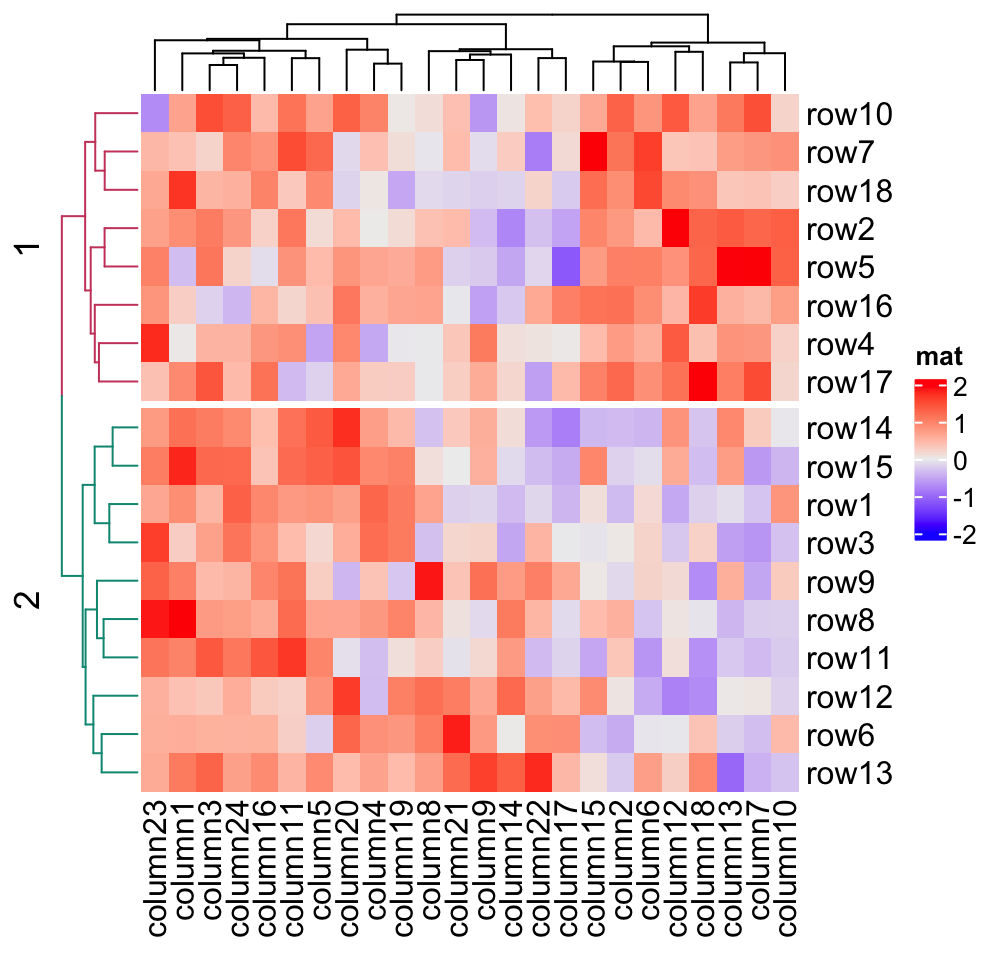If you want to combine splitting from cutree() and other categorical variables, you need to generate the classes from cutree() in the first place, append to e.g. row_split as a data frame and then send it to row_split argument. # code only for demonstration split = data.frame(cutree(hclust(dist(mat)), k = 2), rep(c("A", "B"), 9)) Heatmap(mat, name = "mat", row_split = split) ### 2.7.4 Order of slices When row_split/column_split is set as categorical variable (a vector or a data frame) or row_km/column_km is set, by default, there is an additional clustering applied to the mean of slices to show the hierarchy in the slice level. Under this scenario, you cannot precisely control the order of slices because it is controlled by the clustering of slices. Nevertheless, you can set cluster_row_slices or cluster_column_slices to FALSE to turn off the clustering on slices, and now you can precisely control the order of slices. When there is no slice clustering, the order of each slice can be controlled by levels of each variable in row_split/column_split (in this case, each variable should be a factor). If all variables are characters, the default order is unique(row_split) or unique(column_split). Compare following heatmaps: Heatmap(mat, name = "mat", row_split = rep(LETTERS[1:3], 6), column_split = rep(letters[1:6], 4))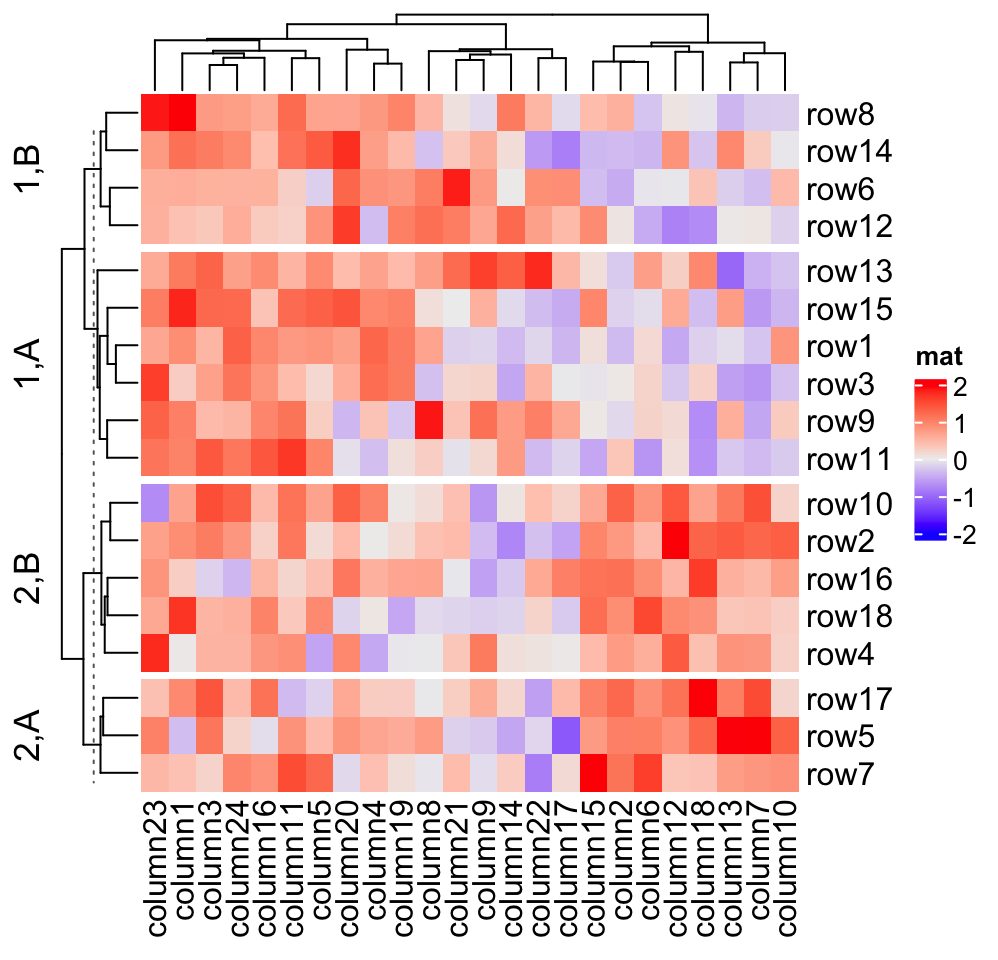# clustering is similar as previous heatmap with branches in some nodes in the dendrogram flipped Heatmap(mat, name = "mat", row_split = factor(rep(LETTERS[1:3], 6), levels = LETTERS[3:1]), column_split = factor(rep(letters[1:6], 4), levels = letters[6:1]))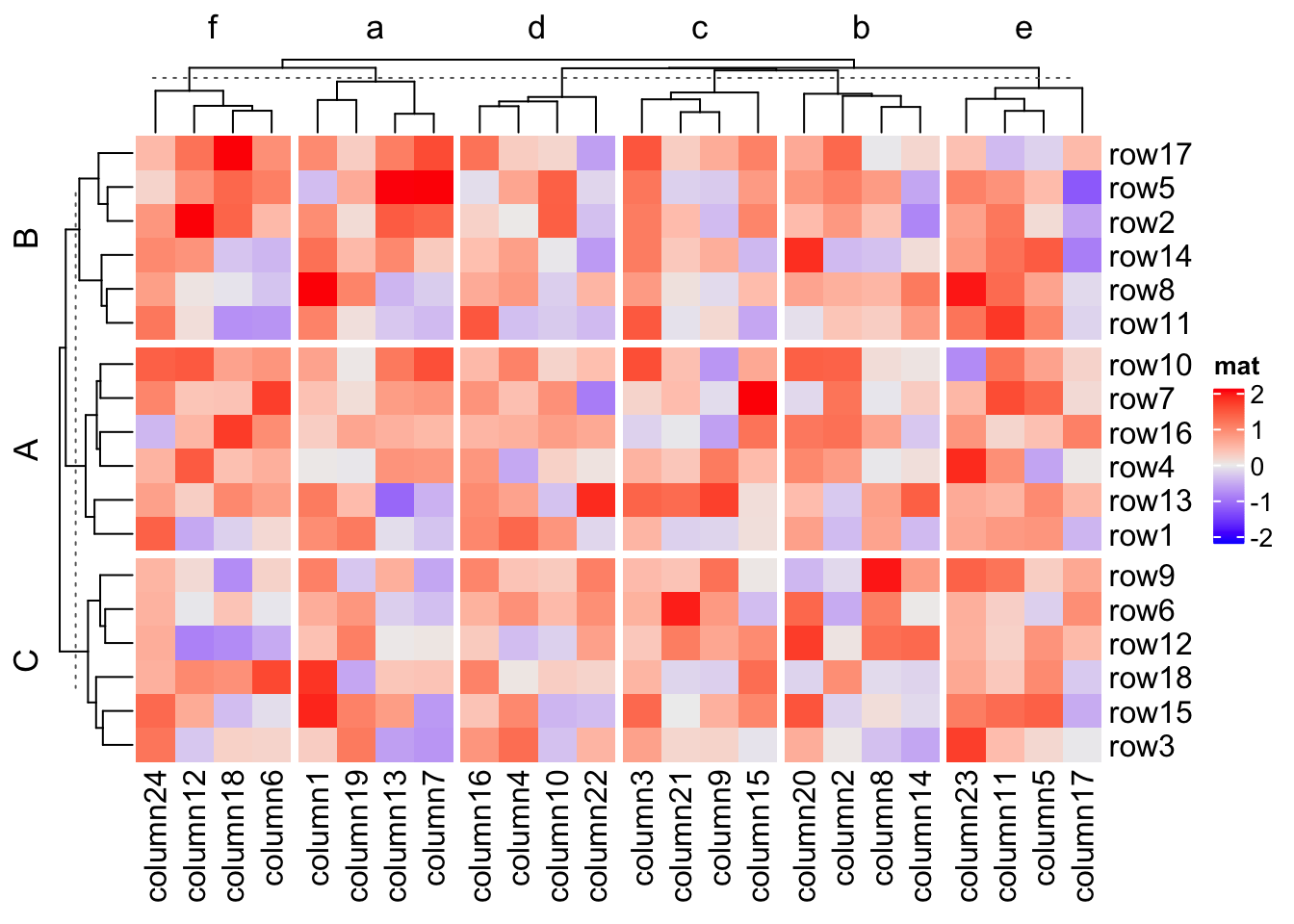# now the order is exactly what we set Heatmap(mat, name = "mat", row_split = factor(rep(LETTERS[1:3], 6), levels = LETTERS[3:1]), column_split = factor(rep(letters[1:6], 4), levels = letters[6:1]), cluster_row_slices = FALSE, cluster_column_slices = FALSE)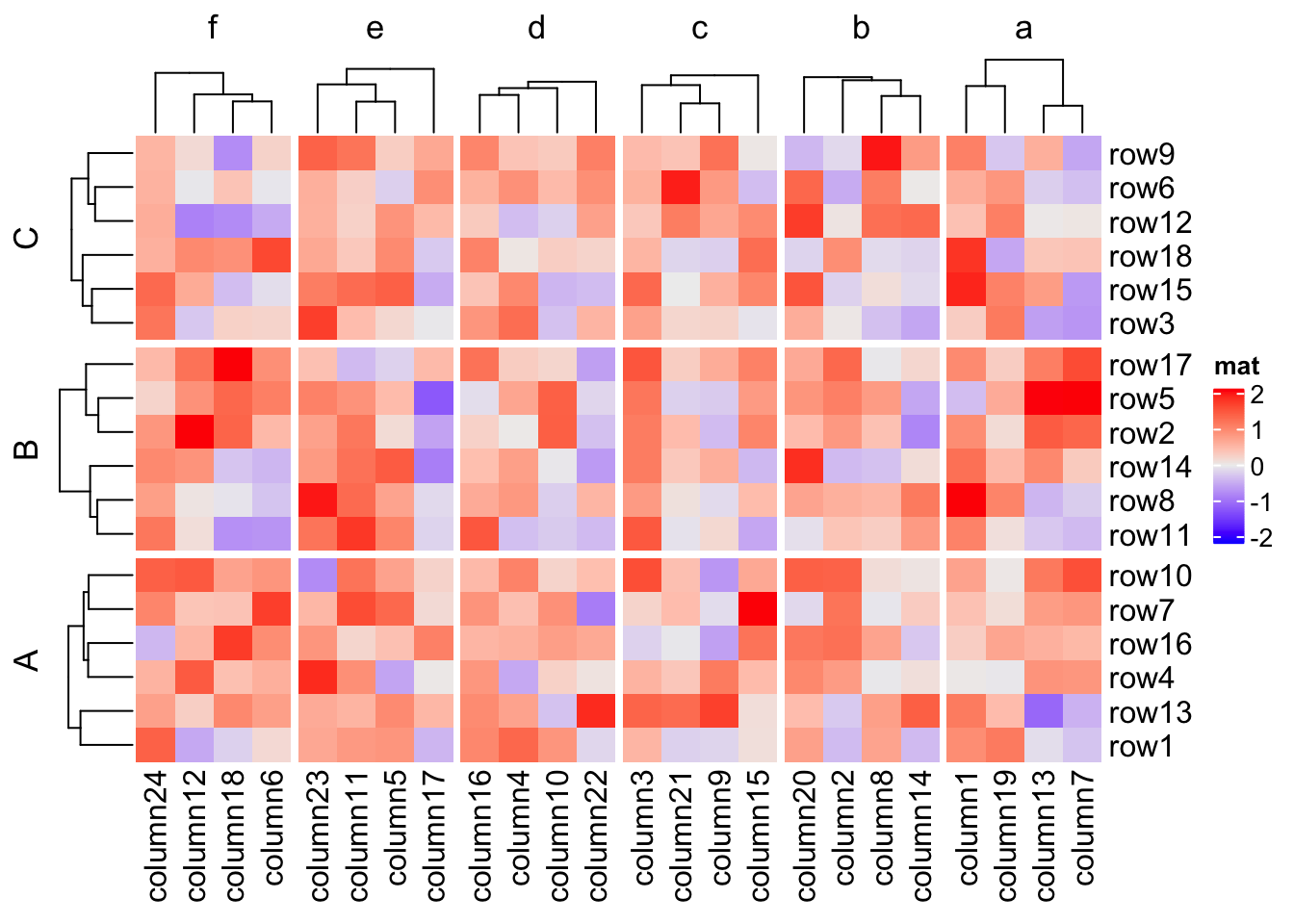### 2.7.5 Titles for splitting When row_split/column_split is set as a single number, there is only one categorical variable, while when row_km/column_km is set and/or row_split/column_split is set as categorical variables, there will be multiple categorical variables. By default, the titles are in a form of "level1,level2,..." which corresponds to every combination of levels in all categorical variables. The titles for splitting can be controlled by “a title template”. ComplexHeatmap supports three types of templates. The first one is by sprintf() where the %s is replaced by the corresponding level. In following example, since all combinations of split are A,C, A,D, B,C and B,D, if row_title is set to %s|%s, the four row titles will be A|C, A|D, B|C, B|D. split = data.frame(rep(c("A", "B"), 9), rep(c("C", "D"), each = 9)) Heatmap(mat, name = "mat", row_split = split, row_title = "%s|%s")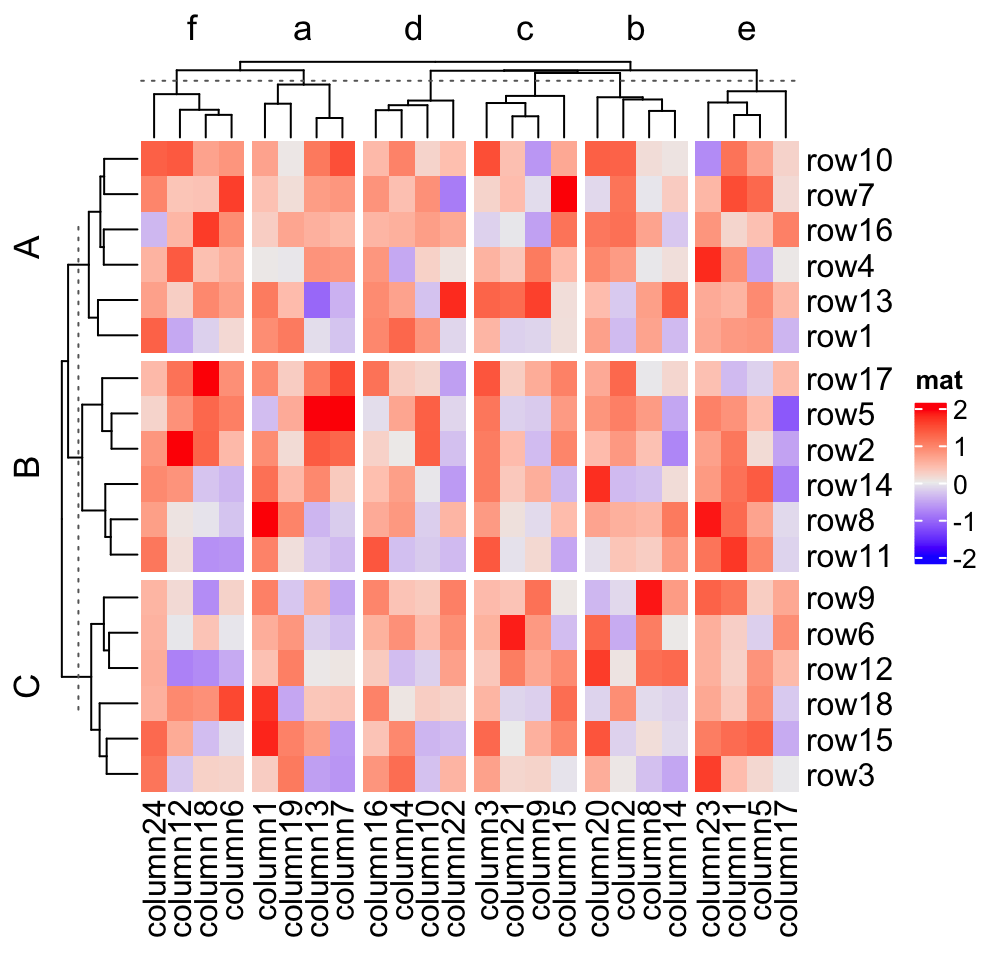For the sprintf() template, you can only put the levels which are A,B,C,D in the title, and C,D is always after A,B. However, when making the heatmap, you might want to put more meaningful text instead of the internal levels. Once you know how to correspond the text to the level, you can add it by following two template methods. In the following two template methods, special marks are used to mark the R code which is executable (it is called variable interpolation where the code is extracted and executed and the returned value in put back to the string). There are two types of template marks @{} and {}. The first one is from GetoptLong package which should already be installed when you install the ComplexHeatmap package and the second one is from glue package which you need to install first. There is an internal variable x you should use when you use the latter two templates. x is just a simple vector which contains current category levels (e.g. c("A", "C")). # We only run the code for the first heatmap map = c("A" = "aaa", "B" = "bbb", "C" = "333", "D" = "444") Heatmap(mat, name = "mat", row_split = split, row_title = "@{map[ x ]}|@{map[ x ]}") Heatmap(mat, name = "mat", row_split = split, row_title = "{map[ x ]}|{map[ x ]}")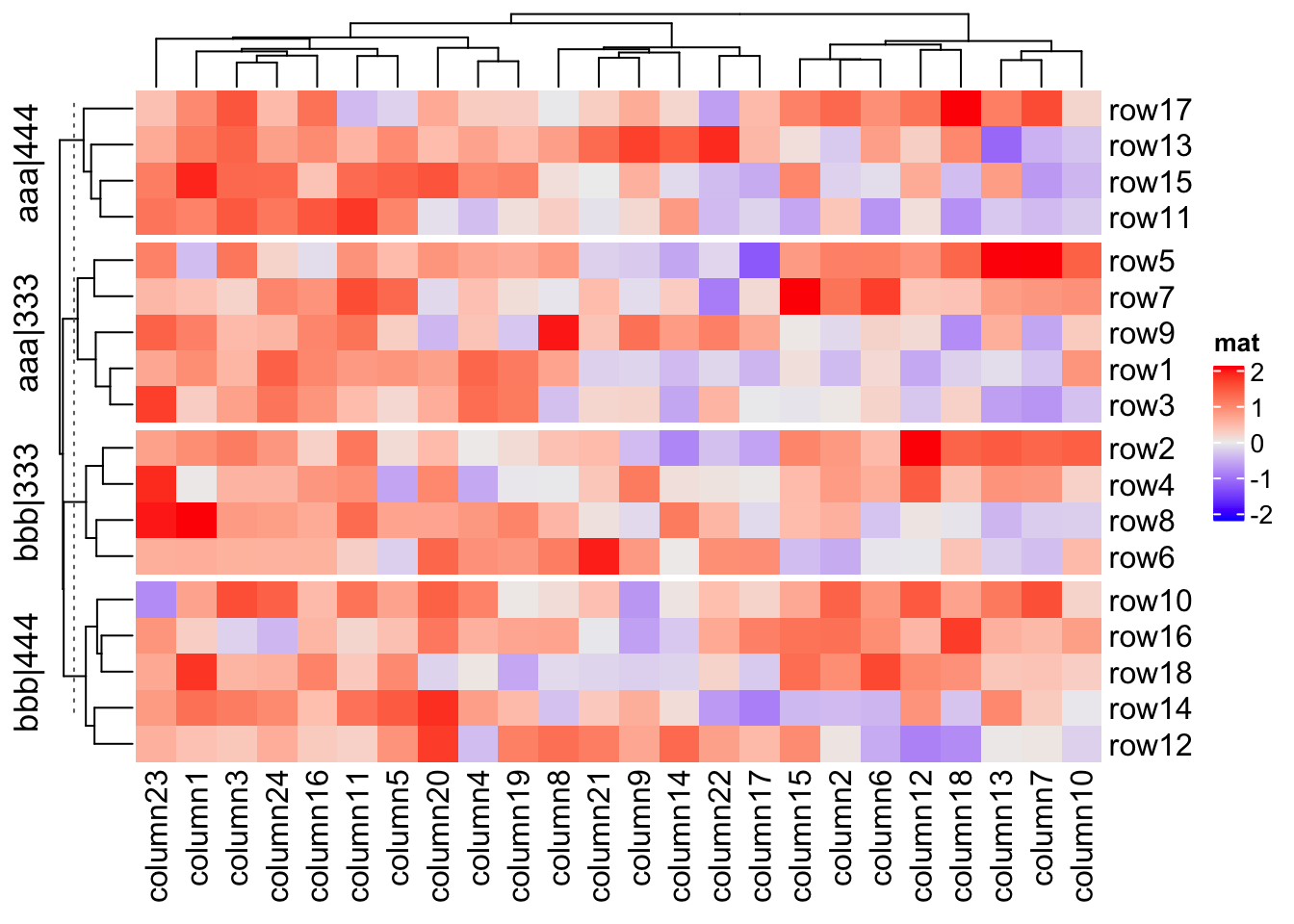The row title is rotated by default, you can set row_title_rot = 0 to make it horizontal: Heatmap(mat, name = "mat", row_split = split, row_title = "%s|%s", row_title_rot = 0)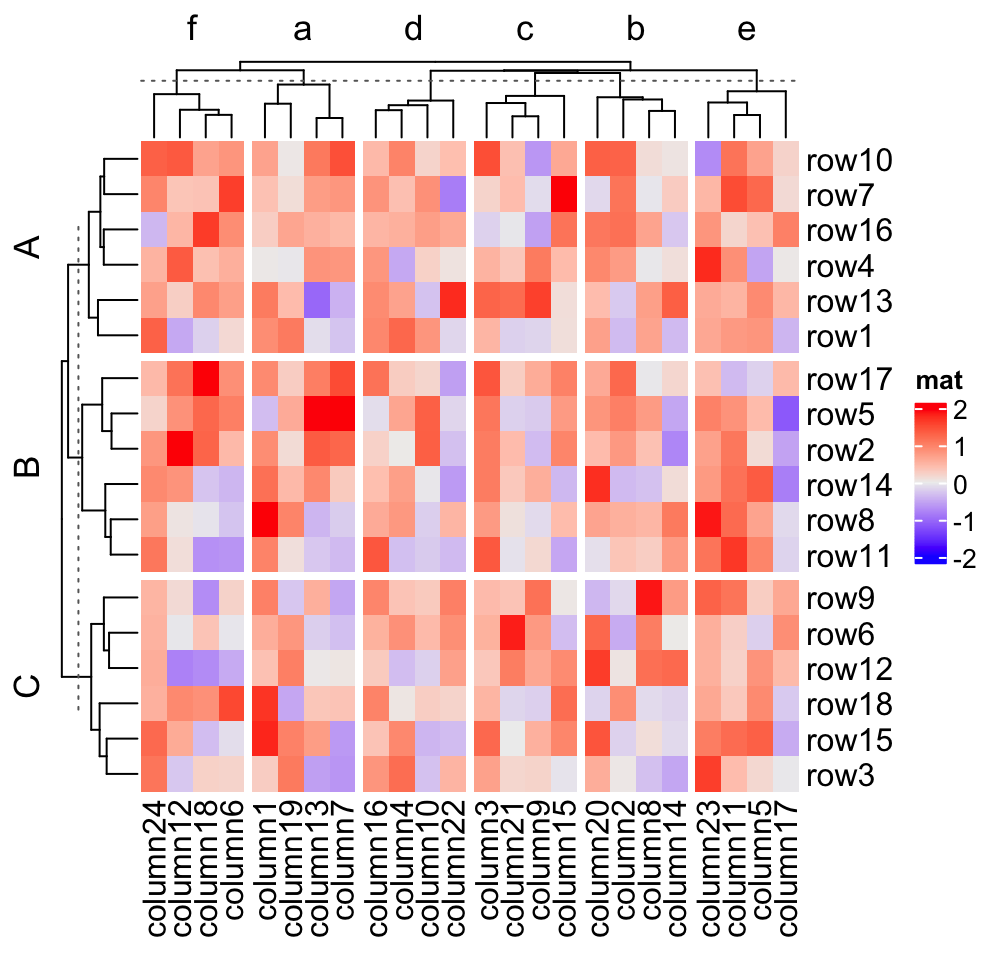When row_split/column_split is set as a number, you can also use template to adjust the titles for slices. Heatmap(mat, name = "mat", row_split = 2, row_title = "cluster_%s")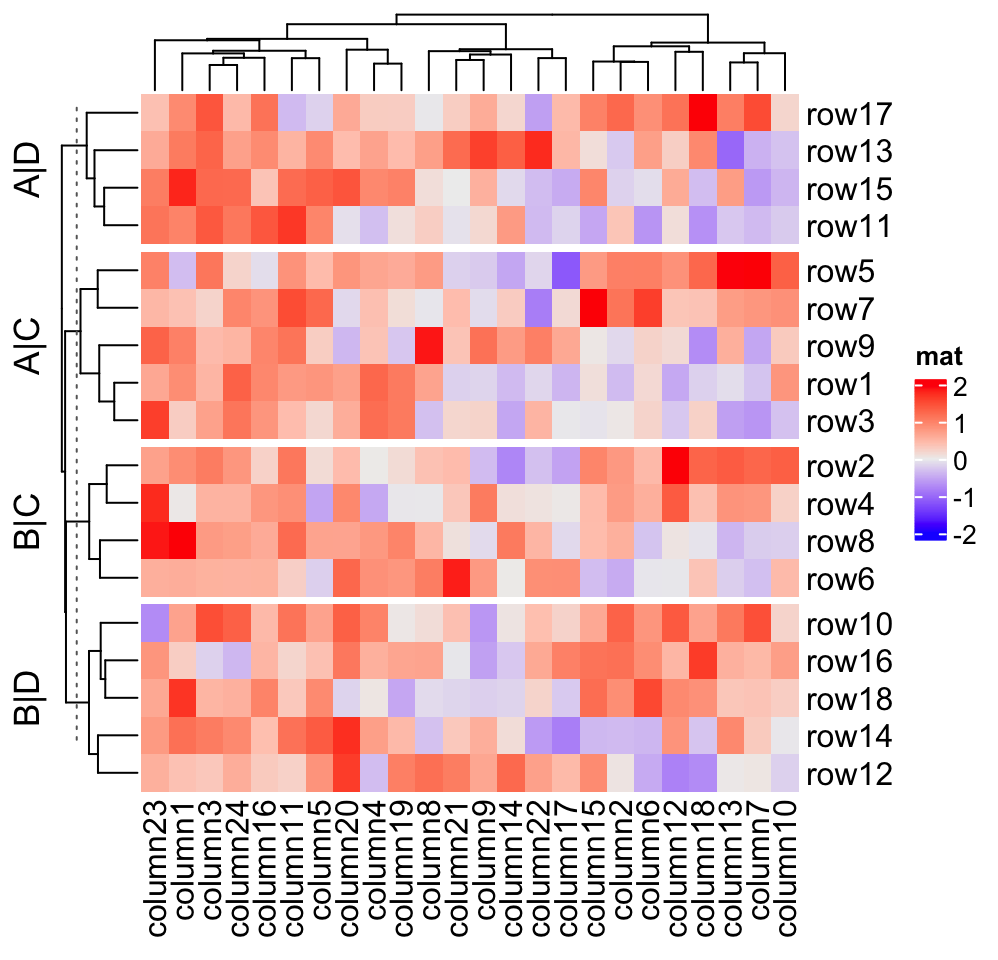If you know the final number of row slices, you can directly set a vector of titles to row_title. Be careful the number of row slices is not always identical to nlevel_1*nlevel_2*.... Heatmap(mat, name = "mat", row_split = split, row_title = c("top_slice", "middle_top_slice", "middle_bottom_slice", "bottom_slice"), row_title_rot = 0)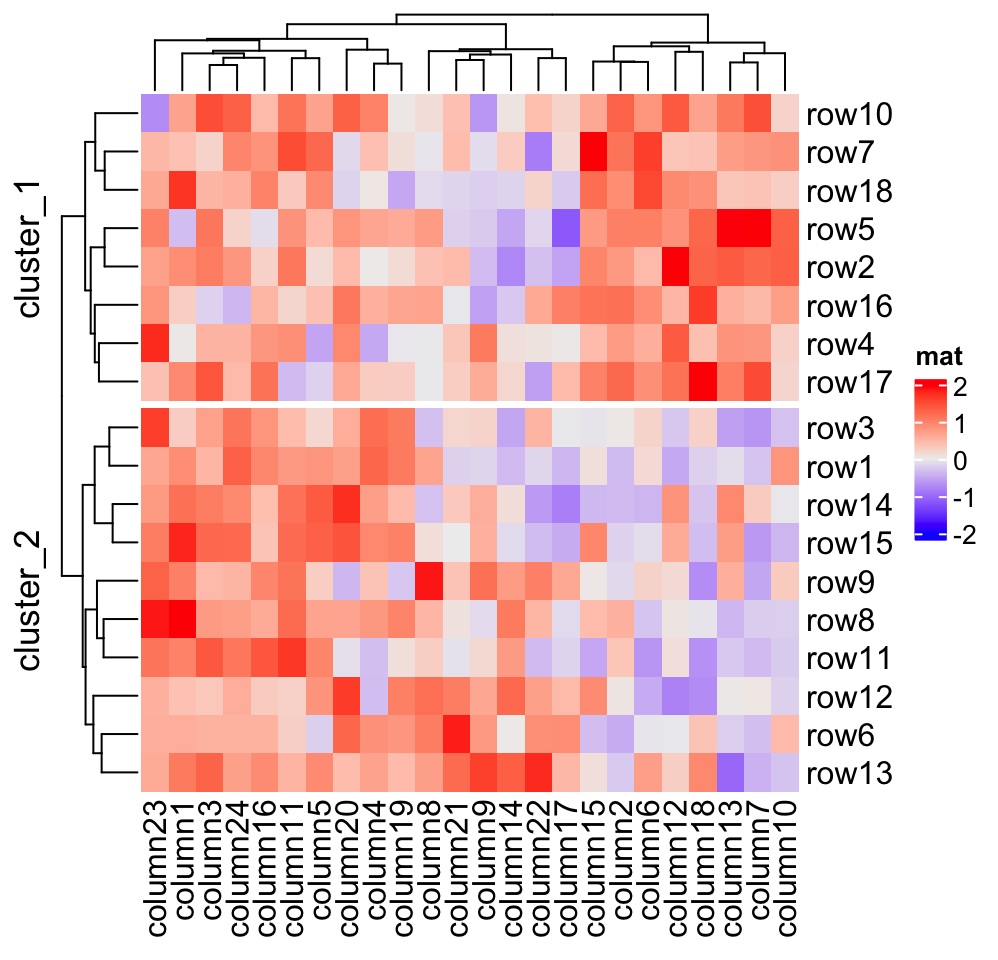If the length of row_title is specified as a single string, it will be like a single title for all slices. Heatmap(mat, name = "mat", row_split = split, row_title = "there are four slices")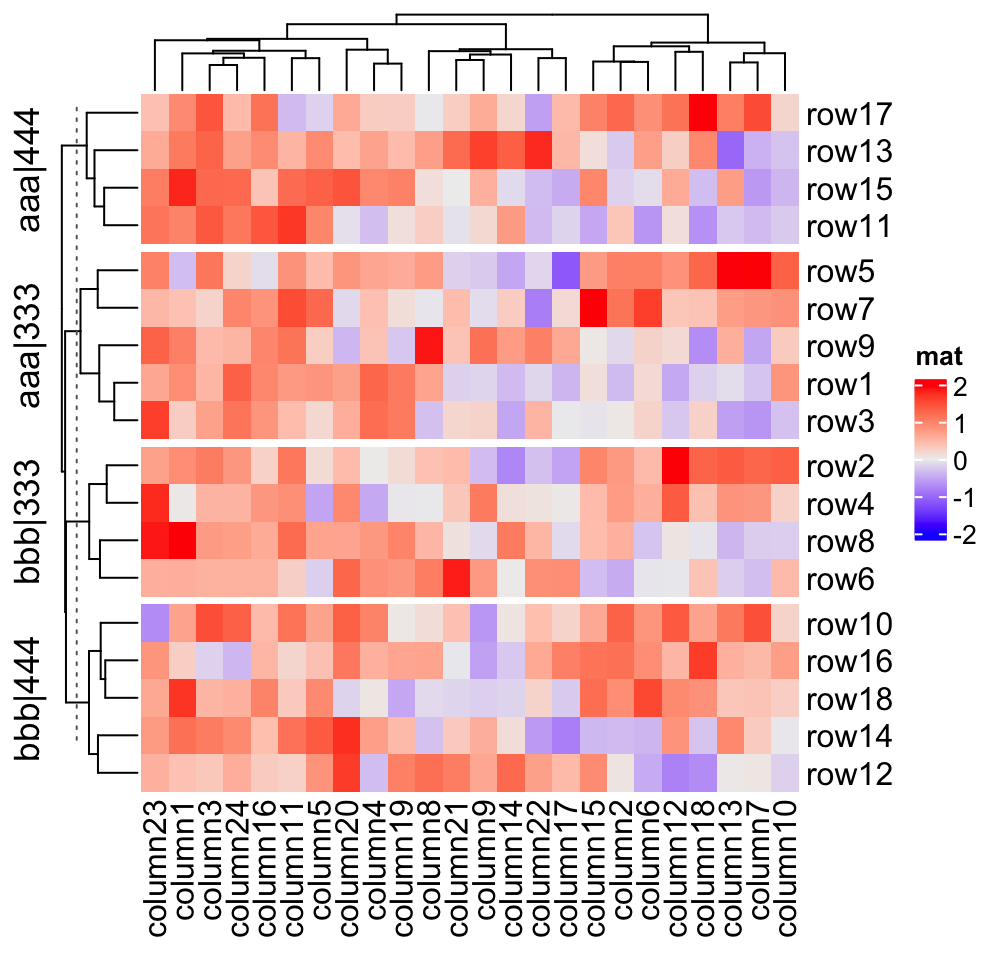If you still want titles for each slice, but also a global title, you can do as follows. ht = Heatmap(mat, name = "mat", row_split = split, row_title = "%s|%s") draw(ht, row_title = "I am a row title")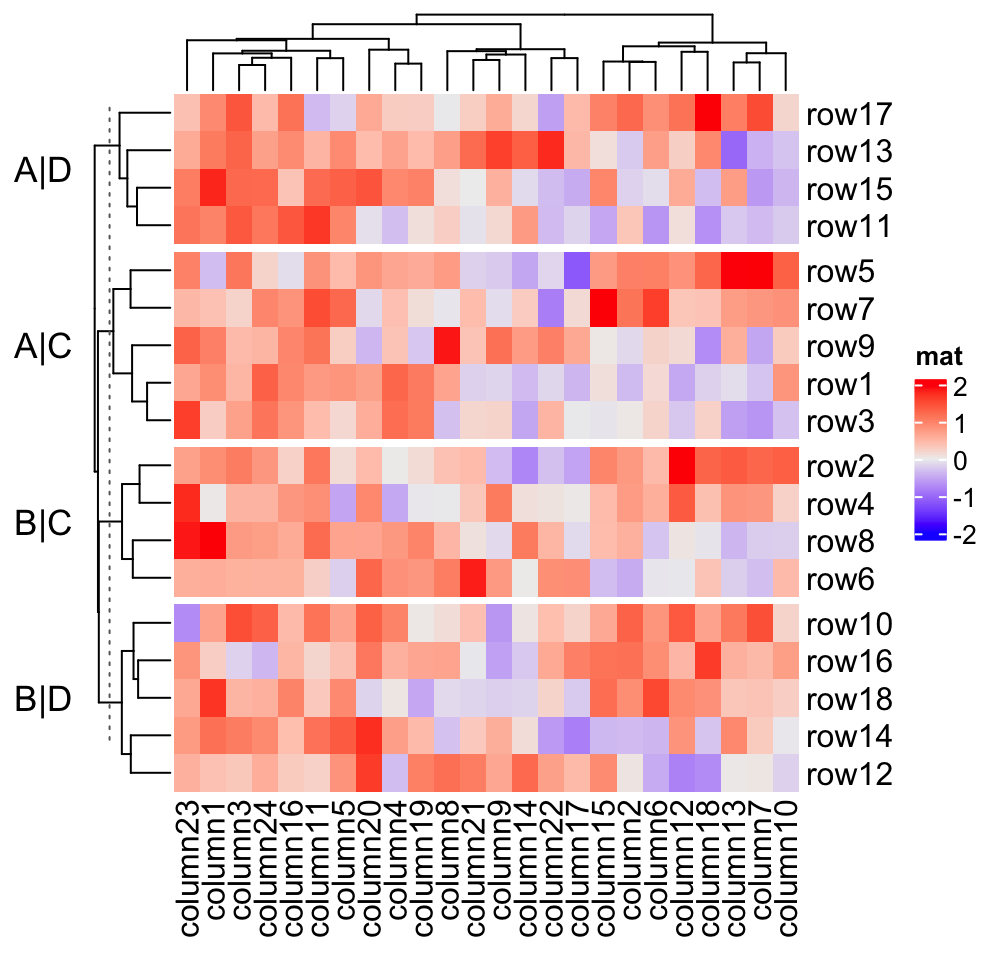Actually the row_title used in draw() function is the row title of the heatmap list, although in the example there is only one heatmap. The draw() function and the heatmap list will be introduced in Chapter 4. If row_title is set to NULL, no row title will be drawn. Heatmap(mat, name = "mat", row_split = split, row_title = NULL)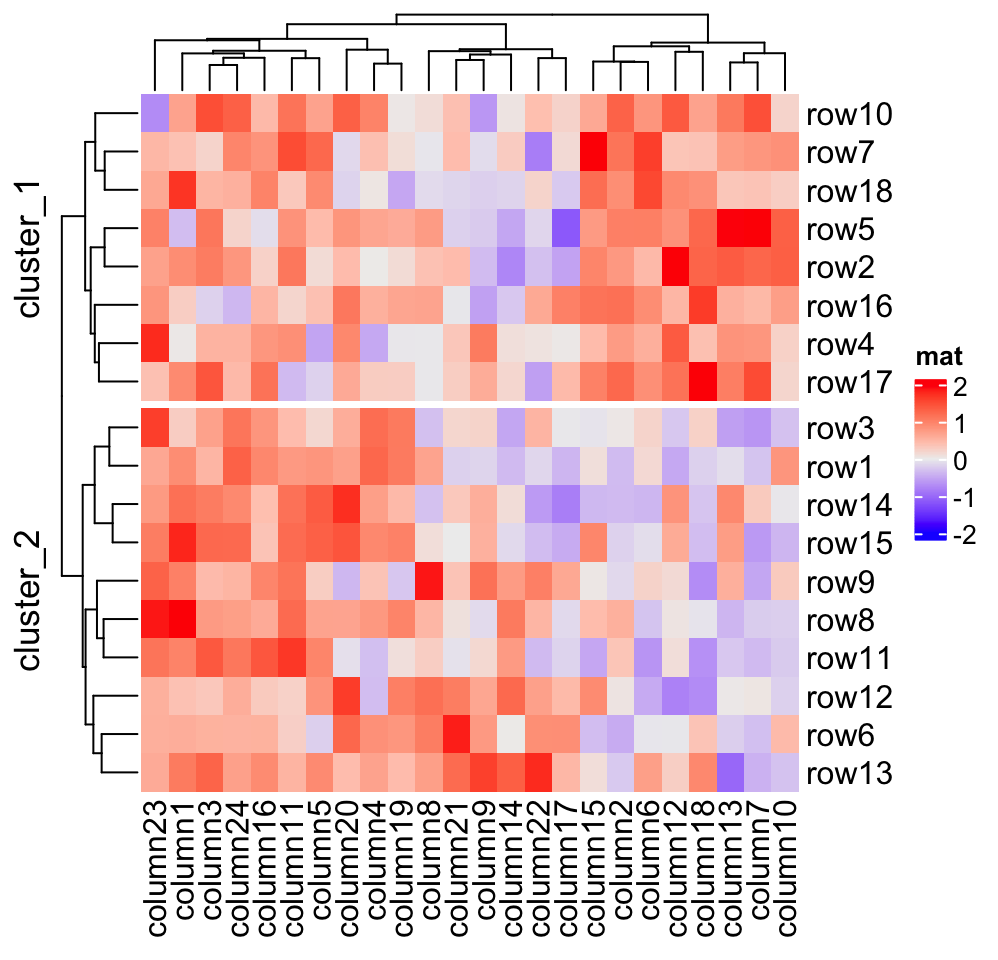All these rules also work for column titles for slices. ### 2.7.6 Graphic parameters for splitting When splitting is applied on rows/columns, graphic parameters for row/column title and row/column names can be specified as same length as number of slices. Heatmap(mat, name = "mat", row_km = 2, row_title_gp = gpar(col = c("red", "blue"), font = 1:2), row_names_gp = gpar(col = c("green", "orange"), fontsize = c(10, 14)), column_km = 3, column_title_gp = gpar(fill = c("red", "blue", "green"), font = 1:3), column_names_gp = gpar(col = c("green", "orange", "purple"), fontsize = c(10, 14, 8)))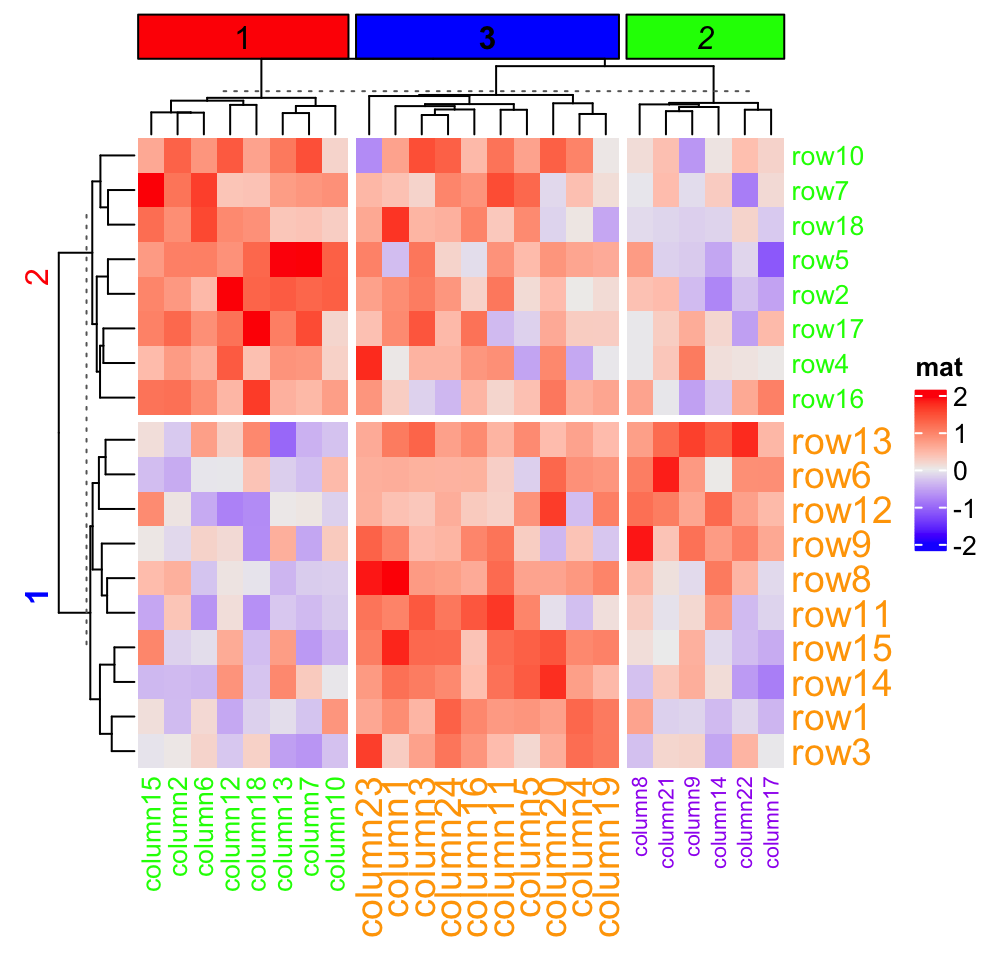### 2.7.7 Gaps between slices The space of gaps between row/column slices can be controlled by row_gap/column_gap. The value can be a single unit or a vector of units. Heatmap(mat, name = "mat", row_km = 3, row_gap = unit(5, "mm"))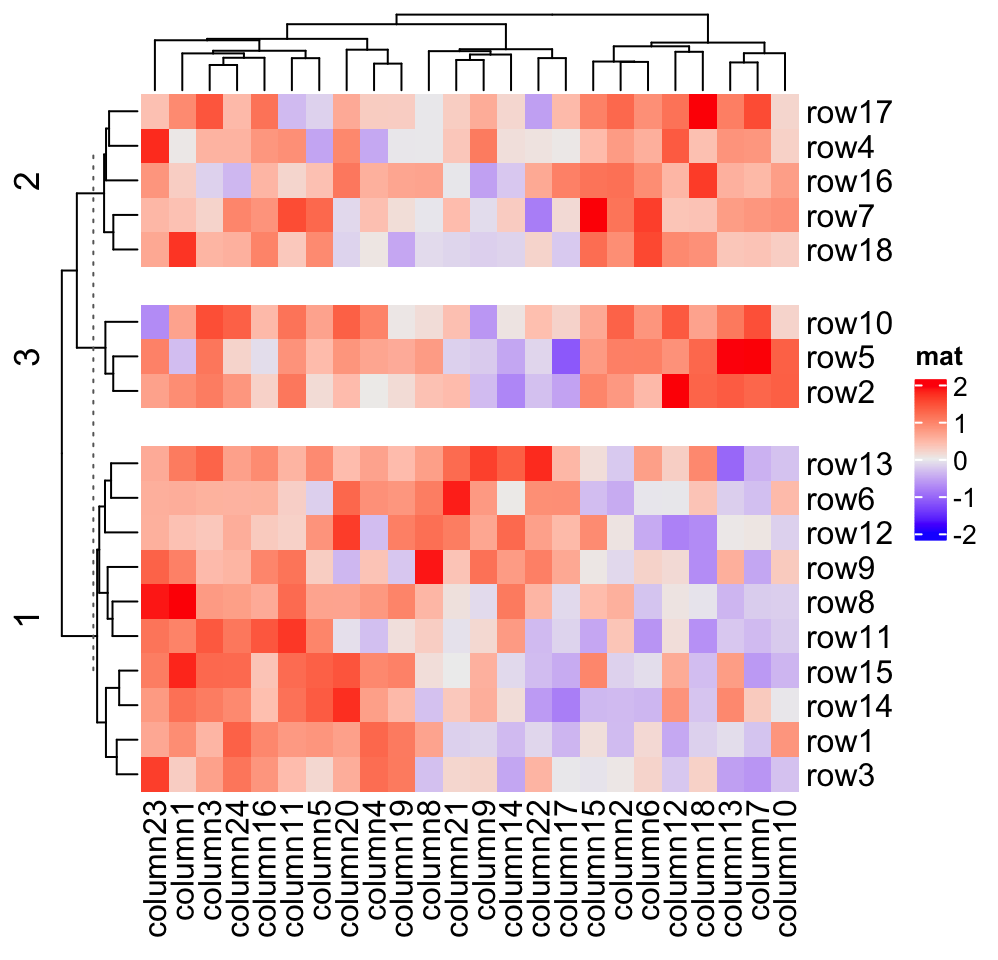Heatmap(mat, name = "mat", row_km = 3, row_gap = unit(c(2, 4), "mm"))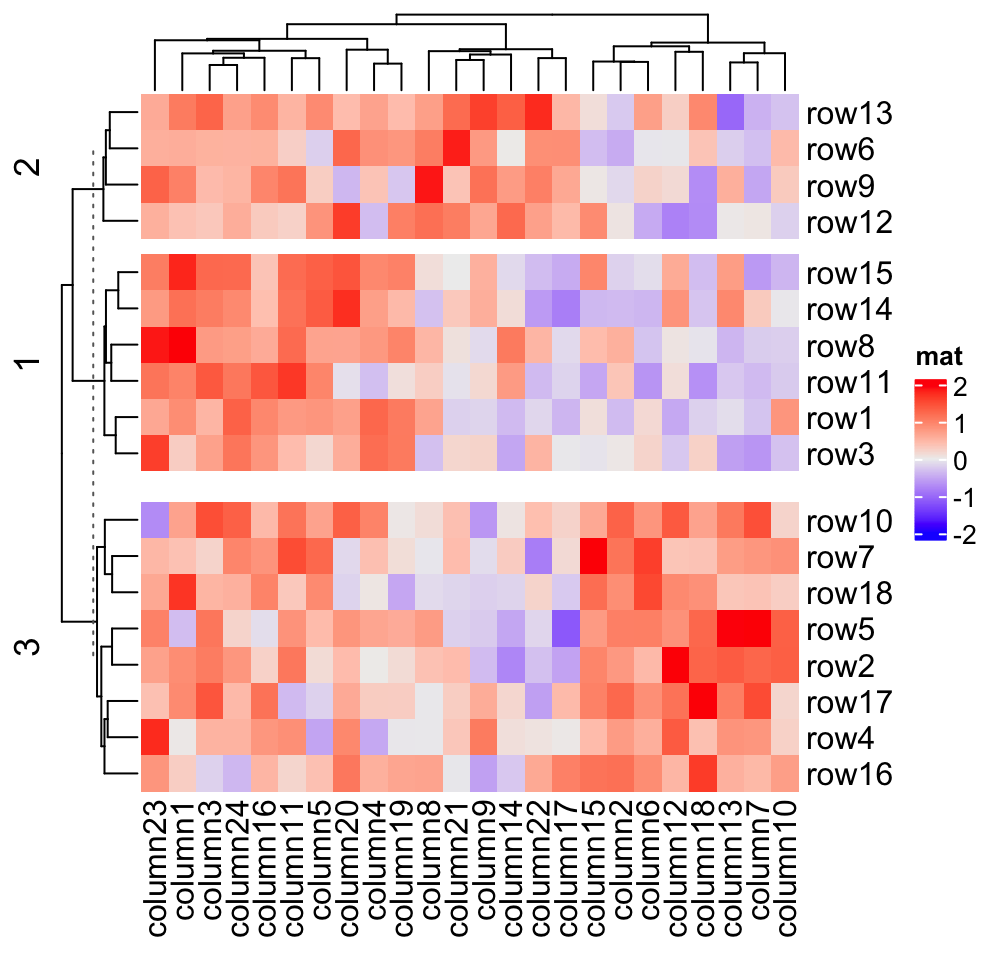Heatmap(mat, name = "mat", row_km = 3, row_gap = unit(c(2, 4), "mm"), column_km = 3, column_gap = unit(c(2, 4), "mm"))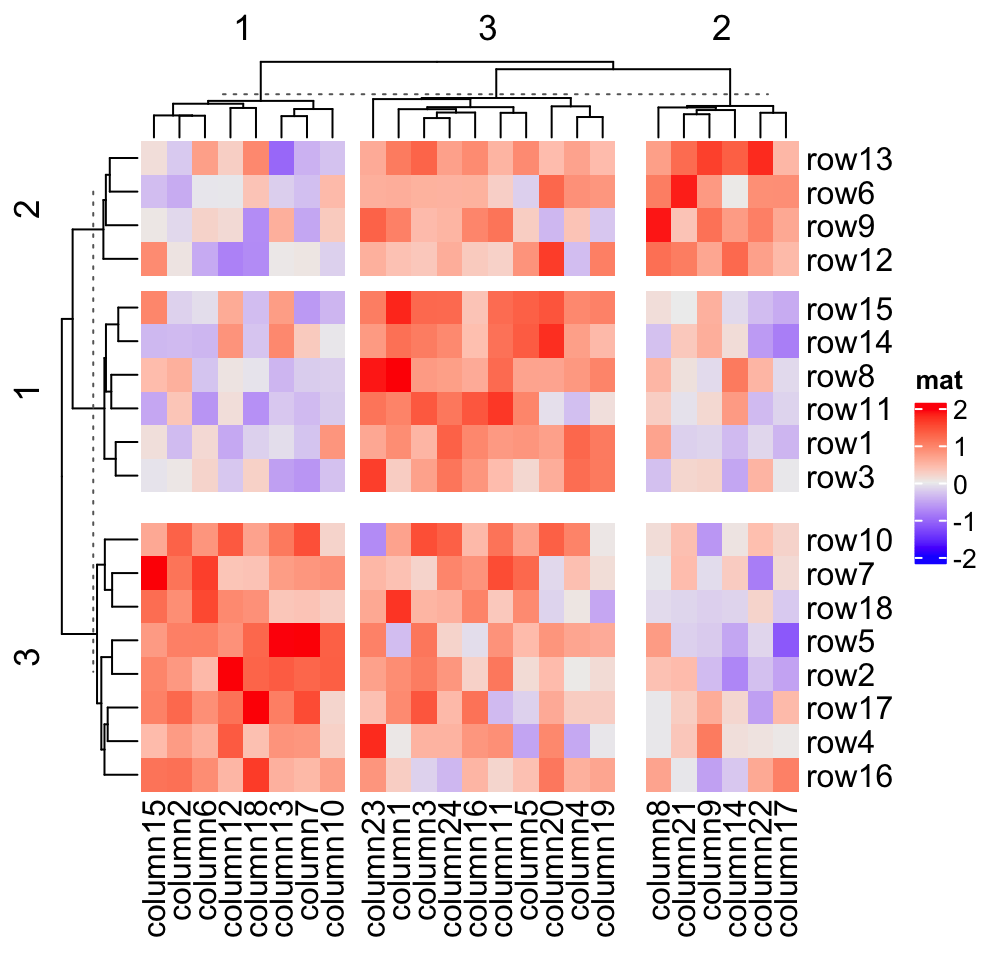When heatmap border is added by setting border = TRUE, the border of every slice is added. Heatmap(mat, name = "mat", row_km = 2, column_km = 3, border = TRUE)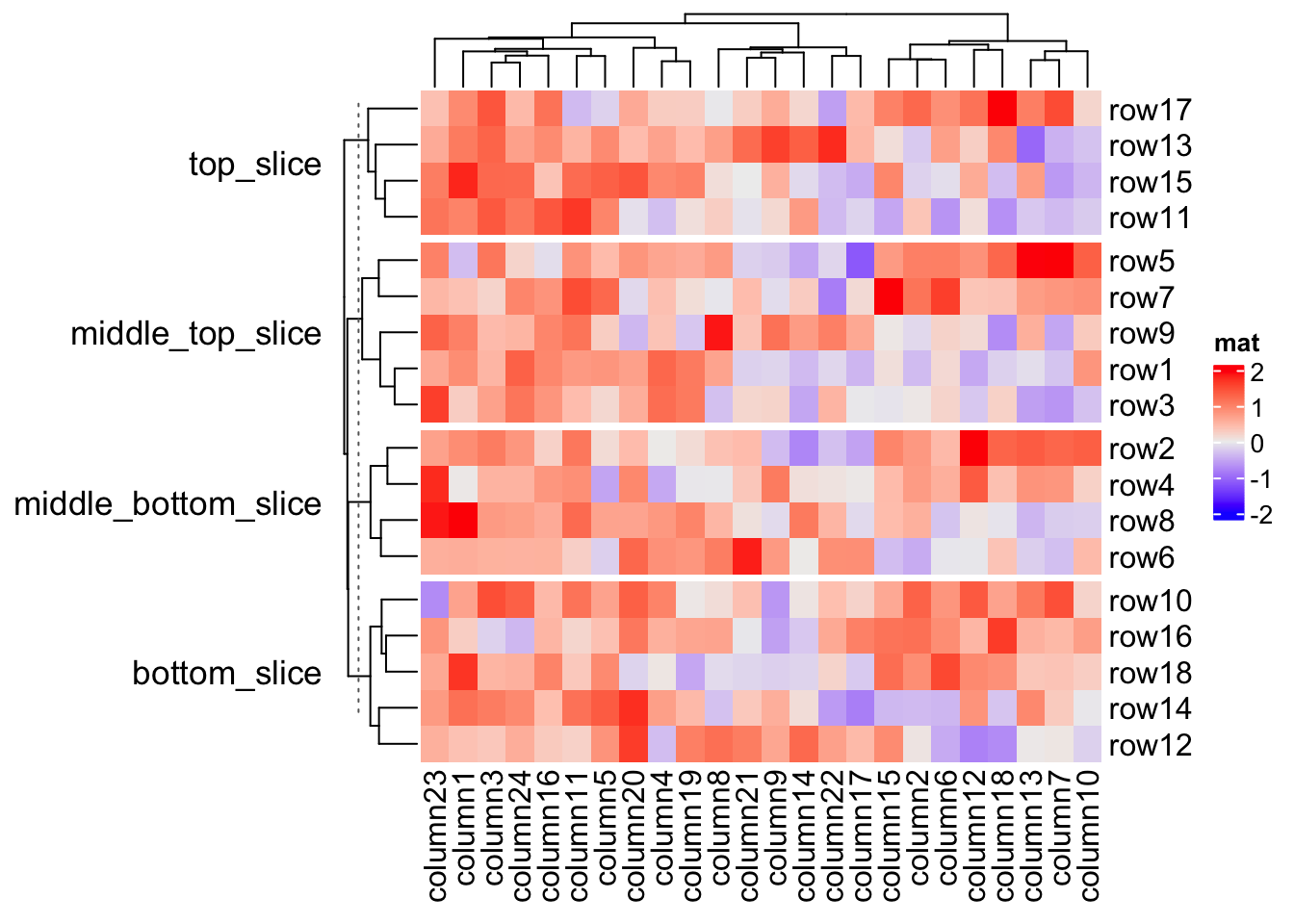If you set gap size to zero, the heatmap will look like it is partitioned by vertical and horizontal lines. Heatmap(mat, name = "mat", row_km = 2, column_km = 3, row_gap = unit(0, "mm"), column_gap = unit(0, "mm"), border = TRUE)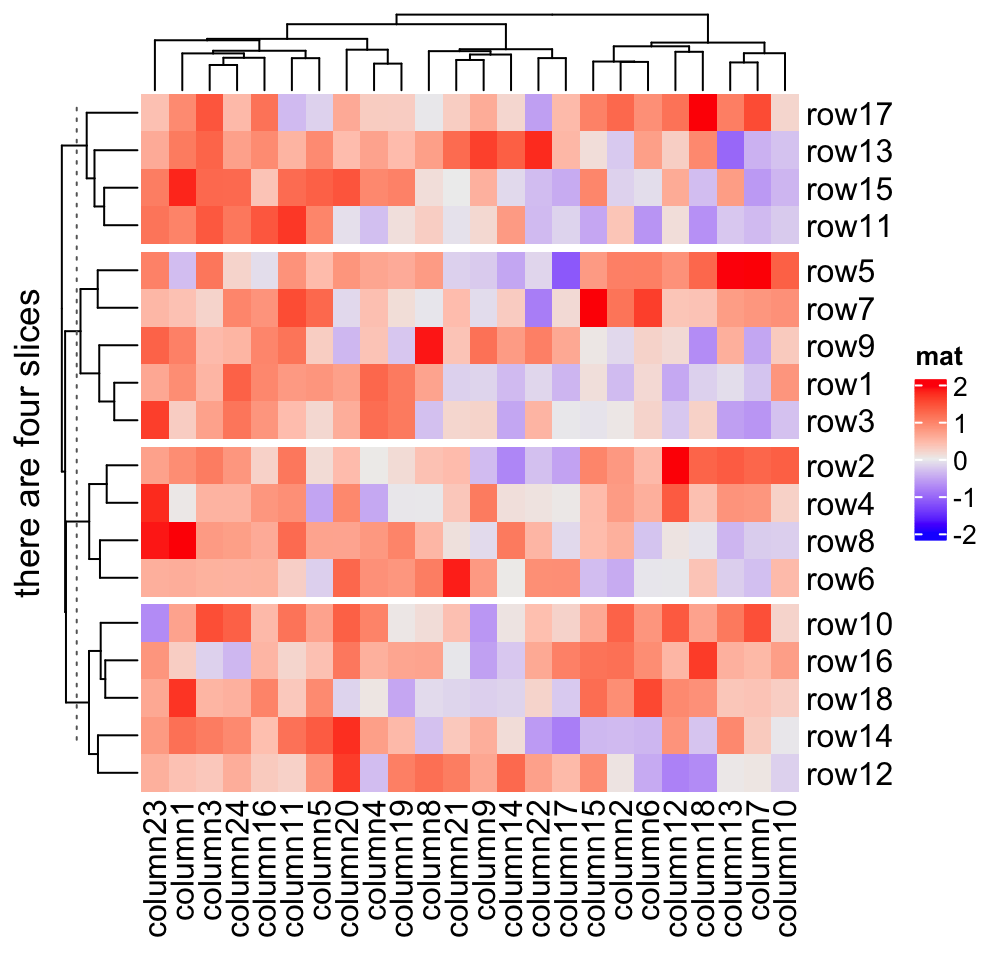### 2.7.8 Split heatmap annotations When the heatmap is split, all the heatmap components are split accordingly. Following gives you a simple example and the heatmap annotation will be introduced in Chapter 3. Heatmap(mat, name = "mat", row_km = 2, column_km = 3, top_annotation = HeatmapAnnotation(foo1 = 1:24, bar1 = anno_points(runif(24))), right_annotation = rowAnnotation(foo2 = 18:1, bar2 = anno_barplot(runif(18))) )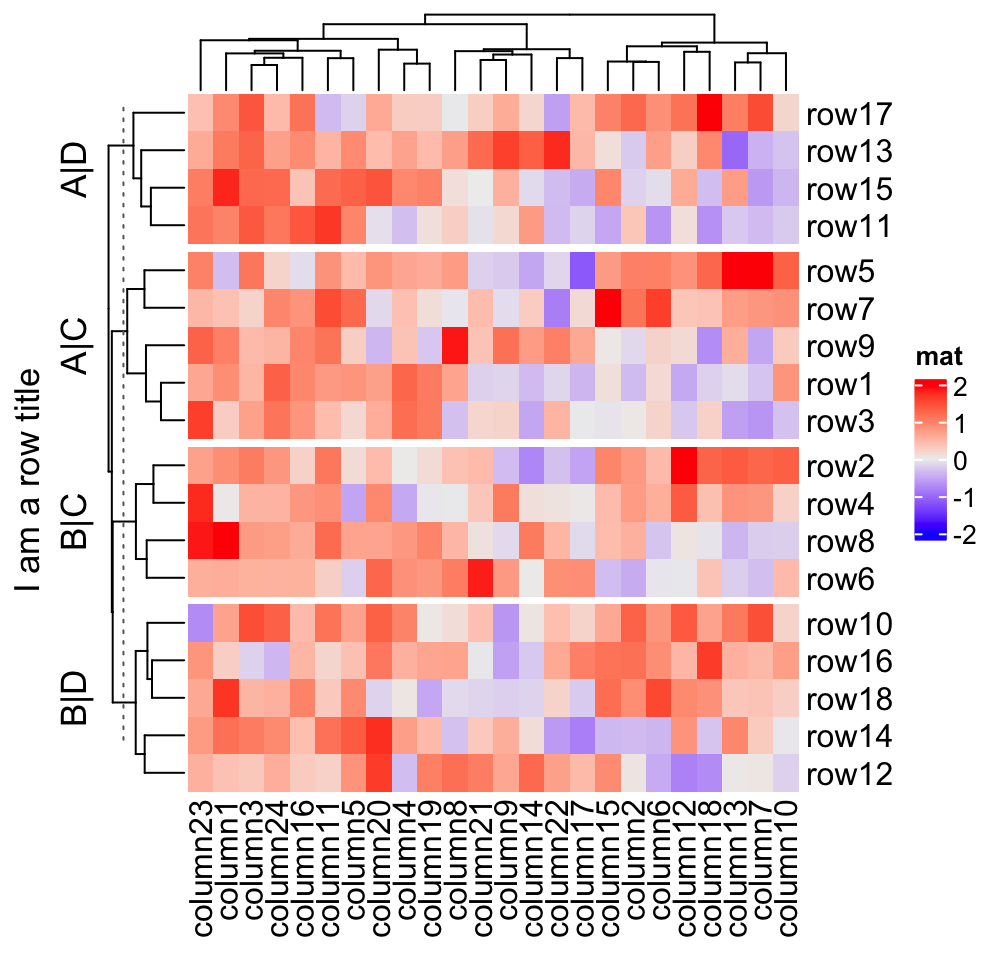## 2.8 Heatmap as raster image Saving plots in PDF format is kind like best parctice to preserve the quality of the plots. However, when there are too many rows (say, > 10000), the output PDF file would be huge and it takes long time and memory to read the whole plot. On the other hand, details of the huge matrix will not be seen in limited size of PDF file. Rendering heatmaps (the heatmap body) as raster images will effectively reduce the file size while the plot looks exactly the same for your screen or if you print it out. In Heatmap() function, there are four options which control how to generate the raster image: use_raster, raster_device, raster_quality, raster_device_param. You can choose graphic device (png, jpeg and tiff) by raster_device, control the quality of the raster image by raster_quality, and pass further parameters for a specific device by raster_device_param. If raster_quality is set to 1, internally, a PNG (if raster_device is set to png) file is generated with the same physical size as the heatmap body and refit into the heatmap body as a raster image. The png file generated has the size of raster_quality*width and raster_quality*height. So a larger raster_quality value gives you a better reservation of the original resolution. # code only for demonstration Heatmap(mat, use_raster = TRUE, raster_quality = 2) In Complexheatmap, use_raster is by default turned on if the number of rows or columns is more than 2000. Following example compares the PDF file size with raster image by different devices. set.seed(123) mat2 = matrix(rnorm(10000*100), ncol = 100) pdf("heatmap.pdf", width = 8, height = 8) Heatmap(mat2, cluster_rows = FALSE, cluster_columns = FALSE, use_raster = FALSE) dev.off() pdf("heatmap_raster_by_png.pdf", width = 8, height = 8) Heatmap(mat2, cluster_rows = FALSE, cluster_columns = FALSE, use_raster = TRUE, raster_device = "png") dev.off() pdf("heatmap_raster_by_jpeg.pdf", width = 8, height = 8) Heatmap(mat2, cluster_rows = FALSE, cluster_columns = FALSE, use_raster = TRUE, raster_device = "jpeg") dev.off() pdf("heatmap_raster_by_tiff.pdf", width = 8, height = 8) Heatmap(mat2, cluster_rows = FALSE, cluster_columns = FALSE, use_raster = TRUE, raster_device = "tiff") dev.off() pdf("heatmap_raster_by_CairoPNG.pdf", width = 8, height = 8) Heatmap(mat2, cluster_rows = FALSE, cluster_columns = FALSE, use_raster = TRUE, raster_device = "CairoPNG") dev.off() pdf("heatmap_raster_by_CairoJPEG.pdf", width = 8, height = 8) Heatmap(mat2, cluster_rows = FALSE, cluster_columns = FALSE, use_raster = TRUE, raster_device = "CairoJPEG") dev.off() all_files = c("heatmap.pdf", "heatmap_raster_by_png.pdf", "heatmap_raster_by_jpeg.pdf", "heatmap_raster_by_tiff.pdf", "heatmap_raster_by_CairoPNG.pdf", "heatmap_raster_by_CairoJPEG.pdf") fs = file.size(all_files) names(fs) = all_files sapply(fs, function(x) paste(round(x/1024), "KB")) ## heatmap.pdf heatmap_raster_by_png.pdf ## "6583 KB" "374 KB" ## heatmap_raster_by_jpeg.pdf heatmap_raster_by_tiff.pdf ## "2845 KB" "374 KB" ## heatmap_raster_by_CairoPNG.pdf heatmap_raster_by_CairoJPEG.pdf ## "307 KB" "2975 KB" ## 2.9 Customize the heatmap body The heatmap body can be self-defined to add more types of graphics. By default the heatmap body is composed by a matrix of small rectangles (it might be called grids in other parts of this documentation, but let’s call it “cells” here) with different filled colors. However, it is also possible to add more graphics or symbols as additional layers on the heatmap. There are two arguments cell_fun and layer_fun which both should be user-defined functions. ### 2.9.1 cell_fun cell_fun draws in each cell repeatedly, which is internally executed in two nested for loops, while layer_fun is the vectorized version of cell_fun. cell_fun is easier to understand but layer_fun is much faster to execute and more customizable. cell_fun expects a function with 7 arguments (the argument names can be different from following, but the order must be the same), which are: • j: column index in the matrix. Column index corresponds to the x-direction in the viewport, that’s why j is put as the first argument. • i: row index in the matrix. • x: x coordinate of middle point of the cell which is measured in the viewport of the heatmap body. • y: y coordinate of middle point of the cell which is measured in the viewport of the heatmap body. • width: width of the cell. The value is unit(1/ncol(sub_mat), "npc") where sub_mat correspond to the sub-matrix by row splitting and column splitting. • height: height of the cell. The value is unit(1/nrow(sub_mat), "npc"). • fill: color of the cell. The values for the seven arguments are automatically sent to the function when executed in each cell. The most common use is to add values in the matrix onto the heatmap: small_mat = mat[1:9, 1:9] col_fun = colorRamp2(c(-2, 0, 2), c("green", "white", "red")) Heatmap(small_mat, name = "mat", col = col_fun, cell_fun = function(j, i, x, y, width, height, fill) { grid.text(sprintf("%.1f", small_mat[i, j]), x, y, gp = gpar(fontsize = 10)) })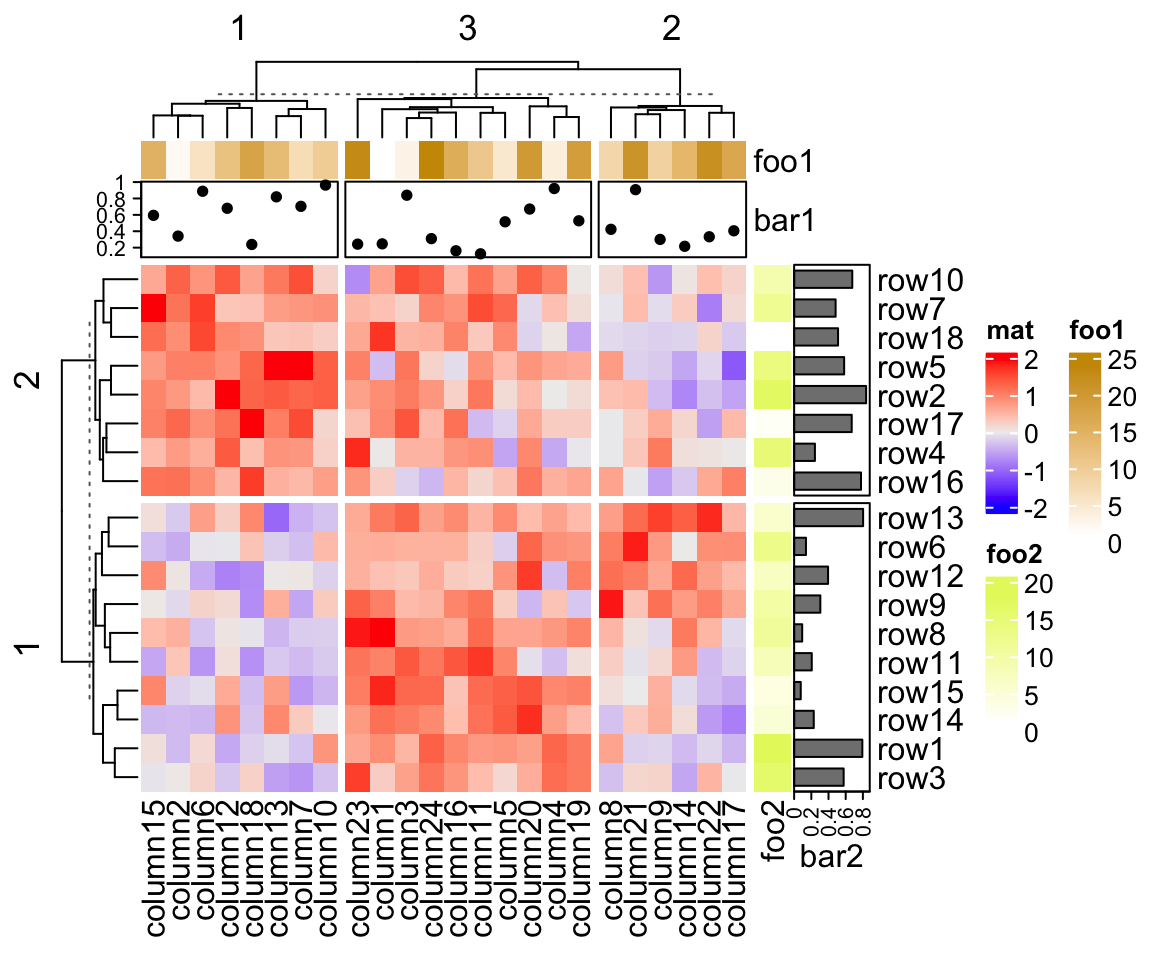and we can also choose only to add text for the cells with positive values: Heatmap(small_mat, name = "mat", col = col_fun, cell_fun = function(j, i, x, y, width, height, fill) { if(small_mat[i, j] > 0) grid.text(sprintf("%.1f", small_mat[i, j]), x, y, gp = gpar(fontsize = 10)) })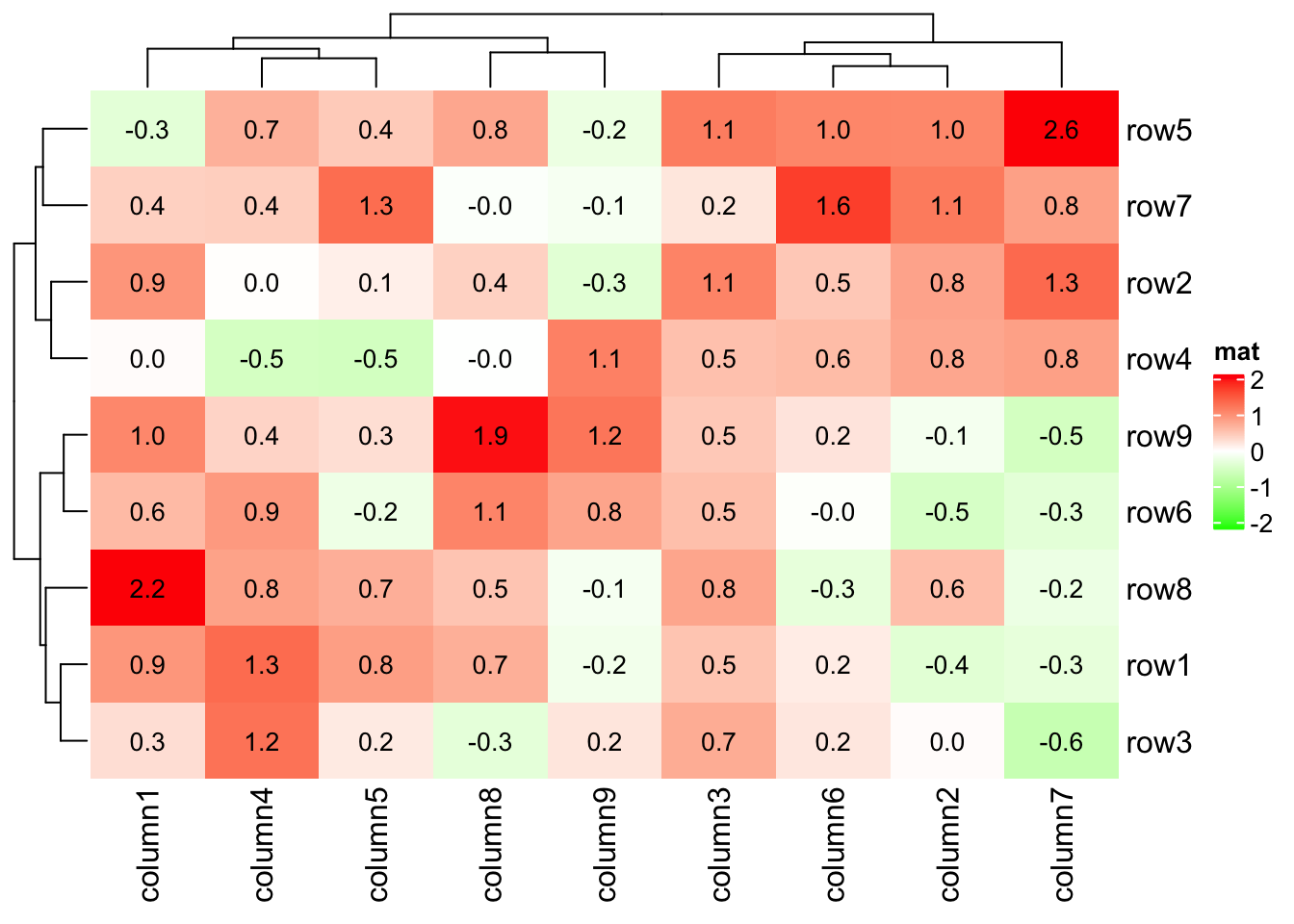You can split the heatmap without doing anything extra to cell_fun: Heatmap(small_mat, name = "mat", col = col_fun, row_km = 2, column_km = 2, cell_fun = function(j, i, x, y, width, height, fill) { grid.text(sprintf("%.1f", small_mat[i, j]), x, y, gp = gpar(fontsize = 10)) })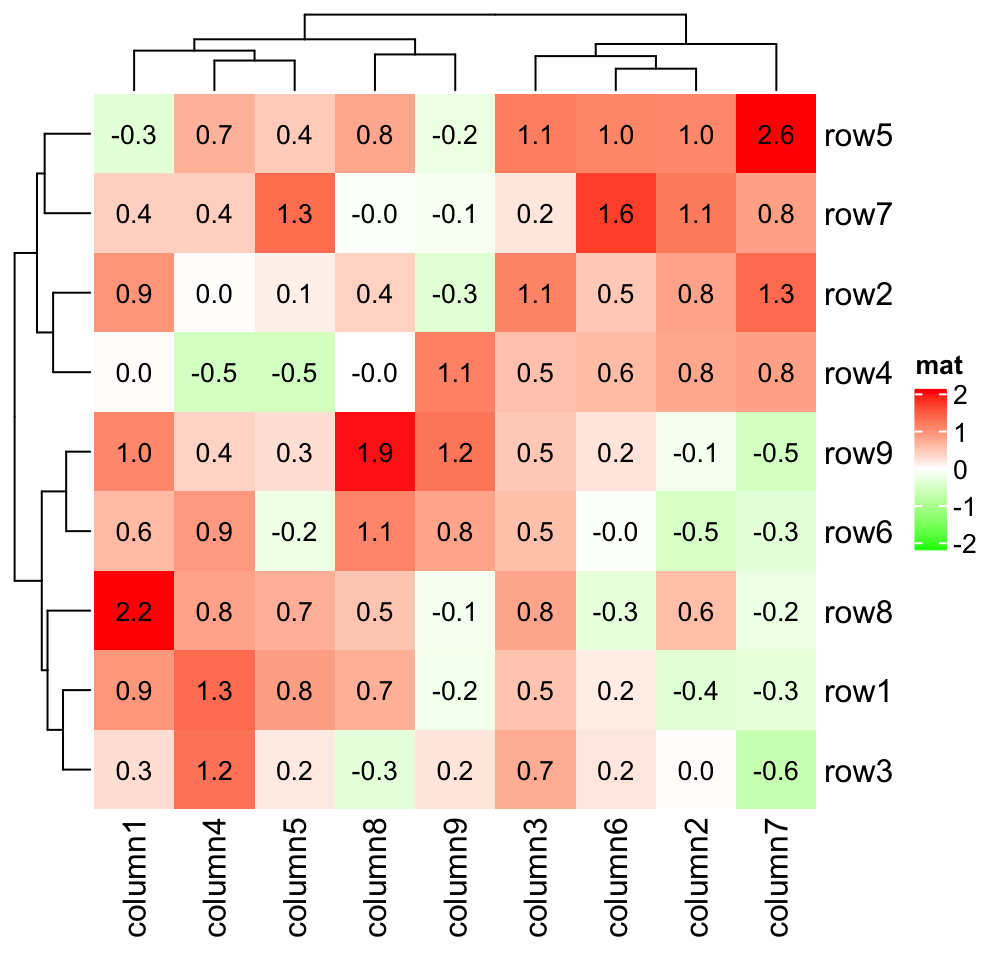In following example, we make a heatmap which shows correlation matrix similar as the corrplot package: cor_mat = cor(small_mat) od = hclust(dist(cor_mat))$order
cor_mat = cor_mat[od, od]
nm = rownames(cor_mat)
col_fun = circlize::colorRamp2(c(-1, 0, 1), c("green", "white", "red"))
# col = col_fun here is used to generate the legend
Heatmap(cor_mat, name = "correlation", col = col_fun, rect_gp = gpar(type = "none"),
cell_fun = function(j, i, x, y, width, height, fill) {
grid.rect(x = x, y = y, width = width, height = height,
gp = gpar(col = "grey", fill = NA))
if(i == j) {
grid.text(nm[i], x = x, y = y)
} else if(i > j) {
grid.circle(x = x, y = y, r = abs(cor_mat[i, j])/2 * min(unit.c(width, height)),
gp = gpar(fill = col_fun(cor_mat[i, j]), col = NA))
} else {
grid.text(sprintf("%.1f", cor_mat[i, j]), x, y, gp = gpar(fontsize = 10))
}
}, cluster_rows = FALSE, cluster_columns = FALSE,
show_row_names = FALSE, show_column_names = FALSE)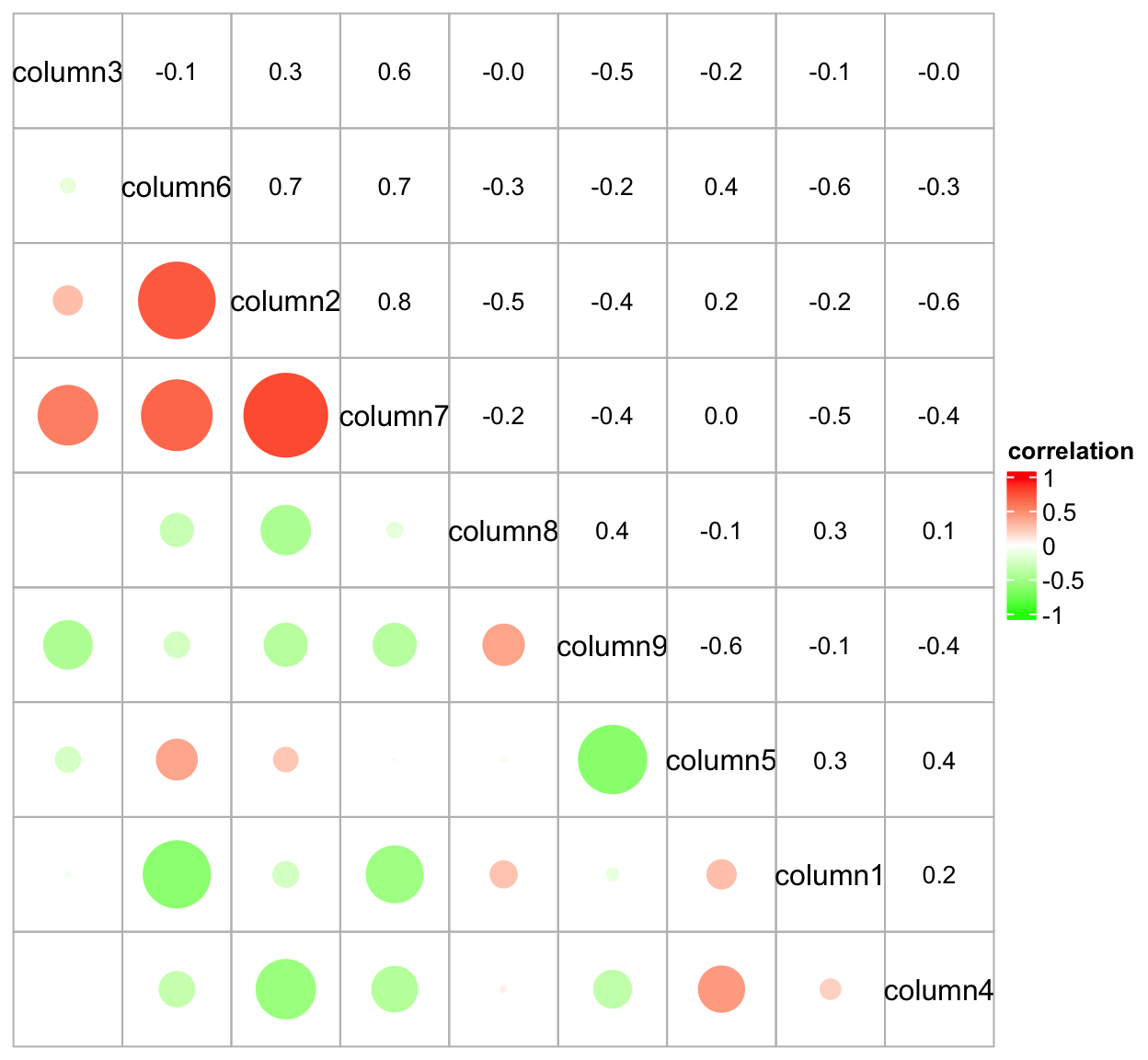As you may see in previous plot, when setting the non-standard parameter rect_gp = gpar(type = "none"), the clustering is performed but nothing is drawn on the heatmap body.

One last example is to visualize a GO game. The input data takes records of moves in the game.

str = "B[cp];W[pq];B[dc];W[qd];B[eq];W[od];B[de];W[jc];B[qk];W[qn]
;B[qh];W[ck];B[ci];W[cn];B[hc];W[je];B[jq];W[df];B[ee];W[cf]
;B[eb];W[db];B[ec];W[lq];B[nq];W[jp];B[iq];W[kq];B[pp];W[op]
;B[po];W[oq];B[rp];W[ql];B[oo];W[no];B[pl];W[pm];B[np];W[qq]
;B[om];W[ol];B[pk];W[qp];B[on];W[rm];B[mo];W[nr];B[rl];W[rk]
;B[qm];W[dp];B[dq];W[ql];B[or];W[mp];B[nn];W[mq];B[qm];W[bp]
;B[co];W[ql];B[no];W[pr];B[qm];W[dd];B[pn];W[ed];B[bo];W[eg]
;B[ef];W[dg];B[ge];W[gh];B[gf];W[gg];B[ek];W[ig];B[fd];W[en]
;B[bn];W[ip];B[dm];W[ff];B[cb];W[fe];B[hp];W[ho];B[hq];W[el]
;B[dl];W[fk];B[ej];W[fp];B[go];W[hn];B[fo];W[em];B[dn];W[eo]
;B[gp];W[ib];B[gc];W[pg];B[qg];W[ng];B[qc];W[re];B[pf];W[of]
;B[rc];W[ob];B[ph];W[qo];B[rn];W[mi];B[og];W[oe];B[qe];W[rd]
;B[rf];W[pd];B[gm];W[gl];B[fm];W[fl];B[lj];W[mj];B[lk];W[ro]
;B[hl];W[hk];B[ik];W[dk];B[bi];W[di];B[dj];W[dh];B[hj];W[gj]
;B[li];W[lh];B[kh];W[lg];B[jn];W[do];B[cl];W[ij];B[gk];W[bl]
;B[cm];W[hk];B[jk];W[lo];B[hi];W[hm];B[gk];W[bm];B[cn];W[hk]
;B[il];W[cq];B[bq];W[ii];B[sm];W[jo];B[kn];W[fq];B[ep];W[cj]
;B[bk];W[er];B[cr];W[gr];B[gk];W[fj];B[ko];W[kp];B[hr];W[jr]
;B[nh];W[mh];B[mk];W[bb];B[da];W[jh];B[ic];W[id];B[hb];W[jb]
;B[oj];W[fn];B[fs];W[fr];B[gs];W[es];B[hs];W[gn];B[kr];W[is]
;B[dr];W[fi];B[bj];W[hd];B[gd];W[ln];B[lm];W[oi];B[oh];W[ni]
;B[pi];W[ki];B[kj];W[ji];B[so];W[rq];B[if];W[jf];B[hh];W[hf]
;B[he];W[ie];B[hg];W[ba];B[ca];W[sp];B[im];W[sn];B[rm];W[pe]
;B[qf];W[if];B[hk];W[nj];B[nk];W[lr];B[mn];W[af];B[ag];W[ch]
;B[bh];W[lp];B[ia];W[ja];B[ha];W[sf];B[sg];W[se];B[eh];W[fh]
;B[in];W[ih];B[ae];W[so];B[af]"

We convert it into a matrix:

str = gsub("\\n", "", str)
step = strsplit(str, ";")[]
type = gsub("(B|W).*", "\\1", step)
row = gsub("(B|W)\$(.).\$", "\\2", step)
column = gsub("(B|W)\$.(.)\$", "\\2", step)

go_mat = matrix(nrow = 19, ncol = 19)
rownames(go_mat) = letters[1:19]
colnames(go_mat) = letters[1:19]
for(i in seq_along(row)) {
go_mat[row[i], column[i]] = type[i]
}
go_mat[1:4, 1:4]
##   a   b   c   d
## a NA  NA  NA  "W"
## b "W" "W" "W" "B"
## c "B" "B" "W" "W"
## d "B" "W" "B" "W"

Black and white stones are put based on the values in the matrix:

Heatmap(go_mat, name = "go", rect_gp = gpar(type = "none"),
cell_fun = function(j, i, x, y, w, h, col) {
grid.rect(x, y, w, h, gp = gpar(fill = "#dcb35c", col = NA))
if(i == 1) {
grid.segments(x, y-h*0.5, x, y)
} else if(i == nrow(go_mat)) {
grid.segments(x, y, x, y+h*0.5)
} else {
grid.segments(x, y-h*0.5, x, y+h*0.5)
}
if(j == 1) {
grid.segments(x, y, x+w*0.5, y)
} else if(j == ncol(go_mat)) {
grid.segments(x-w*0.5, y, x, y)
} else {
grid.segments(x-w*0.5, y, x+w*0.5, y)
}

if(i %in% c(4, 10, 16) & j %in% c(4, 10, 16)) {
grid.points(x, y, pch = 16, size = unit(2, "mm"))
}

r = min(unit.c(w, h))*0.45
if(is.na(go_mat[i, j])) {
} else if(go_mat[i, j] == "W") {
grid.circle(x, y, r, gp = gpar(fill = "white", col = "white"))
} else if(go_mat[i, j] == "B") {
grid.circle(x, y, r, gp = gpar(fill = "black", col = "black"))
}
},
col = c("B" = "black", "W" = "white"),
show_row_names = FALSE, show_column_names = FALSE,
column_title = "One famous GO game",
heatmap_legend_param = list(title = "Player", at = c("B", "W"),
labels = c("player1", "player2"), border = "black")
)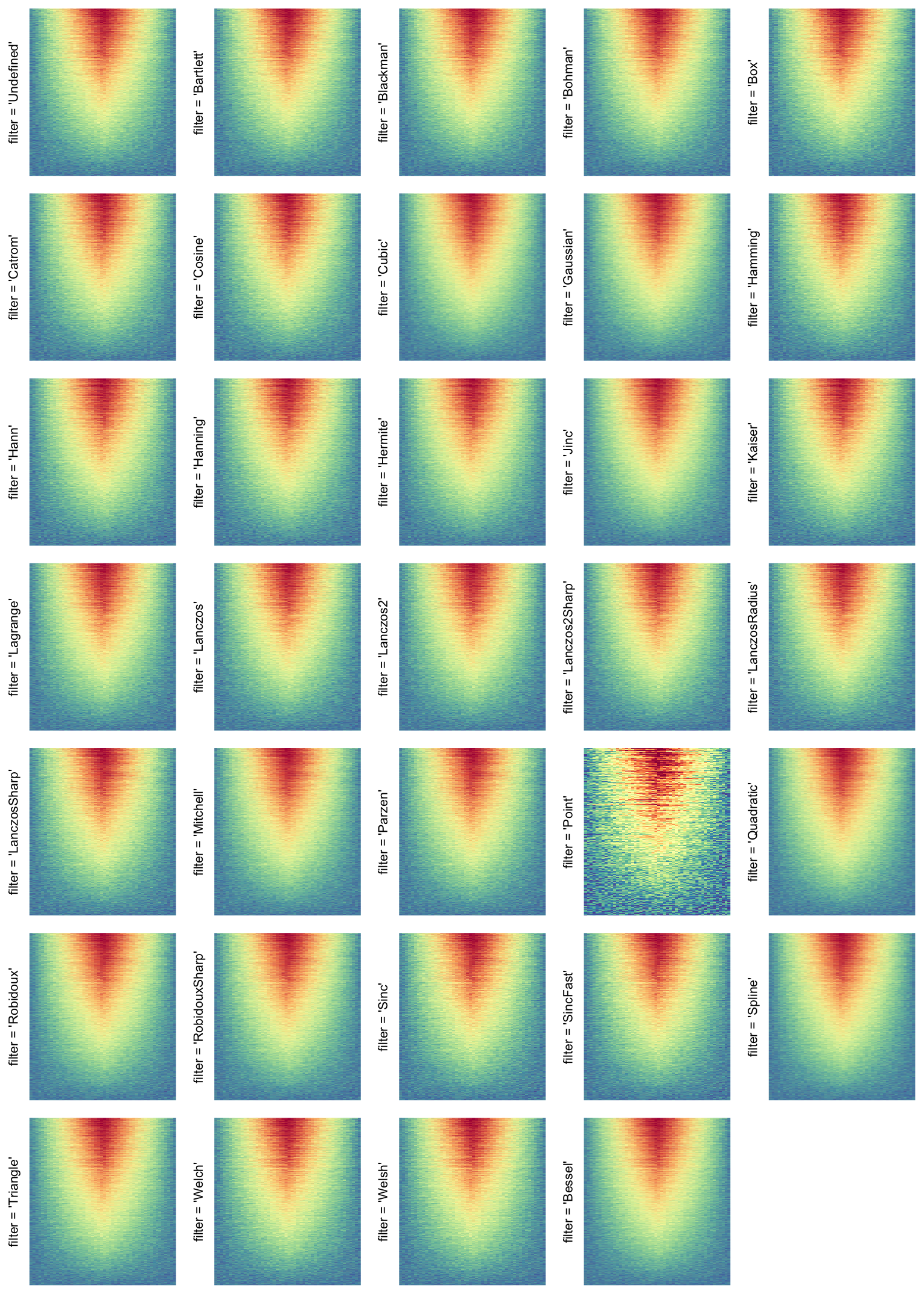### 2.9.2 layer_fun

cell_fun adds graphics cell by cell, while layer_fun adds graphics in a block-wise manner. Similar as cell_fun, layer_fun also needs seven arguments, but they are all in vector form (layer_fun can also have a eighth and ninth arguments which is introduced later this section):

# code only for demonstration
Heatmap(..., layer_fun = function(j, i, x, y, w, h, fill) {...})
# on you can capitalize the arguments to mark they are vectors
Heatmap(..., layer_fun = function(J, I, X, Y, W, H, F) {...})

j and i still contain the column and row indices corresponding to the original matrix, but since now layer_fun applies to a block of cells (or a block of heatmap if the heatmap is split), j and i are vectors for all the cells in the current heatmap slice. Similarlly, x, y, w, h and fill are all vectors corresponding to all cells in the current heatmap slice.

Since j and i now are vectors, to get corresponding values in the matrix, we cannot use the form as mat[j, i] because it gives you a sub-matrix with length(i) rows and length(j) columns. Instead we can use pindex() function from ComplexHeatmap which is like pairwise indexing for a matrix. See follow example:

mfoo = matrix(1:9, nr = 3)
mfoo[1:2, c(1, 3)]
##      [,1] [,2]
## [1,]    1    7
## [2,]    2    8
# but we actually want mfoo[1, 1] and mfoo[2, 3]
pindex(mfoo, 1:2, c(1, 3))
##  1 8

Next example shows the layer_fun version of adding text on heatmap. It’s basically the same as the cell_fun version.

col_fun = colorRamp2(c(-2, 0, 2), c("green", "white", "red"))
Heatmap(small_mat, name = "mat", col = col_fun,
layer_fun = function(j, i, x, y, width, height, fill) {
# since grid.text can also be vectorized
grid.text(sprintf("%.1f", pindex(small_mat, i, j)), x, y, gp = gpar(fontsize = 10))
})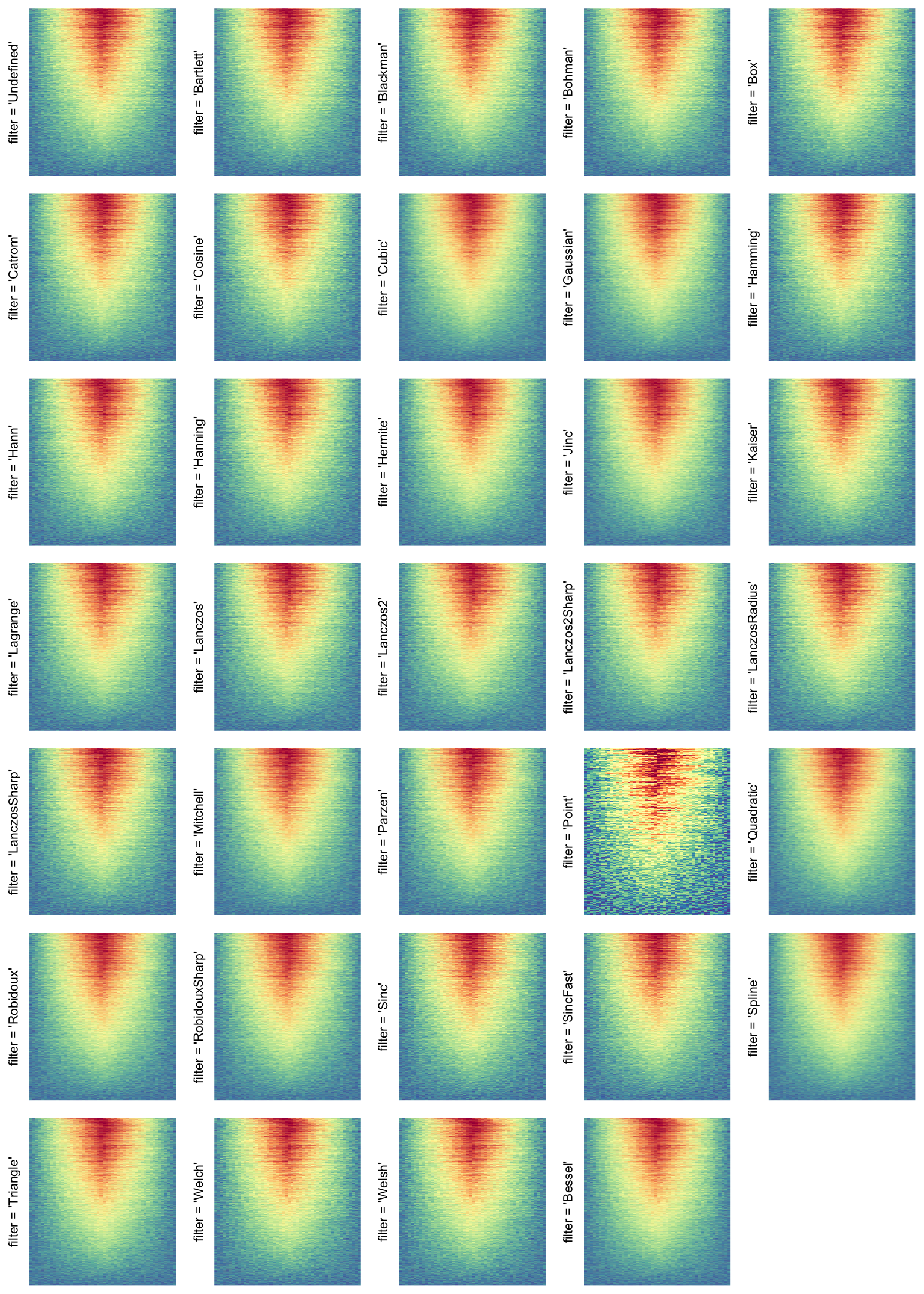And only add text to cells with positive values:

Heatmap(small_mat, name = "mat", col = col_fun,
layer_fun = function(j, i, x, y, width, height, fill) {
v = pindex(small_mat, i, j)
l = v > 0
grid.text(sprintf("%.1f", v[l]), x[l], y[l], gp = gpar(fontsize = 10))
})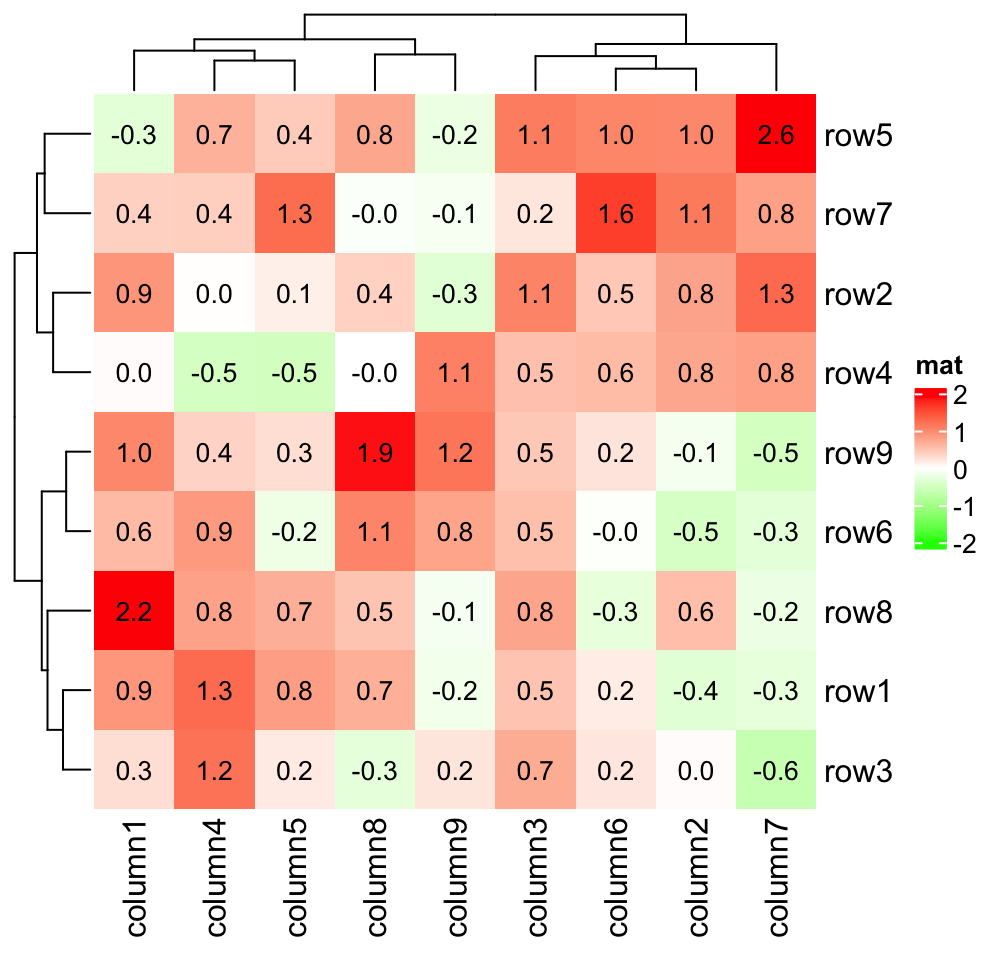When the heatmap is split, layer_fun is applied in every slice.

Heatmap(small_mat, name = "mat", col = col_fun,
row_km = 2, column_km = 2,
layer_fun = function(j, i, x, y, width, height, fill) {
v = pindex(small_mat, i, j)
grid.text(sprintf("%.1f", v), x, y, gp = gpar(fontsize = 10))
if(sum(v > 0)/length(v) > 0.75) {
grid.rect(gp = gpar(lwd = 2, fill = "transparent"))
}
})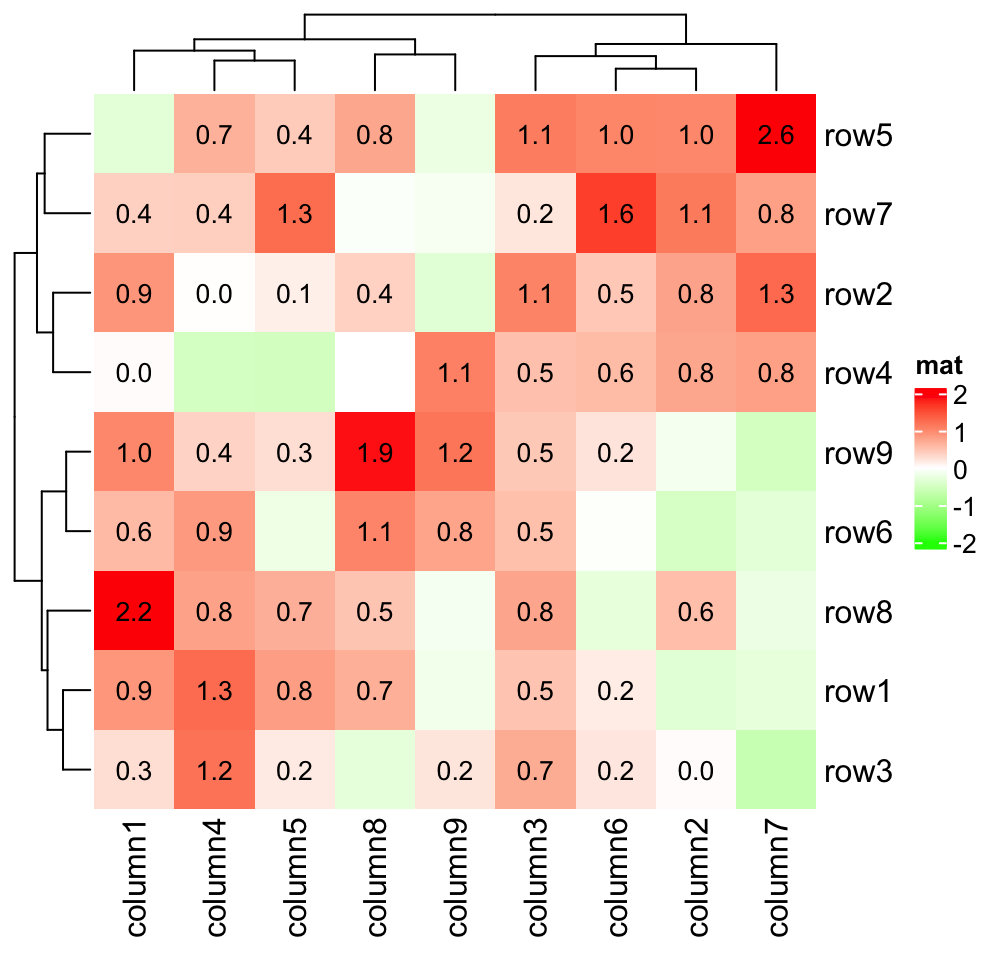layer_fun can also have two more arguments which are the index for the current row slice and column slice. E.g. we want to add borders for the top right and bottom left slices.

Heatmap(small_mat, name = "mat", col = col_fun,
row_km = 2, column_km = 2,
layer_fun = function(j, i, x, y, width, height, fill, slice_r, slice_c) {
v = pindex(small_mat, i, j)
grid.text(sprintf("%.1f", v), x, y, gp = gpar(fontsize = 10))
if(slice_r != slice_c) {
grid.rect(gp = gpar(lwd = 2, fill = "transparent"))
}
})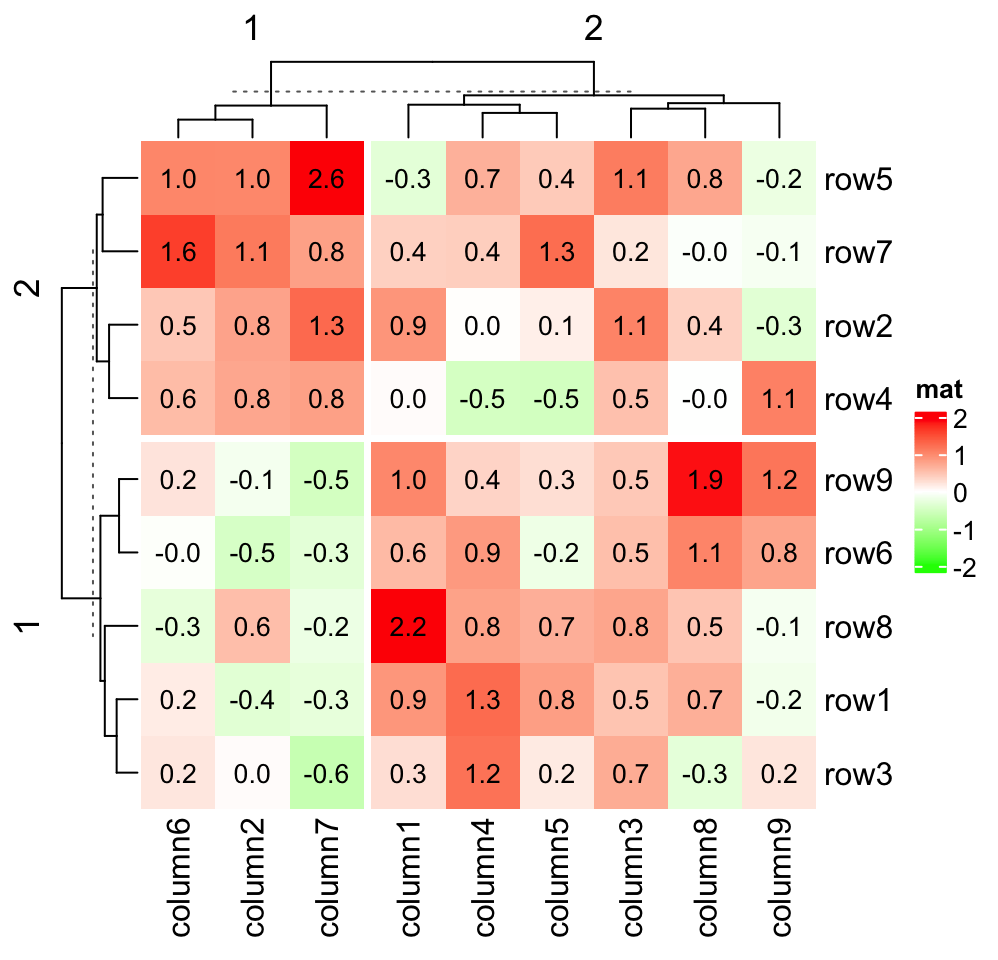The advantage of lay_fun is it is not only fast to add graphics, but also it provides more possibilities to customize the heatmap. Consider following visualization: For each row in the heatmap, if values in the neighbouring two columns have the same sign, we add a red line or a green line depending on the sign of the two values. (Don’t be frightened by following code. They are explained after the code.)

Heatmap(small_mat, name = "mat", col = col_fun,
row_km = 2, column_km = 2,
layer_fun = function(j, i, x, y, w, h, fill) {
# restore_matrix() is explained after this chunk of code
ind_mat = restore_matrix(j, i, x, y)
for(ir in seq_len(nrow(ind_mat))) {
# start from the second column
for(ic in seq_len(ncol(ind_mat))[-1]) {
ind1 = ind_mat[ir, ic-1] # previous column
ind2 = ind_mat[ir, ic]   # current column
v1 = small_mat[i[ind1], j[ind1]]
v2 = small_mat[i[ind2], j[ind2]]
if(v1 * v2 > 0) { # if they have the same sign
col = ifelse(v1 > 0, "darkred", "darkgreen")
grid.segments(x[ind1], y[ind1], x[ind2], y[ind2],
gp = gpar(col = col, lwd = 2))
grid.points(x[c(ind1, ind2)], y[c(ind1, ind2)],
pch = 16, gp = gpar(col = col), size = unit(4, "mm"))
}
}
}
}
)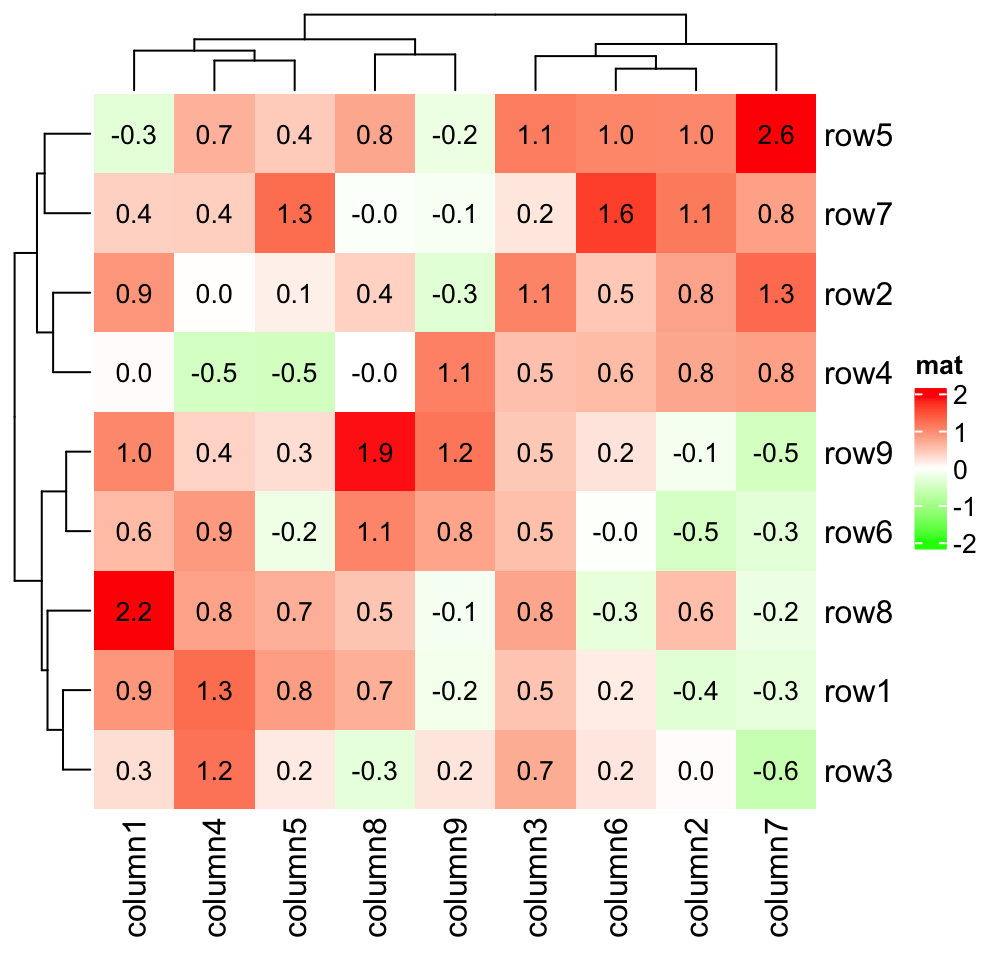The values that are sent to layer_fun are all vectors (for the vectorization of the grid graphic functions), however, the heatmap slice where layer_fun is applied to, is still represented by a matrix, thus, it would be very convinient if all the arguments in layer_fun can be converted to the sub-matrix for the current slice. Here, as shown in above example, restore_matrix() does the job. restore_matrix() directly accepts the first four argument in layer_fun and returns an index matrix, where rows and columns correspond to the rows and columns in the current slice, from top to bottom and from left to right. The values in the matrix are the natural order of e.g. vector j in current slice.

If you run following code:

Heatmap(small_mat, name = "mat", col = col_fun,
row_km = 2, column_km = 2,
layer_fun = function(j, i, x, y, w, h, fill) {
ind_mat = restore_matrix(j, i, x, y)
print(ind_mat)
}
)

The first output which is for the top-left slice:

     [,1] [,2] [,3] [,4] [,5]
[1,]    1    4    7   10   13
[2,]    2    5    8   11   14
[3,]    3    6    9   12   15

As you see, this is a three-row and five-column index matrix where the first row corresponds to the top row in the slice. The values in the matrix correspond to the natural index (i.e. 1, 2, …) in j, i, x, y, … in layer_fun. Now, if we want to add values on the second column in the top-left slice, the code which is put inside layer_fun would look like:

for(ind in ind_mat[, 2]) {
grid.text(small_mat[i[ind], j[ind]], x[ind], y[ind], ...)
}

Now it is easier to understand the second example: we want to add points to the second row and the third column in every slice:

Heatmap(small_mat, name = "mat", col = col_fun,
row_km = 2, column_km = 2,
layer_fun = function(j, i, x, y, w, h, fill) {
ind_mat = restore_matrix(j, i, x, y)
ind = unique(c(ind_mat[2, ], ind_mat[, 3]))
grid.points(x[ind], y[ind], pch = 16, size = unit(4, "mm"))
}
)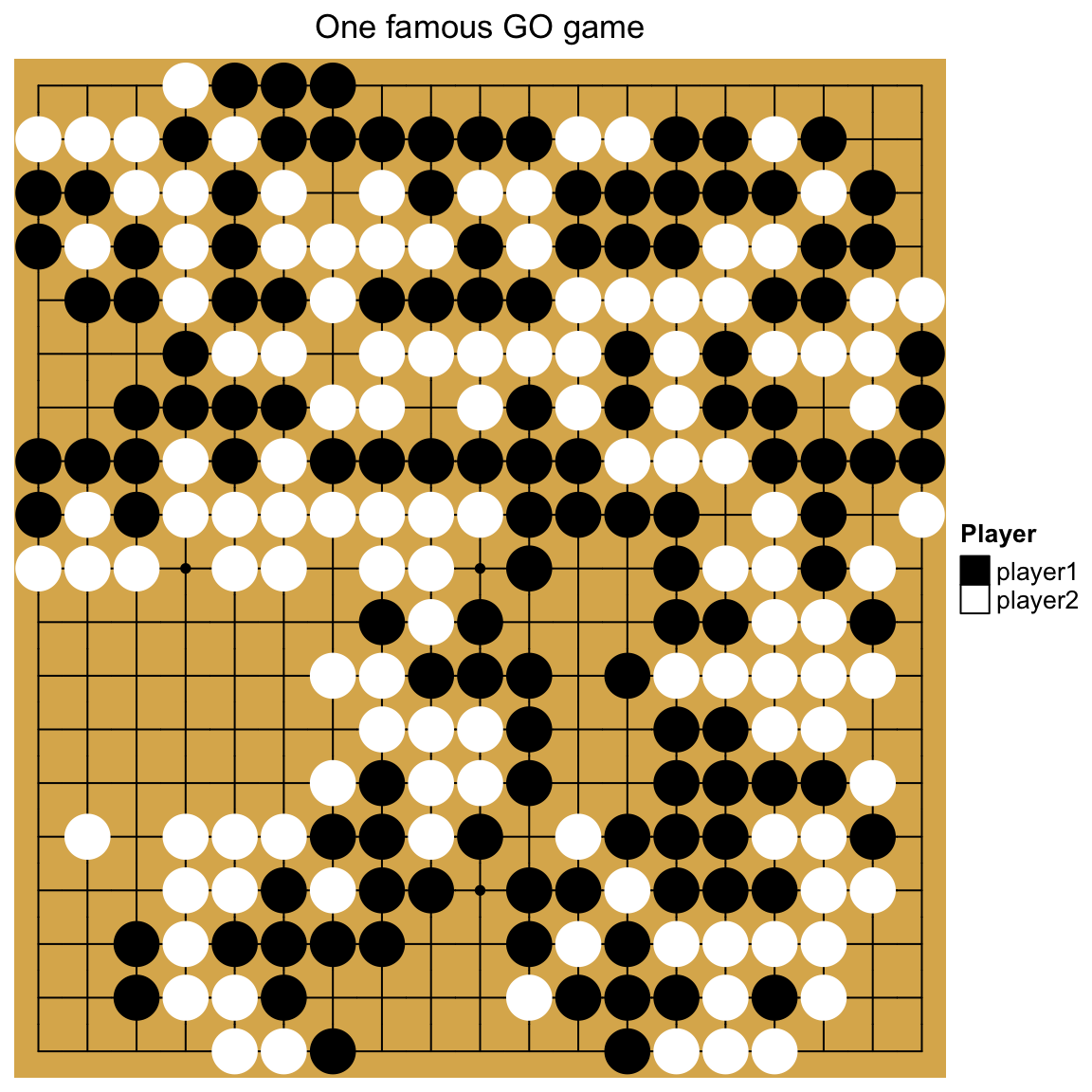## 2.10 Size of the heatmap

width, heatmap_width, height and heatmap_height control the size of the heatmap. By default, all heatmap components have fixed width or height, e.g. the width of row dendrogram is 1cm. The width or the height of the heatmap body fill the rest area of the final plotting region, which means, if you draw it in an interactive graphic window and you change the size of the window by draging it, the size of the heatmap body is automatically adjusted.

heatmap_width and heatmap_height control the width/height of the complete heatmap including all heatmap components (excluding the legends) while width and height only control the width/height of the heamtap body. All these four arguments can be set as absolute units.

Heatmap(mat, name = "mat", width = unit(8, "cm"), height = unit(8, "cm"))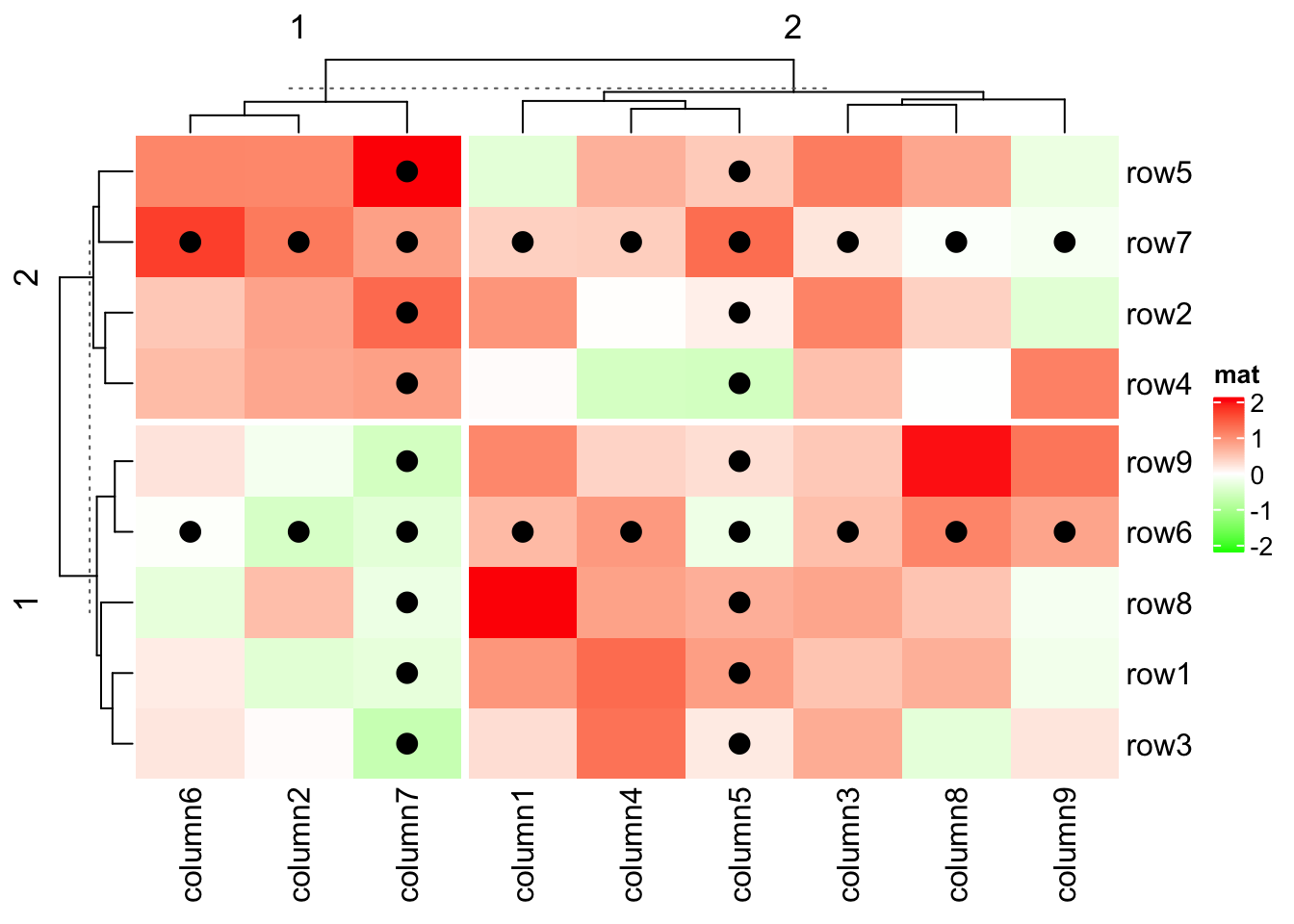Heatmap(mat, name = "mat", heatmap_width = unit(8, "cm"), heatmap_height = unit(8, "cm"))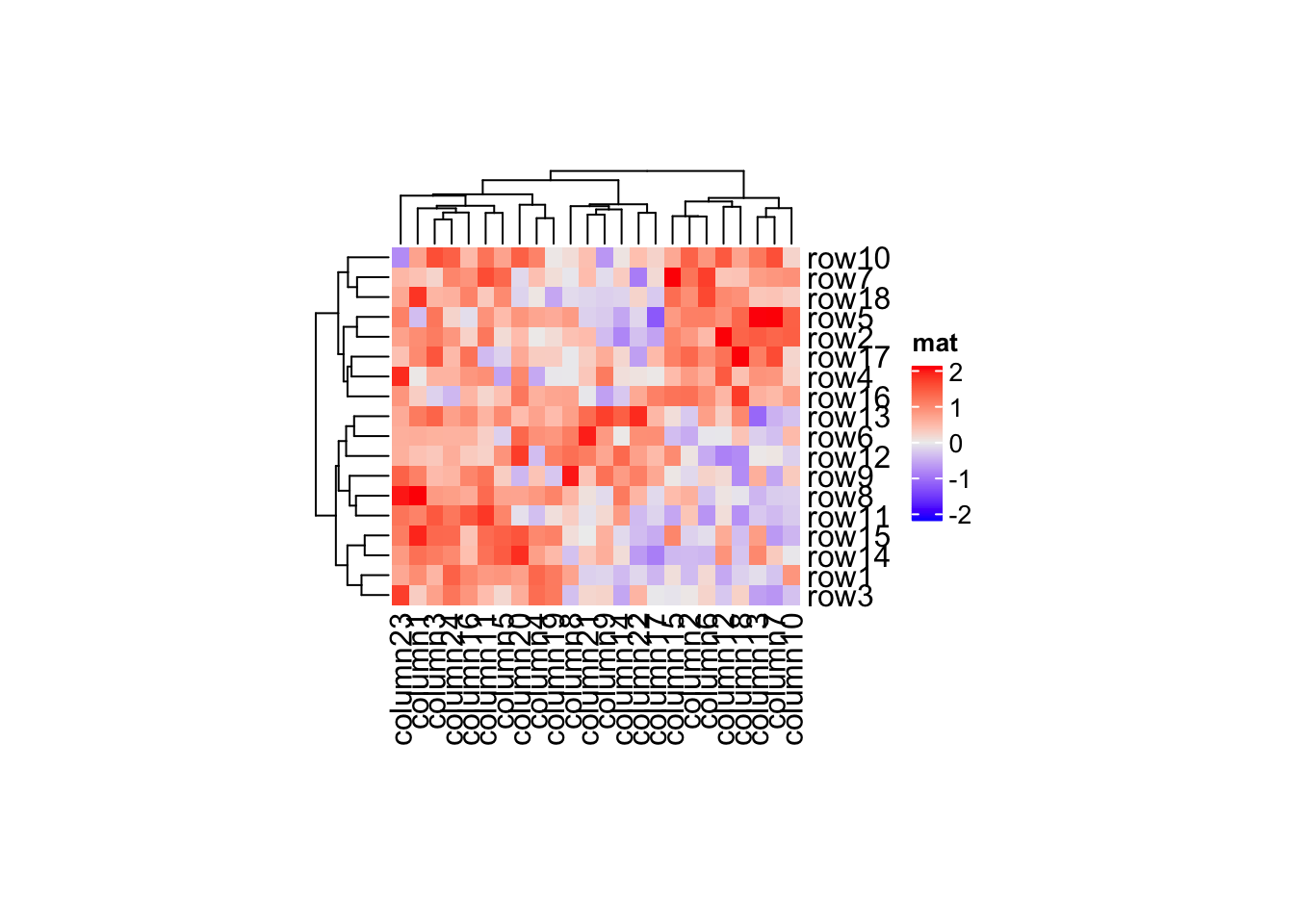These four arguments are more important when adjust the size in a list of heatmaps (see Section 4.2).

When the size of the heatmap is set as absolute units, it is possible that the size of the figure is larger than the size of the plot, which gives blank areas around the plot. The size of the heatmap can be retrieved by width() and height() functions.

ht = Heatmap(mat, name = "mat", width = unit(8, "cm"), height = unit(8, "cm"))
ht = draw(ht)
ComplexHeatmap:::width(ht)
##  118.985533333333mm
ComplexHeatmap:::height(ht)
##  114.8515mm
ht = Heatmap(mat, name = "mat", heatmap_width = unit(8, "cm"),
heatmap_height = unit(8, "cm"))
ht = draw(ht)
ComplexHeatmap:::width(ht)
##  95.0216666666667mm
ComplexHeatmap:::height(ht)
##  84mm

## 2.11 Plot the heatmap

Heatmap() function actually is only a constructor, which means it only puts all the data and configurations into the object in the Heatmap class. The clustering will only be performed when the draw() method is called. Under interactive mode (e.g. the interactive R terminal where you can type your R code line by line), directly calling Heatmap() without returning to any object prints the object and the print method (or the S4 show() method) for the Heatmap class object calls draw() internally. So if you type Heatmap(...) in your R terminal, it looks like it is a plotting function like plot(), you need to be aware of that it is actually not true and in the following cases you might see nothing plotted.

• you put Heatmap(...) inside a function,
• you put Heatmap(...) in a code chunk like for or if-else
• you put Heatmap(...) in an Rscript and you run it under command line.

The reason is in above three cases, the show() method WILL NOT be called and thus draw() method is not executed either. So, to make the plot, you need to call draw() explicitly: draw(Heatmap(...)) or:

# code only for demonstration
ht = Heatmap(...)
draw(ht)

The draw() function actually is applied to a list of heatmaps in HeatmapList class. The draw() method for the single Heatmap class constructs a HeatmapList with only one heatmap and call draw() method of the HeatmapList class. The draw() function accpets a lot of more arguments which e.g. controls the legends. It will be discussed in Chapter 4.

draw(ht, heatmap_legend_side, padding, ...)

## 2.12 Get orders and dendrograms

The row/column orders of the heatmap can be obtained by row_order()/column_order() functions. You can directly apply to the heatmap object returned by Heatmap() or to the object returned by draw(). In following, we take row_order() as example.

small_mat = mat[1:9, 1:9]
ht1 = Heatmap(small_mat)
row_order(ht1)
##  5 7 2 4 9 6 8 1 3
ht2 = draw(ht1)
row_order(ht2)
##  5 7 2 4 9 6 8 1 3

As explained in previous section, Heatmap() function does not perform clustering, thus, when directly apply row_order() on ht1, clustering will be first performed. Later when making the heatmap by draw(ht1), the clustering will be applied again. This might be a problem that if you set k-means clustering in the heatmap. Since the clustering is applied twice, k-means might give you different clusterings, which means, you might have different results from row_order() and you might have different heatmap.

In following chunk of code, o1, o2 and o3 might be different because each time, k-means clustering is performed.

# code only for demonstration
ht1 = Heatmap(small_mat, row_km = 2)
o1 = row_order(ht1)
o2 = row_order(ht1)
ht2 = draw(ht1)
o3 = row_order(ht2)
o4 = row_order(ht2)

draw() function returns the heatmap (or more precisely, the heatmap list) which has already been reordered, and applying row_order() just extracts the row order from the object, which ensures the row order is exactly the same as the one shown in the heatmap. In above code, o3 is always identical to o4.

So, the preferable way to get row/column orders is as follows.

# code only for demonstration
ht = Heatmap(small_mat)
ht = draw(ht)
row_order(ht)
column_order(ht)

If rows/columns are split, row order or column order will be a list.

ht = Heatmap(small_mat, row_km = 2, column_km = 3)
ht = draw(ht)
row_order(ht)
## $1 ##  9 6 8 1 3 ## ##$2
##  5 7 2 4
column_order(ht)
## $1 ##  6 2 7 ## ##$2
##  8 9
##
## $3 ##  1 3 4 5 Similarly, the row_dend()/column_dend() functions return the dendrograms. It returns a single dendrogram or a list of dendrograms depending on whether the heatmap is split. ht = Heatmap(small_mat, row_km = 2) ht = draw(ht) row_dend(ht) ##$1
## 'dendrogram' with 2 branches and 5 members total, at height 2.681351
##
## \$2
## 'dendrogram' with 2 branches and 4 members total, at height 2.946428
column_dend(ht)
## 'dendrogram' with 2 branches and 9 members total, at height 4.574114

row_order(), column_order(), row_dend() and column_dend() also work for a list of heatmaps, it will be introduced in Section 4.12.

## 2.13 Subset a heatmap

Since heatmap is a representation of a matrix, there is also a subset method for the Heatmap class.

ht = Heatmap(mat, name = "mat")
dim(ht)
##  18 24
ht[1:10, 1:10]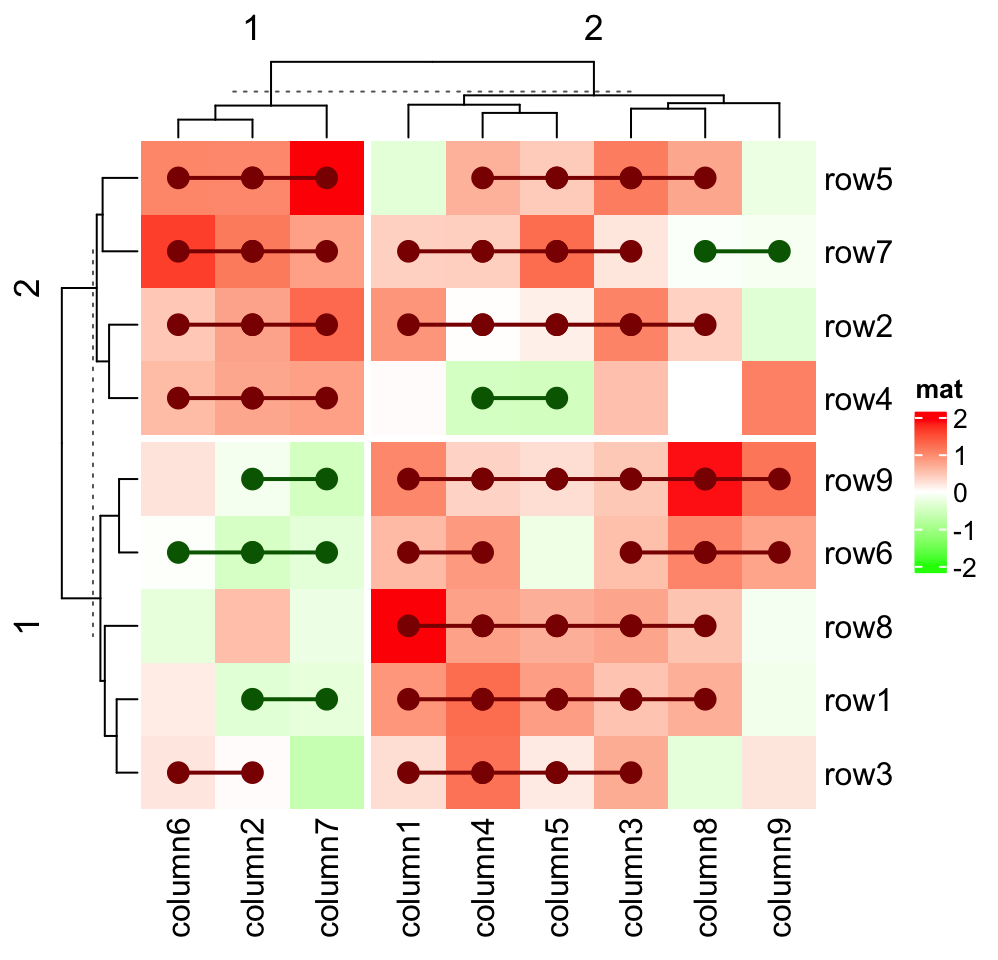The annotations are subsetted accordingly as well.

ht = Heatmap(mat, name = "mat", row_km = 2, column_km = 3,
col = colorRamp2(c(-2, 0, 2), c("green", "white", "red")),
top_annotation = HeatmapAnnotation(foo1 = 1:24, bar1 = anno_points(runif(24))),
right_annotation = rowAnnotation(foo2 = 18:1, bar2 = anno_barplot(runif(18)))
)
ht[1:9*2 - 1, 1:12*2] # odd rows, even columns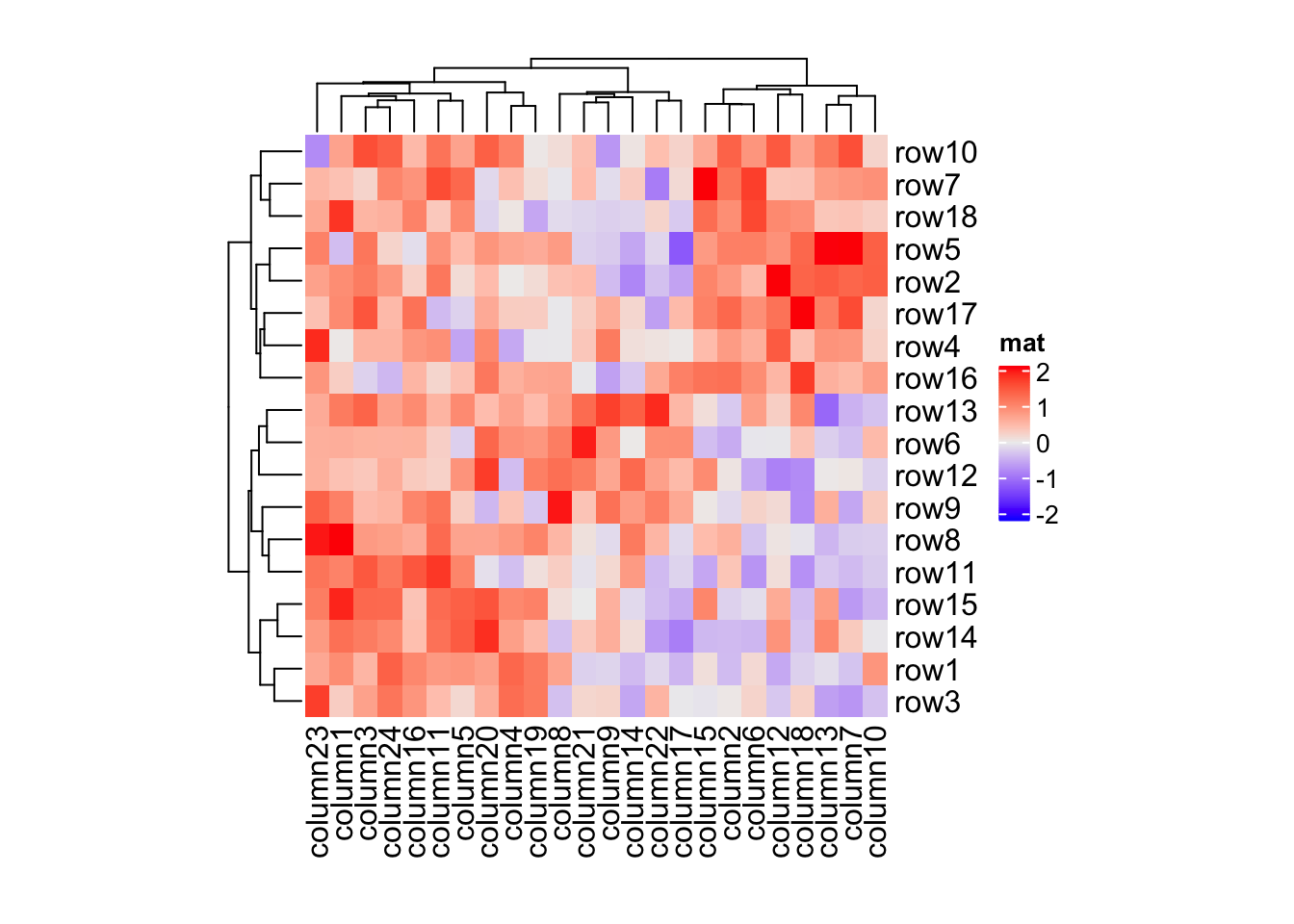The heatmap components are subsetted if they are vector-like. Some configurations in the heatmap keep the same when subsetting, e.g. if row_km is set in the original heatmap, the configuration of k-means is kept and it is performed in the sub-heatmap. So in following example, k-means clustering is only performed when making heatmap for ht2.

ht = Heatmap(mat, name = "mat", row_km = 2)
ht2 = ht[1:10, 1:10]
ht2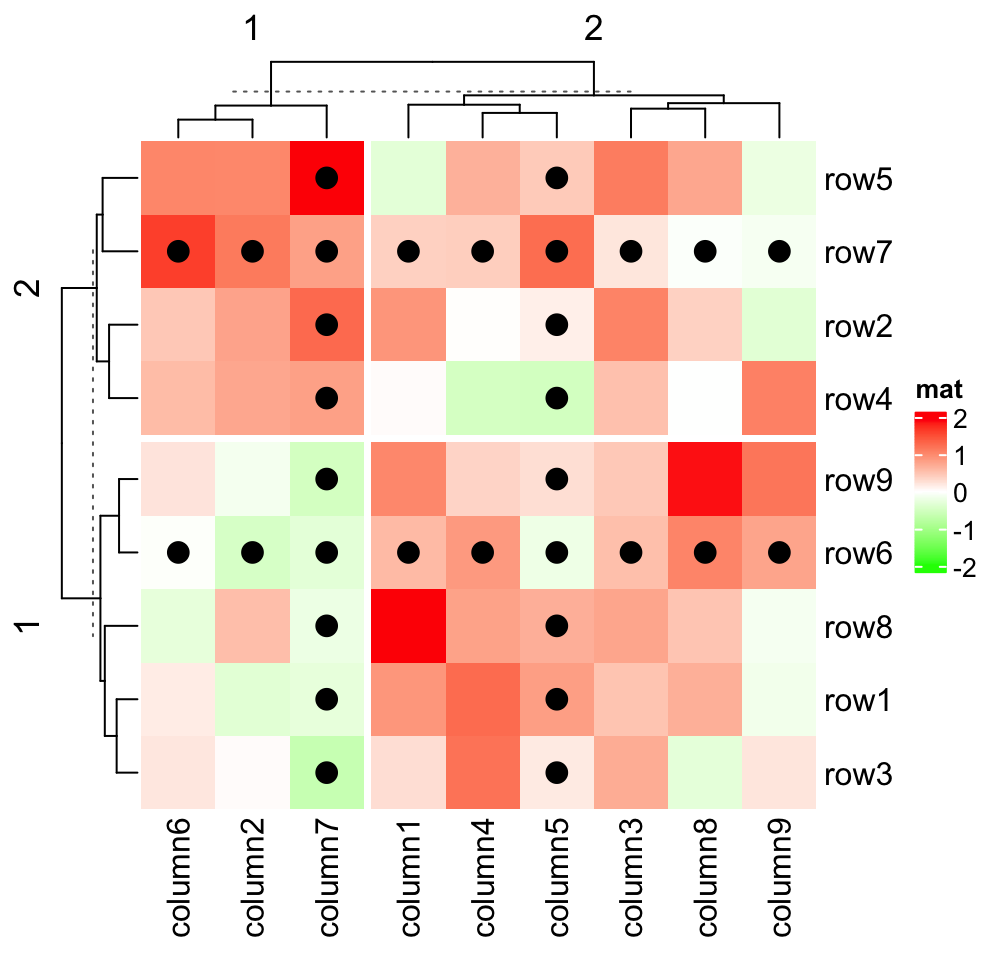The implementation of subsetting heatmaps is very experimental. It is not always working, e.g. if cell_fun is defined and uses an external matrix, or clustering objects are assigned to cluster_rows or cluster_columns.

There are also subset methods for the HeatmapAnnotation class (Section 3.19) and the HeatmapList class (Section 4.10), but both are very experimental as well.